# KR20110070758A - Pointer detection apparatus and pointer detection method - Google Patents

## Info

Publication number
KR20110070758A
KR20110070758A KR1020100094326A KR20100094326A KR20110070758A KR 20110070758 A KR20110070758 A KR 20110070758A KR 1020100094326 A KR1020100094326 A KR 1020100094326A KR 20100094326 A KR20100094326 A KR 20100094326A KR 20110070758 A KR20110070758 A KR 20110070758A
Authority
KR
South Korea
Prior art keywords
conductor
conductors
circuit
code
plurality
Prior art date
Application number
KR1020100094326A
Other languages
Korean (ko)
Other versions
KR101446373B1 (en
Inventor
요시히사 스기야마
사다오 야마모토
야스오 오다
Original Assignee
가부시키가이샤 와코무
Priority date (The priority date is an assumption and is not a legal conclusion. Google has not performed a legal analysis and makes no representation as to the accuracy of the date listed.)
Filing date
Publication date
Priority to JP2009288273A priority Critical patent/JP5295090B2/en
Priority to JPJP-P-2009-288273 priority
Application filed by 가부시키가이샤 와코무 filed Critical 가부시키가이샤 와코무
Publication of KR20110070758A publication Critical patent/KR20110070758A/en
Application granted granted Critical
Publication of KR101446373B1 publication Critical patent/KR101446373B1/en

• 238000001514 detection method Methods 0 title 1
• 239000004020 conductor Substances 0 abstract 5
• 230000000875 corresponding Effects 0 abstract 1
• 239000000284 extracts Substances 0 abstract 1

## Images

•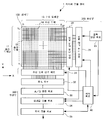•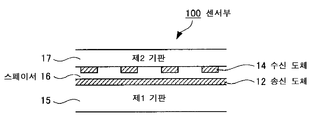•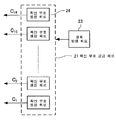••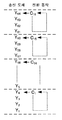•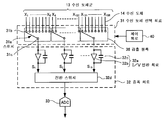••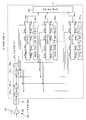•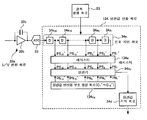•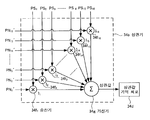•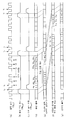•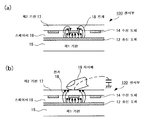•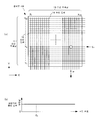•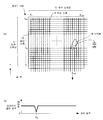••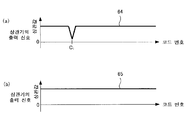•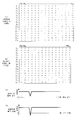•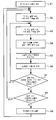•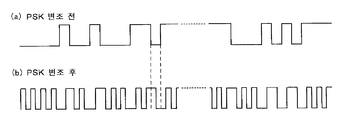•••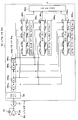•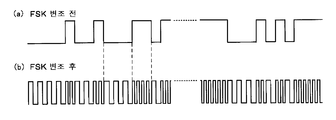•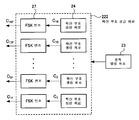•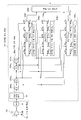•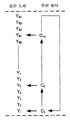•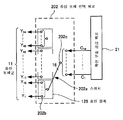•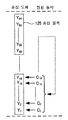•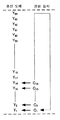•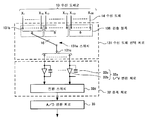•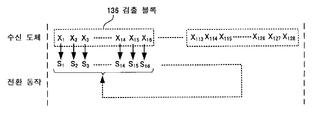•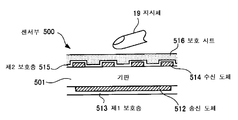••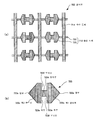•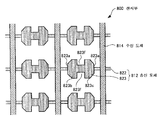••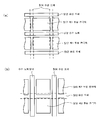•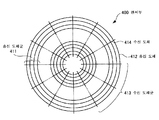•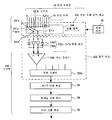•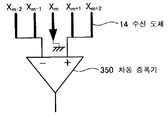•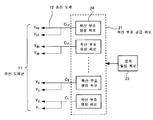••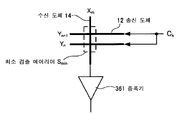•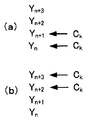•••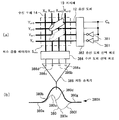•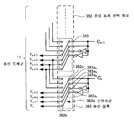••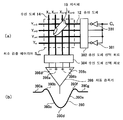•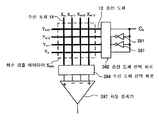••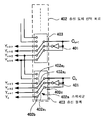•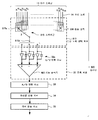•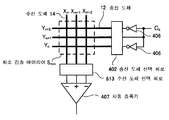•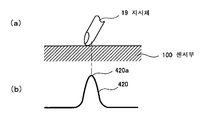•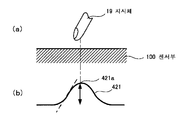•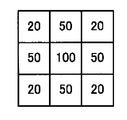•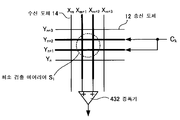•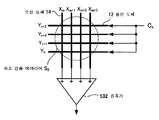•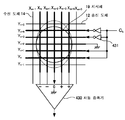•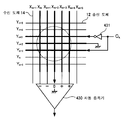•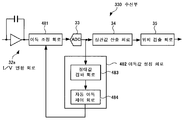•••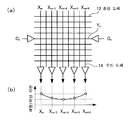•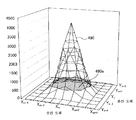••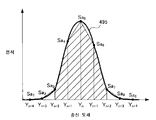•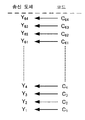•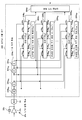•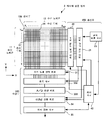•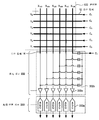•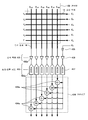•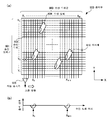## Classifications

• GPHYSICS
• G06COMPUTING; CALCULATING; COUNTING
• G06FELECTRIC DIGITAL DATA PROCESSING
• G06F3/00Input arrangements for transferring data to be processed into a form capable of being handled by the computer; Output arrangements for transferring data from processing unit to output unit, e.g. interface arrangements
• G06F3/01Input arrangements or combined input and output arrangements for interaction between user and computer
• G06F3/03Arrangements for converting the position or the displacement of a member into a coded form
• G06F3/033Pointing devices displaced or positioned by the user, e.g. mice, trackballs, pens or joysticks; Accessories therefor
• G06F3/0354Pointing devices displaced or positioned by the user, e.g. mice, trackballs, pens or joysticks; Accessories therefor with detection of 2D relative movements between the device, or an operating part thereof, and a plane or surface, e.g. 2D mice, trackballs, pens or pucks
• G06F3/03545Pens or stylus
• GPHYSICS
• G06COMPUTING; CALCULATING; COUNTING
• G06FELECTRIC DIGITAL DATA PROCESSING
• G06F3/00Input arrangements for transferring data to be processed into a form capable of being handled by the computer; Output arrangements for transferring data from processing unit to output unit, e.g. interface arrangements
• G06F3/01Input arrangements or combined input and output arrangements for interaction between user and computer
• G06F3/03Arrangements for converting the position or the displacement of a member into a coded form
• G06F3/041Digitisers, e.g. for touch screens or touch pads, characterised by the transducing means
• G06F3/0416Control or interface arrangements specially adapted for digitisers
• GPHYSICS
• G06COMPUTING; CALCULATING; COUNTING
• G06FELECTRIC DIGITAL DATA PROCESSING
• G06F3/00Input arrangements for transferring data to be processed into a form capable of being handled by the computer; Output arrangements for transferring data from processing unit to output unit, e.g. interface arrangements
• G06F3/01Input arrangements or combined input and output arrangements for interaction between user and computer
• G06F3/03Arrangements for converting the position or the displacement of a member into a coded form
• G06F3/041Digitisers, e.g. for touch screens or touch pads, characterised by the transducing means
• G06F3/044Digitisers, e.g. for touch screens or touch pads, characterised by the transducing means by capacitive means
• GPHYSICS
• G06COMPUTING; CALCULATING; COUNTING
• G06FELECTRIC DIGITAL DATA PROCESSING
• G06F3/00Input arrangements for transferring data to be processed into a form capable of being handled by the computer; Output arrangements for transferring data from processing unit to output unit, e.g. interface arrangements
• G06F3/01Input arrangements or combined input and output arrangements for interaction between user and computer
• G06F3/03Arrangements for converting the position or the displacement of a member into a coded form
• G06F3/041Digitisers, e.g. for touch screens or touch pads, characterised by the transducing means
• G06F3/046Digitisers, e.g. for touch screens or touch pads, characterised by the transducing means by electromagnetic means
• GPHYSICS
• G06COMPUTING; CALCULATING; COUNTING
• G06FELECTRIC DIGITAL DATA PROCESSING
• G06F2203/00Indexing scheme relating to G06F3/00 - G06F3/048
• G06F2203/041Indexing scheme relating to G06F3/041 - G06F3/045
• G06F2203/04111Cross over in capacitive digitiser, i.e. details of structures for connecting electrodes of the sensing pattern where the connections cross each other, e.g. bridge structures comprising an insulating layer, or vias through substrate

## Abstract

PURPOSE: An apparatus and method for detecting a pointer is provided to rapidly extract the existence and the position of the pointers by extracting the pointer located on the surface the conductor pattern based on the relative operation result. CONSTITUTION: A symbol supplying circuit includes a plurality of symbol rows which have different symbol with each other and supply the symbol rows to the conductors arranged as a first direction comprising the conductor pattern. A relation value computing symbol supplying circuit supplies the relation value computing symbol corresponding to the symbol rows, A relation value computing circuit(34) performs the relating operation between the signal of the conductors arranged as a second direction and the relation value computing symbol. The pointer located on the surface of the conductor pattern is extracted on the basis of the result of the relating operation.

## Description

Indicator detection device and indicator detection method {POINTER DETECTION APPARATUS AND POINTER DETECTION METHOD}

The present invention relates to an indicator detection apparatus and an indicator detection method, and more particularly, to an indicator detection apparatus and an indicator detection method capable of detecting a plurality of indicators at high speed.

Background Art Conventionally, various sensor methods, such as a resistive film method and an electrostatic coupling method (capacitive method), have been proposed as a method of detecting the position of an indicator such as a finger or an exclusive pen used in a touch panel. Among them, in recent years, development of an indicator detection device of an electrostatic coupling method has been actively performed.

There are two types of electrostatic coupling methods: surface capacitive type and projected capacitive type. The surface type is applied to, for example, an automated teller machine (ATM) and the like, and the projection type is applied to, for example, a mobile phone. In addition, both methods detect the position of the indicator by detecting a change in the electrostatic coupling state between the sensor electrode and the indicator (for example, a finger, an electrostatic pen, etc.).

The indicator detection device of the projection type electrostatic coupling method is formed by forming electrodes in a predetermined pattern on a transparent substrate or transparent film, for example, and detects a change in the state of the electrostatic coupling between the indicator and the electrode when the indicator approaches. do. Conventionally, various techniques for optimizing the structure of the indicator detection device of such a system have been proposed (see Patent Documents 1 to 3, for example). In addition, Patent Document 1 describes a technique of applying a code division multiplexing method using an orthogonal spreading code to a multi-user touch system. Patent Document 2 describes a coordinate input device using a pseudo random signal. In addition, Patent Document 3 describes a pen used in a capacitive coordinate device.

Moreover, the indicator detection apparatus of the system called the cross point electrostatic coupling system which developed the projection type electrostatic coupling system conventionally is proposed conventionally. Here, the operation of the indicator detection device of the cross point electrostatic coupling method will be briefly described with reference to the drawings. 75 (a) and (b) show schematic structures and output signal waveforms in the vicinity of the sensor unit in the indicator detection device of the cross point electrostatic coupling method, respectively.

In general, the sensor unit 900 includes a transmission conductor group 901 composed of a plurality of transmission conductors 902 and a reception conductor group 903 composed of a plurality of reception conductors 904. An insulating layer is formed between the transmitting conductor group 901 and the receiving conductor group 903. The transmission conductors 902 are conductors having a predetermined shape extending in a predetermined direction (the X direction in Fig. 75 (a)), and the plurality of transmission conductors 902 are arranged in parallel with a predetermined distance from each other. In addition, the receiving conductor 904 is a conductor having a predetermined shape extending in the direction crossing the stretching direction of the transmitting conductor 902 (Y direction in FIG. 75 (a)), and the plurality of receiving conductors 904 are mutually different. It is arranged in parallel with a predetermined distance distance.

In the indicator detection apparatus using the sensor unit 900 having such a configuration, for example, a predetermined signal is supplied to a predetermined transmission conductor 902, and the transmission conductor 902 and the reception conductor (to which the predetermined signal is supplied) are supplied. The change in the current flowing through the intersection point (hereinafter referred to as cross point) of 904 is detected for every cross point. Here, at the position where the indicator 910 such as a finger is placed on the sensor unit 900, a part of the current flowing through the transmission conductor 902 is classified through the indicator 910 so that the reception conductor 904 is provided. The incoming current changes. Therefore, the position of the indicator 910 can be detected by detecting the cross point of the transmission conductor 902 which supplied the signal and the reception conductor 904 which a current changes. In addition, since the current change is detected for each of the plurality of cross points formed on the sensor unit 900 in the indicator detecting apparatus of the cross point electrostatic coupling method, the plurality of indicators can be detected at the same time.

Here, more specifically, the principle of the position detection of the cross point electrostatic coupling method will be described. The detection of the pointed position of, (for example, finger 910), for example as shown in Fig. 75 (a), the transmission conductors (Y 6) to the indicating device on a predetermined supply signal, and transmitting conductors (Y 6) Think. First, in the state where a signal is supplied to the transmission conductor Y 6 , the differential amplifier 905 detects a difference in the current flowing through each of the reception conductors X 1 and X 2 . Subsequently, the switch at a predetermined time after, receiving conductors (X 2 and X 3) for receiving the conductor to be connected to the differential amplifier 905, and a positive reception conductors (X 2 and X 3) detecting a difference between the current flowing in each of the do. This operation is repeated up to the receiving conductor X M.

Then, the level change of the output signal of the differential amplifier 905 at each cross point of the transmitting conductor Y 6 and the receiving conductor is obtained. 75 (b) shows the characteristic. Here, the horizontal axis of the characteristic of FIG. 75 (b) shows a detection signal outputted by the reception conductors X 1 to X m being sequentially selected in time and connected to the differential amplifier 905. In addition, the characteristic shown by the broken line in FIG. 75 (b) actually shows the level change of the signal output from the differential amplifier 905, and the solid line characteristic shows the change of the integral value of the output signal of the differential amplifier 905. .

As shown in Fig. 75 (a), since the indicator 910 (finger) is placed near the cross point of the transmitting conductor Y 6 and the receiving conductors X 5 and X M -5 , the current flowing near the cross point. Changes. Therefore, as shown in Fig. 75B, the output signal of the differential amplifier 905 changes at a position corresponding to the vicinity of the cross point of the receiving conductors X 5 and X M -5 on the transmitting conductor Y 6 . The integral value changes. The position of the indicator 910 can be detected based on the change of the integral value. The conventional indicator detection device performs detection as described above while switching the transmission conductors 902 one by one.

Prior art literature

Patent Literature

Patent Document 1: Japanese Patent Application Laid-Open No. 2003-22158

Patent Document 2: Japanese Patent Application Laid-Open No. 9-222947

Patent Document 3: Japanese Patent Laid-Open No. 10-161795

By the way, the above-mentioned conventional cross-point electrostatic coupling type indicator detecting apparatus performs signal supply and reception processing for each transmitting conductor and receiving conductor constituting each cross point, and performs the position detection processing of the indicator. For this reason, if the position detection process is performed for all the cross points, there is a problem that the process requires a long time. For example, in the sensor unit including 64 transmitting conductors and 128 receiving conductors, if the detection processing time at each cross point is 256 µsec, the detection time of about 2 sec is taken at all cross points (8192). Not practical

In view of the above problems, an object of the present invention is to provide an indicator detecting apparatus and an indicator detecting method capable of detecting an indicator at a higher speed.

The indicator detection device of the present invention is an indicator detection for detecting an indicator located on a conductor pattern comprising a plurality of conductors arranged in a first direction and a plurality of conductors arranged in a second direction crossing with respect to the first direction. An apparatus, comprising: a code supply circuit for supplying a predetermined code string to each of a plurality of conductors having a plurality of code strings different from each other and arranged in a first direction constituting a conductor pattern; A correlation value calculation code supply circuit for supplying a correlation value calculation code corresponding to each of the plurality of code strings; A correlation calculation circuit for correlating a signal generated in each conductor arranged in the second direction and a code for correlation value calculation, and an indicator positioned on the conductor pattern based on a correlation calculation result obtained by the correlation calculation circuit. Detection.

Moreover, the indicator detection method of this invention is a some conductor arrange | positioned in a 1st direction. An indicator detection method for detecting an indicator located on a conductor pattern composed of a plurality of conductors arranged in a second direction crossing with respect to a first direction, comprising a plurality of code strings having different codes from each other to form a conductor pattern. A code supply step of supplying a predetermined code string to each of the plurality of conductors arranged in the first direction to be specified; A correlation value calculation code supply step of supplying a correlation value calculation code corresponding to each of the plurality of code strings; A correlation calculation processing step for correlating a signal generated in each conductor arranged in the second direction and a sign for correlation value calculation, and located on the conductor pattern based on the correlation calculation result obtained by the correlation calculation processing step. Indicators were detected.

In the present invention, a code supply circuit for supplying a predetermined code string to each of a plurality of conductors having a plurality of code strings different from each other and arranged in a first direction constituting a conductor pattern, and each of the plurality of code strings A correlation calculation code supply circuit for supplying a correlation calculation code corresponding to the correlation calculation circuit, and a correlation calculation circuit for correlation between a signal generated in each conductor arranged in the second direction and the correlation calculation code; The indicator located on the conductor pattern was detected based on the correlation calculation result obtained by the circuit. Therefore, according to the present invention, it is possible to simultaneously detect the presence of a plurality of indicators and their indication positions at a higher speed in the indicator detection apparatus of the cross point electrostatic coupling method.

BRIEF DESCRIPTION OF THE DRAWINGS It is a schematic block block diagram of the indicator detection apparatus which concerns on 1st Embodiment of this invention.
2 is a schematic cross-sectional view of a sensor unit of the indicator detecting device according to the first embodiment.
3 is a schematic configuration diagram of a spread code supply circuit of the indicator detection device according to the first embodiment.
4 is a schematic configuration diagram of a transmission conductor selection circuit of the indicator detection device according to the first embodiment.
5 is an explanatory diagram of a spread code switching operation in a transmitting unit of the indicator detecting apparatus according to the first embodiment.
6 is a schematic configuration diagram of a reception conductor selection circuit of the indicator detection device according to the first embodiment.
It is explanatory drawing for demonstrating the switching operation of the reception conductor of a reception conductor selection circuit in the indicator detection apparatus which concerns on 1st Embodiment.
8 is a block configuration diagram of a correlation value calculation circuit of the indicator detection device according to the first embodiment.
9 is a block diagram of a correlation value calculation circuit that performs correlation calculation by time division of the indicator detection device according to the first embodiment.
It is a block block diagram which shows the internal structural example of the correlator of the indicator detection apparatus which concerns on 1st Embodiment.
11A to 11G are time charts for explaining the operation of each part of the indicator detecting device according to the first embodiment.
It is explanatory drawing for demonstrating the principle of position detection in the indicator detection apparatus which concerns on 1st Embodiment.
It is explanatory drawing for demonstrating the principle (state without a indicator) of position detection in the indicator detection apparatus which concerns on 1st Embodiment.
It is explanatory drawing for demonstrating the principle (state with a indicator) of position detection in the indicator detection apparatus which concerns on 1st Embodiment.
FIG. 15 is an explanatory diagram for explaining the principle of position detection (state with indicators at a plurality of cross points) in the indicator detection device according to the first embodiment. FIG.
Figure 16 is an explanatory view showing an example of the output signal is detected in the state shown in FIG. 15, FIG. 16 (a) is a diagram showing the relationship between the output signal and the code number that is output from the transmitting electrodes (Y 1 ~ Y 4) 16 (b) is a diagram showing a relationship between an output signal output from the transmission electrodes Y 5 to Y 64 and a code number.
FIG. 17A is a diagram showing an example of a 16 chip length Hadamard code, FIG. 17B is a diagram showing an example of a 15 chip length Hadamard code, and FIG. Fig. 17 (d) is a diagram showing the relationship between the output signal and the code number obtained when the 16-chip length Adama code shown in (a) is supplied. Is a diagram showing a relationship between and a code number.
18 is a flowchart showing a processing procedure of position detection in the indicator detecting apparatus according to the first embodiment.
19 (a) is a spread code waveform diagram before PSK modulation in the second embodiment of the present invention, and FIG. 19 (b) is a spread code waveform diagram after PSK modulation.
20 is a schematic block configuration diagram of an indicator detection device according to a second embodiment.
Fig. 21 is a schematic configuration diagram of a spread code supply circuit of the indicator detection device according to the second embodiment.
It is a block block diagram of the correlation value calculation circuit of the indicator detection apparatus which concerns on 2nd Embodiment.
FIG. 23A is a spread code waveform diagram before FSK modulation in a third embodiment of the present invention, and FIG. 23B is a spread code waveform diagram after FSK modulation.
24 is a schematic configuration diagram of a spread code supply circuit of the indicator detection device according to the third embodiment.
Fig. 25 is a block configuration diagram of a correlation value calculation circuit of the indicator detection device according to the third embodiment.
FIG. 26 is an explanatory diagram for explaining a spreading code supply method in a first modification. FIG.
27 is a schematic configuration diagram of a transmission conductor selection circuit in Modification Example 2. FIG.
28 is an explanatory diagram for explaining a spreading code supply method in a second modification.
29 is an explanatory diagram for explaining a spreading code supply method in a third modification.
30 is a block diagram of a receiving conductor selection circuit in a fourth modification.
31 is an explanatory diagram for explaining a switching operation of the reception conductor selection circuit in modified example 4. FIG.
32 is a schematic sectional view of a sensor unit according to Modification Example 5. FIG.
FIG. 33A is a schematic sectional view of a sensor unit according to Modification Example 6, and FIG. 33B is a perspective view illustrating an arrangement example of the sensor unit according to Modification Example 6. FIG.
FIG. 34A is a plan view showing a schematic configuration of a transmission conductor and a reception conductor according to Modification Example 7, and FIG. 34B is an enlarged plan view of a land conductor portion of the transmission conductor according to Modification Example 7. FIG.
35 is a schematic plan view of a sensor unit according to Modification Example 8. FIG.
36 is a schematic plan view of a sensor unit according to Modification Example 9. FIG.
FIG. 37 (a) is a schematic block diagram showing the arrangement of the transparent electrode film of the transmission conductor in the sensor unit according to the modification 9, and FIG. 37 (b) is a schematic block diagram showing the arrangement of the transparent electrode film of the receiving conductor.
38 is a schematic configuration diagram of a sensor unit of Modification Example 10;
39 is a block diagram of a receiver of a modification 11.
40 is a schematic configuration diagram of a differential amplifier of Modification 12;
Fig. 41 is a schematic configuration diagram for explaining a spreading code supply method according to a modification 13.
42 is a schematic configuration diagram of a sensor unit of Modified Example 14. FIG.
43 is a schematic configuration diagram of a sensor unit for comparison with Modification Example 14. FIG.
It is explanatory drawing which shows the example of the switching state of the transmission conductor of the modification 14.
It is explanatory drawing which shows the other example of the switching state of the transmission conductor of the modification 14.
46 is a schematic diagram illustrating a relationship between a transmission form of a spread code of a transmitter and a reception form of a signal of a receiver in a modification 15. FIG.
FIG. 47 is a configuration diagram showing a relationship between a transmission form of a spread code of a transmitter and a reception form of a signal of a receiver in a modification 16; FIG.
48 is a configuration diagram illustrating an example of an internal configuration of a transmission conductor selection circuit in Modification Example 16. FIG.
FIG. 49 is a configuration diagram illustrating an example of a reception conductor selection circuit in Modification Example 16. FIG.
FIG. 50A is a configuration diagram showing a relationship between a transmission form of a spread code of a transmitter and a reception form of a signal of a receiver in Modification 17, and FIG. 50B is a waveform diagram of an output signal output from a differential amplifier. .
FIG. 51 is a configuration diagram showing a relationship between a transmission form of a spread code of a transmitter and a reception form of a signal of a receiver in a modification 18; FIG.
52 is a block diagram showing the relationship between the transmission form of the spreading code of the transmission unit and the reception form of the signal of the reception unit in Modification Example 19. FIG.
53 is a block diagram illustrating an example of a transmission conductor selection circuit in a modification 19;
54 is a block diagram illustrating an example of a receiver of a modification 19;
FIG. 55 is a configuration diagram showing a relationship between a transmission form of a spread code of a transmitter and a reception form of a signal of a receiver in a modification 20; FIG.
56A and 56B are explanatory diagrams for explaining a principle of identifying a hovering state in a modification 21;
57A and 57B are explanatory diagrams for explaining a principle of identifying a hovering state in a modification 21;
Fig. 58 is a distribution diagram for explaining a principle of identifying a hovering state in a modification 21;
FIG. 59 is a configuration diagram showing a relationship (a state where a detection area is narrow) between a transmission form of a spread code of a transmitter and a reception form of a signal of a receiver in a modification 22; FIG.
FIG. 60 is a configuration diagram showing a relationship (a state where a detection area is wide) between a transmission form of a spread code of a transmitter and a reception form of a signal of a receiver in a modification 22; FIG.
61 is a block diagram showing the relationship between the transmission form of the spreading code of the transmission unit and the reception form of the signal of the reception unit in the modification 24. FIG.
FIG. 62 is a configuration diagram showing a relationship between a transmission form of a spread code of a transmitter and a reception form of a signal of a receiver in a modification 25; FIG.
Fig. 63 is a block diagram of a receiver example in Modification 26;
64 is a block diagram illustrating an example of an absolute value detection circuit in Modified Example 26. FIG.
Fig. 65 (a) is an explanatory diagram showing the form when a spread code is supplied from one side of the transmitting conductor, and Fig. 65 (b) shows the ratio of the position of the receiving conductor and the level and phase delay of the detection signal in that case. It is explanatory drawing which shows the relationship with (level / phase).
FIG. 66 (a) is an explanatory diagram showing a form in the case where a spread code is supplied from both sides of a transmitting conductor in Modification 27, and FIG. 66 (b) shows the position of the receiving conductor and the detection signal in that case. It is explanatory drawing which shows the relationship with the ratio (level / phase) of a level and phase delay.
FIG. 67 is a characteristic diagram for explaining the principle of obtaining the indicated pressure of the indicator in the modification 28; FIG.
FIG. 68 is a characteristic diagram for explaining the principle of obtaining the indicated pressure of the indicator in the modification 28; FIG.
FIG. 69 is a characteristic view for explaining the principle of obtaining the indicated pressure of the indicator in the modification 28; FIG.
Fig. 70 is an explanatory diagram showing a modification example of preparing the spreading codes by the number of transmitting electrodes as a variation 29;
71 is a block diagram illustrating an example of a correlation value calculation circuit in a modification 29. FIG.
72 is a configuration diagram of an indicator detecting device in Modified Example 30. FIG.
73 is a schematic configuration diagram of a receiver according to a modification 30;
74 is a schematic configuration diagram of a receiver according to a modification 31;
Fig. 75A is a schematic configuration diagram of a conventional cross point electrostatic coupling type indicator detecting device, and Fig. 75B is an output signal waveform diagram.

EMBODIMENT OF THE INVENTION Hereinafter, embodiment of the indicator detection apparatus and indicator detection method of this invention is described in the following order, referring drawings. In addition, although the indicator detection apparatus is demonstrated to the following embodiment as an example, this invention is not limited to this embodiment, As long as it is an apparatus which detects the indicator which contacted or contacted and performs some process, it is applicable also to other things.

1. First embodiment: Basic configuration example

2. Second embodiment: Configuration example using PSK modulated spreading code

3. Third Embodiment Configuration Example Using FSK Modulated Spread Code

4. Fourth embodiment: Another supply method of spreading code

5. Fifth embodiment: Selection method of receiving conductor

6. Sixth embodiment: Other structural example of sensor part

7. Seventh embodiment: Another configuration example of an amplifier circuit

8. Eighth embodiment: Hovering detection

<1. First embodiment: Basic constitution example>

The basic structural example of the indicator detection apparatus and indicator detection method of this invention is demonstrated with reference to FIGS. Further, the position detection method of the present invention adopts an electrostatic coupling method for detecting the position of the indicator based on the change of the electrostatic coupling state between the transmitting conductor and the receiving conductor of the sensor unit. In the present embodiment, a configuration example in which spreading codes (code strings) are simultaneously supplied to all transmission conductors and signal detection is simultaneously performed at each reception conductor will be described.

[Configuration of Indicator Detection Device]

1, the schematic block diagram of the indicator detection apparatus in 1st Embodiment is shown.

The indicator detecting apparatus 1 mainly comprises a control circuit 40 for controlling operations of the sensor unit 100, the transmitter 200, the receiver 300, the transmitter 200, and the receiver 300. Hereinafter, the structure of each part is demonstrated.

First, the configuration of the sensor unit 100 will be described with reference to FIGS. 1 and 2. The sensor unit 100 includes a first flat plate 15 having a substantially flat plate shape as shown in FIG. 2, a transmission conductor group 11 composed of a plurality of transmission conductors 12, and a reception conductor composed of a plurality of reception conductors 14. A group 13, a spacer 16, and a flat second substrate 17 are provided. The sensor unit 100 is formed by sequentially placing the transmitting conductor 12, the spacer 16, the receiving conductor 14, and the second substrate 17 on the first substrate 15. Thus, the transmitting conductor 12 and the receiving conductor 14 are arranged to face through the spacer 16.

An indicator such as a finger or an electrostatic pen is used on the second substrate 17 side (the side opposite to the surface on which the second substrate 17 faces the first substrate 15). Therefore, the receiving conductor 14 is arranged closer to the detection surface than the transmitting conductor 12. In addition, although the well-known glass substrate which has a permeability is used for the 1st board | substrate 15 and the 2nd board | substrate 17, the sheet-like (film-form) base material which consists of synthetic resin etc. instead of a glass substrate ( May be used.

The transmitting conductor 12 and the receiving conductor 14 are formed of a transparent electrode film made of, for example, an Indium Tin Oxide (ITO) film, a copper foil, or the like. The electrode pattern of this transmission conductor 12 can be formed as follows, for example. First, an electrode film formed of the above-described material or the like is formed on the first substrate 15 by, for example, a sputtering method, a vapor deposition method, a coating method, or the like. Next, the formed electrode film is etched to form a predetermined electrode pattern. The electrode pattern of the receiving conductor 14 can also be formed on the second substrate 17 in the same manner. In addition, when forming the transmission conductor 12 and the reception conductor 14 from copper foil, the ink containing a copper particle may be blown out on a glass plate etc. using an inkjet printer, and a predetermined electrode pattern may be formed. In addition, the shape of the transmission conductor 12 and the reception conductor 14 can be formed, for example with a linear (line shape) conductor. Moreover, the shape of the transmission conductor 12 may be made into shapes, such as a diamond shape and a straight line pattern.

The spacer 16 can be formed of synthetic resin, such as PVB (polyvinyl butyral), EVA (ethylene vinyl acetate copolymer), acrylic resin, for example. The spacer 16 can also be made of a silicone resin having a high refractive index (high dielectric constant) or the like. In addition, the spacer 16 can also be comprised with liquid, such as oil which is a high refractive index (high dielectric). Thus, by employing a high refractive index material for the spacer 16, parallax in the spacer 16 can be suppressed, and the optical characteristic is improved.

In the case where the spacer 16 is formed of a synthetic resin, for example, a plastic sheet is sandwiched between the transmitting conductor 12 and the receiving conductor 14, and pressurized and heated while vacuuming between the conductors to obtain the spacer ( 16) may be formed. For example, the spacer 16 may be formed by flowing a liquid synthetic resin between the transmitting conductor 12 and the receiving conductor 14 and then solidifying the synthetic resin.

As shown in FIG. 1, the transmission conductor group 11 is comprised from 64 transmission conductors 12 extended | stretched and formed in the predetermined direction (X direction in FIG. 1), for example. These transmission conductors 12 are arranged in parallel with each other at a predetermined distance from each other. The receiving conductor group 13 consists of 128 receiving conductors 14 formed by extending | stretching, for example in the direction orthogonal to the extending | stretching direction of the transmission conductor 12 (Y direction in FIG. 1). The receiving conductors 14 are arranged in parallel with each other at a predetermined distance from each other. In addition, the transmission conductor 12 and the reception conductor 14 are formed together by a linear (plate-shaped) conductor.

In this way, by arranging the transmitting conductor group 11 and the receiving conductor group 13 via the spacers 16, a capacitor of approximately 0.5 pF is provided at the cross point of the transmitting conductor group 11 and the receiving conductor group 13. Is formed.

In addition, in the following description, although the case where the transmission conductor 12 and the reception conductor 14 which were formed in linear form was arrange | positioned so that it may cross orthogonally was described, the shape of the transmission conductor 12 and the reception conductor 14 is implemented. It is suitably set according to the shape. In addition, the transmission conductor group 11 and the reception conductor group 13 may be configured so that angles other than orthogonal, for example, the transmission conductor 12 and the reception conductor 14 cross at an angle. Other embodiments will be described later. Moreover, it is preferable to form the width | variety of the receiving conductor 14 thinner than the width | variety of the transmission conductor 12 from an electrical characteristic. This is because the stray capacitance is reduced, so that noise mixed into the receiving conductor 14 can be reduced.

The arrangement interval (pitch) of the transmitting conductor 12 and the receiving conductor 14 is formed to be 3.2 mm in total, for example. In addition, the number and pitch of the transmitting conductor 12 and the receiving conductor 14 are not limited to this, It sets suitably according to the size of the sensor part 100, the detection precision required, etc.

Hereinafter, for convenience of explanation, each transmission conductor 12 constituting the transmission conductor group 11 has its index n as "1" to "64" from the transmission conductor 12 on the side close to the receiver 300. In addition, the transmission conductor 12 corresponding to each index n suitably is also described as the transmission conductor Y n . Similarly with respect to the receiving conductor group 13, the receiving m corresponding to each index m is set to "1" to "128" from the receiving conductor 14 on the side far from the transmitting unit 200, and appropriately corresponding to each index m ( 14) is also referred to as the receiving conductor (X m ).

In the first embodiment, the transmission conductor group 11 and the reception conductor group 13 are divided into 16 groups (blocks), respectively. In addition, in the following description, the group of the transmission conductor group 11 is written as a transmission block and the group of the reception conductor group 13 as a detection block, respectively. This transmission block is composed of four transmission conductors 12. Each transmission block is composed of four transmission conductors 12 adjacent to each other (index n consecutive). More specifically, in the present embodiment, the transmission conductor group 11 is defined as the transmission blocks Y 1 to Y 4 Y, Y 5 to Y 8 Y, Y 57 to Y 60 Y, and Y 61 to Y. Divide by Y 64 ｝.

Similarly, the detection block is composed of eight receiving conductors 14. Each detection block is composed of eight receiving conductors 14 adjacent to each other (index m is continuous). More specifically, in the present embodiment, the receiving conductor group 13 is selected from the detection blocks X 1 to X 8 X, X 9 to X 16 X, and X 113 to X 120 X and X 121 to X. Divide into X 128 ms. However, the present invention is not limited to this, and the number of conductors in one group, the number of groups, and the form of the group (positional relationship of the conductors belonging to the same group, etc.) are determined by the size of the sensor unit 100 and the required detection speed. It is suitably set according to the above. Details will be described later.

Next, the transmitter 200 will be described. As shown in FIG. 1, the transmitter 200 includes a spread code supply circuit 21, a transmission conductor selection circuit 22, and a clock generation circuit 23. The transmission conductor selection circuit 22, the spread code supply circuit 21, and the clock generation circuit 23 are arranged in this order from the sensor unit 100 side. The spread code supply circuit 21 is connected to a control circuit 40 and a clock generation circuit 23 which will be described later, and a clock signal output from the clock generation circuit 23 is input. The clock output from the clock generation circuit 23 is also input to the control circuit 40 described later.

As described later, the present invention may use a spread code modulated by PSK modulation, FSK modulation, or the like. In the radio communication technology employing CDMA, since the term "chip" is generally used, the communication speed is referred to as chip rate in the following description.

Next, the transmission conductor selection circuit 22 will be described with reference to FIG. 4. 4, the internal structure of the transmission conductor selection circuit 22 is shown.

The transmission conductor selecting circuit 22 is a circuit for selectively supplying the spreading codes C 1 to C 16 supplied from the spreading code supply circuit 21 to the transmitting conductor 12. Each transmission conductor 12 constituting the transmission conductor group 11 is divided into 16 transmission blocks 25 having four transmission conductors 12 as one group, and the transmission conductor selection circuit 22 is each It consists of a transmission block 25 and the same number of switches 22a. Four output terminals 22b of each switch 22a are connected to the corresponding transmission conductors 12, respectively, and one input terminal 22c is an output terminal of the corresponding spread code generation circuit 24 (see FIG. 3). Is connected to. Each switch 22a is selected at a predetermined time interval, specifically, at a time interval of 16 cycles of the clock output from the clock generation circuit 23, and the selected transmission conductor 12 and the corresponding predetermined spreading code C k. Is connected to the output terminal of the spreading code generation circuit 24. In addition, the switching operation of each switch 22a is controlled by the control circuit 40.

Next, an example of switching operation of the transmission conductor selection circuit 22 will be described with reference to FIG. 5. Here, each transmission block 25 is a spreading code whose transmission conductors 12, i.e., transmission conductors Y 4 , Y 8 ,... Y 60 and Y 64 , of the largest index, respectively, correspond to each other via the switch 22a. Assume that it is connected to the output terminal of the generation circuit 24 (state shown in FIG. 4).

First, spreading codes C 1 to C 16 outputted from each spreading code generation circuit 24 constituting the spreading code supply circuit 21 are supplied to the 16 transmitting conductors 12 each selected by the switch 22a. Supplied at the same time. In this state, the position of the indicator is detected for a predetermined time (for 16 cycles of clock). Subsequently, after a predetermined time has elapsed, that is, when supply of the spread codes C 1 to C 16 to each of the transmission conductors 12 selected for the switch 22a is completed, the switch 22a is supplied to the spread code generation circuit 24. are switched to the transmission conductors positioned adjacent to the transmission conductors 12 in the direction in which the index n decreases to be connected (12), that the transmission conductors (Y 2, Y 6, and and and, Y 58 and Y 62). After the switching, the spread codes C 1 to C 16 outputted from the spread code generation circuits 24 are simultaneously supplied to the 16 selected transmission conductors 12 to perform position detection. While repeating such an operation, spread codes are supplied to all transmission conductors 12.

And a transmission conductor 12 within minimum index each transmission block 25, that is, the transmission conductors (Y 1, Y 5, and and and, Y 57 and Y 61) is selected by the switch (22a), spreading codes ( After supplying C 1 to C 16 , the transmission conductor 12 of the largest index in each transmission block 25 is selected by the switch 22a again, and the above operation is repeated in each block. In addition, the procedure of switching operation of the transmission conductor 12 is not limited to the example shown in FIG. For example, in FIG. 5, the switching operation of the transmitting conductor 12 illustrates the case of switching after the spread code C k supplied to each transmitting conductor 12 is supplied. The switching may be performed each time one chip is supplied. Other modifications will be described later.

As described above, each of the plurality of transmitting conductors 12 has a predetermined number M (M) in each group.

Integer of 2; In the example of FIG. 5, it divides into several group which consists of a conductor of M = 4). Each spreading code C 1 to C 16 generated by the spreading code supply circuit 21 is supplied to a predetermined transmission conductor 12 constituting each group, and the spreading code is supplied within each group. The conductors are to be switched in sequence. By configuring in this way, the spreading code for position detection can be supplied to the some transmission conductor 12 simultaneously. In this example, since 16 kinds of spreading codes are supplied at the same time, the time required for transmitting the signal for position detection can be shortened to 1/16 of the conventional one.

Next, the receiver 300 will be described. As shown in FIG. 1, the receiver 300 includes a reception conductor selection circuit 31, an amplifier circuit 32, an analog-to-digital (A / D) conversion circuit 33, a correlation value calculation circuit 34, and a position detection. A circuit 35 is provided. The correlation value obtained by the correlation value calculating circuit 34 of the receiver 300 corresponds to the detection state of the indicator, and the position of the indicator is calculated by the position calculation circuit 35 from the detection state of the indicator.

Next, the reception conductor selection circuit 31 will be described with reference to FIG. 6.

Each receiving conductor 14 constituting the receiving conductor group 13 is divided into sixteen detection blocks 36 having eight receiving conductors 14 as one group. The receiving conductor selection circuit 31 is composed of the detection block 36 and the same number of switches 31a. One switch 31a is provided for each detection block 36, and the selected reception conductor 14 is switched based on the control signal of the control circuit 40 described later.

Eight terminals 31b on the input side of each switch 31a are connected to the corresponding receiving conductors 14, respectively. In addition, one terminal 31c on the output side of each switch 31a is connected to an input terminal of a corresponding one I / V conversion circuit 32a (to be described later). Moreover, each switch 31a connects the receiving conductor 14 connected with the I / V conversion circuit 32a at predetermined time intervals (four times the switching timing of the switch 22a of the transmitting conductor selecting circuit 22). Switch. The output signal from the I / V conversion circuit 32a is amplified to a predetermined signal level in an amplifier (not shown) and then output to the A / D conversion circuit 33 through the changeover switch 32d.

Next, the switching operation of the receiving conductor selection circuit 31 will be described with reference to FIG. 7. Here, each detection block 36 is connected to the amplifying circuit 32 through the switch 31a to the receiving conductor 14 of the minimum index, that is, the receiving conductors X 1 , X 9 ,..., And X 121 . It is supposed to be (the state of FIG. 6).

First, the reception conductor selection circuit 31 simultaneously selects the plurality of reception conductors 14 for a predetermined time in the state shown in FIG. 6, and outputs the signals S 1 , 2 from each detection block 36 which is a current signal. S 2 ,..., S 16 ).

Then, predetermined over time, the reception conductor selection circuit 31 receives each switch (31a) is received reception conductors 14 adjacent which is located a conductor 14 in the direction in which the index m increases, that is, the conductors (X 2 , X 10 ,..., And X 122 ). After the switching, new output signals S 1 , S 2 ,..., S 16 output from the receiving conductors X 2 , X 10 ,..., X 114 and X 122 connected to the switch 31a. Get) Thereafter, the switch 31a of the receiving conductor selection circuit 31 repeats this switching operation.

A switch 31a is connected to the receiving conductor 14 of the largest index in each detection block 36, that is, the receiving conductors X 8 , X 16 ,..., X 120 and X 128 , and the selected receiving conductor. New output signals output from (X 8 , X 16 ,..., X 120 and X 128 ) are obtained. After that, the switch 31a again obtains a new output signal output from the receiving conductor 14 of the minimum index in each detection block 36. This operation is repeated in each detection block 36. In addition, it is preferable that the receiving conductor 14 not selected by the switch 31a be connected to any reference potential or ground. In this way, by connecting the receiving conductor 14 that is not selected by the switch 31a to any reference potential or ground, noise can be saved to the receiving conductor 14 which is not selected, thereby improving noise immunity. have. It is also possible to reduce the incidence of the transmission signal. In addition, the order of switching operation of the receiving conductor 14 is not limited to the example of FIG. The modified example is explained in full detail next.

As described above, the receiving conductor selection circuit divides a plurality of receiving conductors into a plurality of groups each of which consists of a predetermined number of conductors, selects at least one conductor constituting each group, and selects each group. Each conductor to be composed is switched sequentially. In this way, the output signal for position detection can be detected simultaneously from the receiving conductor group. In the first embodiment, since the receiving conductor group is divided into 16 groups, the time taken for receiving the signal for position detection can be shortened to 1/16 of the conventional method.

Next, the amplifying circuit 32 will be described with reference to FIG. 6. The amplifier circuit 32 converts the current signal output from the receiving conductor 14 into a voltage signal and amplifies it. This amplifying circuit 32 is constituted by the number of detection groups of the receiving conductor group 13 (16), the same number of I / V conversion circuits 32a, and the switching switch 32d. One I / V conversion circuit 32a is connected. The I / V conversion circuit 32a is composed of an amplifier 32b (operational amplifier) having one input and one output and a capacitor 32c connected in parallel to the amplifier 32b. Each I / V conversion circuit 32a converts the output signals S 1 , S 2 ,... S 16 of each detection block 36 constituting the receiving conductor selection circuit 31 into voltage signals. Output In addition, a resistor, a transistor, etc. are provided in parallel with the capacitor 32c for DC bias adjustment, but description is abbreviate | omitted here.

The switching switch 32d sequentially switches the I / V conversion circuit 32a connected to the A / D conversion circuit 33 described later every predetermined time, and converts the voltage signal output from the I / V conversion circuit 32a. It is a circuit which outputs to the A / D conversion circuit 33 by time division. In such a configuration, since only one system of the A / D conversion circuit 33 and the correlation value calculation circuit 34 is provided in the receiver 300, the circuit configuration of the receiver 300 can be simplified. In addition, although the case where the switching switch 32d was provided in the amplifier circuit 32 was illustrated in FIG. 6, this switching switch 32d may be provided between the receiving conductor selection circuit 31 and the amplifier circuit 32. As shown in FIG. As described above, when the switching switch 32d is provided between the receiving conductor selection circuit 31 and the amplifying circuit 32, the switch 31a constituting the I / V conversion circuit 32a constitutes the reception conductor selection circuit 31. Since there is no need to provide the number of), the circuit configuration of the receiver 300 can be simplified. In addition, in this 1st Embodiment, when the switching switch 32d was provided, the case where the A / D conversion circuit 33 and the correlation value calculation circuit 34 of the following stage were set as one system each was illustrated, However, this invention does this. The A / D conversion circuit 33 and the correlation value calculation circuit 34 may be provided by the number of the I / V conversion circuits 32a (16 pieces), without being limited thereto. In such a configuration, it is not necessary to perform the switching control by the switching switch 32d, which is preferable in the case of constituting the indicator detecting device that requires higher speed signal processing.

The A / D conversion circuit 33 is a circuit connected to the output terminal of the amplifier circuit 32, as shown in FIG. 1, and converts the analog signal output from the amplifier circuit 32 into a digital signal and outputs it. The output signals S 1 , S 2 ,..., S 16 converted into voltage signals in the I / V conversion circuit 32a are converted into digital signals and output in the A / D conversion circuit 33. . In addition, a well-known A / D converter can be used for this A / D conversion circuit 33.

Next, the configuration of the correlation value calculating circuit 34 will be described in detail with reference to FIG. 8. The correlation value calculating circuit 34 correlates from the output signals S 1 , S 2 ,... S 16 output from the A / D conversion circuit 33 based on the control of the control circuit 40 described later. It is a circuit for calculating a value, and is connected to the A / D conversion circuit 33, the control circuit 40, and the position detection circuit 35 mentioned later as shown in FIG.

The correlation value calculation circuit 34 includes a signal delay circuit (34a) and the spreading code number of (C k) with the same number (16), the correlator (34b 1, 34b 2, 34b 3, and and and 34b 16) of And the correlation value calculation code generation circuits 34c 1 , 34c 2 , 34c 3 , 34c 15 , 34c 16 , which supply the correlation value calculation codes to each of the correlators 34b 1 to 34b 16 . And a correlation value memory circuit 34d.

The signal delay circuit 34a is a circuit for temporarily holding a digital signal output from the A / D conversion circuit 33 and simultaneously supplying the held data to each of the correlators 34b 1 to 34b 16 . The signal delay circuit 34a is composed of the same length (16) of D-flip flop circuits 34a 1 , 34a 2 , 34a 3 ,..., 34a 15 , 34a 16 as the code length of the spread code C k . It is composed. The D-flip-flop circuits 34a 16 , 34a 15 , 34a 14... 34a 3 , 34a 2 , 34a 1 are configured in this order by being connected in series from the A / D conversion circuit 33 side. And a D- flip-flop circuits (34a 1 ~ 34a 16) each of the output terminals, D- flip-flop circuits (34a 15 is another circuit D- flip-adjacent (e.g. D- flip-flop circuits (34a 16) ) And the correlators 34b 1 to 34b 16 , and output signals from the respective D-flip-flop circuits 34a 1 to 34a 16 are input to all correlators 34b 1 to 34b 16 . Below, the D- 16 flip-flop circuits (34a 1 ~ 34a 16) is referred to as an output signal of 16 chips from each PS 1, PS 2, PS 3 , and and and, PS 15, PS 16.

The correlators 34b 1 to 34b 16 are each output signal PS 1 , PS 2 ,..., PS 16 outputted from each of the D-flip-flop circuits 34a 1 to 34a 16 , and for correlation value calculation to be described later. It is a circuit which multiplies each of the correlation value calculation codes C 1 ′ to C 16 ′ input from the code generation circuits 34c 1 to 34c 16 , and calculates a correlation value of each spreading code C k . Since the correlators 34b 1 to 34b 16 each perform a correlation operation with spread codes C 1 to C 16 , 16 are provided. That is, the correlators (34b 1) are each D- flip-flop circuits (34a 1 ~ 34a 16) output signals (PS 1, PS 2, and and and, PS 16) and the correlation value calculation codes (C 1 ') for from Multiplying to calculate a correlation value, and the correlator 34b 2 performs a correlation operation on each received signal and the correlation value sign C 2 ′ to calculate a correlation value. Calculate the correlation value for C 1 ~ C 16 ). Each correlator 34b 1 to 34b 16 outputs the calculated correlation value to the correlation value storage circuit 34d.

The code generation circuits 34c 1 , 34c 2 , 34c 3 ,..., 34c 15 , 34c 16 for correlation value calculation are used for the correlation value calculation code for each correlator 34b 1 to 34b 16 to perform a correlation operation. It is a circuit for supplying C k iii). The code generation circuits 34c 1 to 34c 16 for each correlation value calculation are connected to respective correlators 34b 1 to 34b 16 respectively corresponding thereto. The correlation value calculation codes C 1 ′ to C 16 ′ supplied from the correlation code calculation circuits 34c 1 to 34c 16 to the corresponding correlators 34b 1 to 34b 16 each have a code length of 2n. For example, since the correlator 34b 1 performs a correlation operation on the spread code C 1 , the code for correlation value calculation C 1 ′ is 16 chips. Hereinafter, the correlation value calculation codes supplied from the correlation code calculation circuits 34c 1 to 34c 16 to the respective correlators 34b 1 to 34b 16 are referred to as C x '(PN 1 ', PN 2 ', PN 3). ´, ···, PN 15 ′, PN 16 ′).

When the correlators 34b 1 to 34b 16 correlate the output signals PS 1 , PS 2 ,..., PS 16 with the correlation value calculation codes C 1 ′ to C 16 ′, the sensor unit ( When the indicator 19 does not exist on 100, a correlation value of a constant value is obtained. When the indicator exists on the sensor unit 100, a correlation value of a value different from the correlation value of this constant value is obtained. You lose.

The correlation value storage circuit 34d is a storage unit for temporarily storing the correlation value obtained by the correlation operation in the correlators 34b 1 to 34b 16 . This correlation value memory circuit 34d is composed of correlators 34b 1 to 34b 16 and the same number of resists (not shown). 4 and 5, since each transmission block 25 of the transmission conductor selecting circuit 22 is composed of four transmission conductors 12, and this is switched by the switch 22a, one reception conductor When the indicator 19 is detected in (14), four correlation values are obtained. For this reason, each resist which comprises the correlation value memory circuit 34d has four areas. In these four areas, the correlation values obtained by the correlation calculation are stored. Therefore, in each area constituting the resist, data (for 128 points) for the cross points between any one transmitting conductor 12 and all the receiving conductors 14 constituting the receiving conductor group 13 are stored. In the correlation value storage circuit 34d, the correlation value is mapped corresponding to the entire surface of the sensor unit 100 at each input cross point, and a spatial distribution (mapping data) of the correlation value is generated.

Next, the operation of the correlation value calculating circuit 34 will be described. The output signals S 1 , S 2 ,..., S 16 of the I / V conversion circuit 32a are The A / D conversion circuit 33 is converted into a digital signal and input to the correlation value calculating circuit 34. The digital signal input from the A / D conversion circuit 33 to the correlation value calculating circuit 34 is first stored in the D-flip-flop circuit 34a 16 of the signal delay circuit 34a. This D flip-flop circuit 34a 16 supplies this stored data to each of the correlators 34b 1 to 34b 16 . If then the next digital signal output from the A / D conversion circuit 33 is supplied to the D- flip-flop circuit (16 34a), D- flip-flop circuits (34a 16) is adjacent to the data that was stored until now and the output to the D- flip-flop circuits (34a 15) and, remembering the newly supplied digital signal in addition, the newly stored data is output to each correlator (34b 1 ~ 34b 16). Thereafter, each time new data is entered, each D- flip-flop circuits (34a 1 ~ 34a 16) is a D- flip-flop circuit and each correlator adjacent the data that was stored until now (34b 1 ~ 34b 16) to the output In addition, the process of storing the newly supplied digital signal is repeated.

The output signals PS 1 to PS 16 of the 16 chips stored in each of the D-flip-flop circuits 34a 1 to 34a 16 are supplied to 16 correlators 34b 1 to 34b 16 . Each correlator (34b 1 ~ 34b 16) are each D- flip-flop circuits (34a 1 ~ 34a 16) the output signals (PS 1 ~ PS 16) supplied from the correlation value calculating code generation circuit (34c 1 ~ 34c 16 for The correlation values (C 1 ˜ C 16 ′) supplied from the correlation coefficient calculation symbols are correlated to each other to obtain a correlation value.

Each correlator 34b 1 to 34b 16 outputs only the correlation value obtained from the 16nth operation result to the correlation value storage circuit 34d based on the control of the control circuit 40 described later. By repeating this, only the result of the correlation calculation on the output signal obtained when the spreading codes C 1 to C 16 are supplied to all the transmitting conductors 12 that intersect any one receiving conductor 14 is stored in the correlation value. It is output to the circuit 34d. The correlation value as a result of the correlation calculation is stored in a predetermined area of each resist of the correlation value memory circuit 34d.

Similarly, the output obtained from all the receiving conductors 14 constituting the sensor unit 100 by appropriately switching the switch 31a constituting the receiving conductor selecting circuit 31 and the switching switch 32d of the amplifying circuit 32. A correlation operation is performed on the signal.

In FIG. 8, the correlation value calculating circuit 34 performing the correlation operation separately in each of the correlators 34b 1 to 34b 16 using the spreading code C k and the same number of correlators 34b 1 to 34b 16 . Although illustrated, one correlator may be supplied with a plurality of correlation value calculation codes C 1 ′ to C 16 ′ in order, and a correlation may be performed by time division.

Hereinafter, an example of the correlation value calculation circuit which supplies a plurality of correlation value calculation codes to one correlator in order and performs correlation calculation by time division will be described with reference to FIG. 9. FIG. 9 shows an example of one configuration of a correlation value calculating circuit that performs correlation calculation on each spreading code in time division.

Hereinafter, the structure and each structure of the correlation value calculation circuit 134 shown in FIG. 9 are demonstrated. The correlation value calculation circuit 134 includes a signal delay circuit 34a, a correlator 34b x , a correlation value calculation code generation circuit 134c x , a correlation value storage circuit 34d, and a register 134e. The register 134e is provided between the output terminal of each of the D-flip-flop circuits 34a 1 to 34a 16 and the correlator 34b x constituting the signal delay circuit 34a, and each of the D-flip-flop circuits 34a 1. The output signals of the 16 chips output from ˜34a 16 ) (PS 1 ′ to PS 16 ′) are temporarily stored.

The correlator 34b x is a circuit for correlating the data stored in the register 134e with the correlation value calculation code C x 공급 supplied from the correlation value calculation code generation circuit 134c x to calculate a correlation value. . The output terminal of this correlator 34b x is connected to the correlation value memory circuit 34d.

The correlation value calculation code generation circuit 134c x is connected to the correlator 34b x by the correlation value calculation codes C x P (PN 1 ', PN 2 ', PN 3 ', ..., PN 15 ', PN 16). ´)) circuit. The correlation value calculation code generation circuit 134c x switches over time and supplies the correlation value calculation code C x 하는 supplied to the correlator 34b x .

Is connected to a, (see Fig 35) a correlation value storage circuit (34d) has correlators (34b x) is a storage unit for temporarily storing the correlation values output from the correlator (34b x) and the position detection circuit. Since the other structure is the same as the correlation value calculation circuit 34 shown in FIG. 8, the same number is attached | subjected to FIG. 8, and detailed description is abbreviate | omitted.

Hereinafter, the operation of the correlation value calculating circuit 134 will be described in detail. The output signals S 1 to S 16 of the I / V conversion circuit 32a shown in FIG. 6 are converted into digital signals by the A / D conversion circuit 33 and then input to the signal delay circuit 34a. The digital signals input to the signal delay circuit 34a are sequentially supplied to the D-flip flop circuits 34a 1 to 34a 16 connected in series in 16 stages. Each of the D flip-flop circuits 34a 1 to 34a 16 temporarily stores the supplied data and outputs the stored data to the register 134e. Thereafter, each D-flip-flop circuit 34a 1 to 34a 16 supplies the held data to the adjacent D-flip-flop circuit 34a x each time a new digital signal is supplied, and this newly supplied data. The newly supplied data is output to the register 134e as an output signal.

On the other hand, the correlator 34b x is provided with data in the register 134e, and based on the control of the control circuit 40 described later, the data stored in the register 134e and the code generation circuit 134c x for correlation value calculation are calculated. A correlation value is calculated by performing a correlation operation on the code C 1 ′ supplied from the correlation value calculation. The correlator 34b x then outputs the correlation value that is the result of this calculation to the correlation value storage circuit 34d. Thereafter, the correlator 34b x performs the same correlation operation with respect to the correlation value calculation codes C 2 ′, C 3 ′ ··· C 16 ′, and the correlation value storage circuit 34d from time to time. Output to. Thereafter, when the correlator 34b x performs a correlation operation on all the correlation value codes, it discards the data stored in the register 134e and waits until the next data is recorded. Subsequently, by repeating the above process, correlation calculation is performed on the received signals obtained from all of the receiving conductors 14 constituting the sensor unit 100.

As described above, by setting the correlation value calculating circuit as shown in FIG. 9, it is the same as when a correlator is prepared by the number of spreading codes in the correlator and the correlation value calculation code generating circuit smaller than the correlation value calculating circuit shown in FIG. , The correlation value of each spreading code is obtained.

Next, the configuration of the correlator will be described with reference to FIG. 10. FIG. 10 shows an example of the configuration of the correlators 34b 1 to 34b 16 and 34b x shown in FIGS. 8 and 9. The correlator 34b is composed of 16 multipliers 34f 1 , 34f 2... 34f 16 and an adder 34g. The reason why 16 multipliers 34f 1 to 34f 16 are used in this first embodiment is because correlation of spread code C k of 16 chips is obtained. Therefore, the number of multipliers differs depending on the number of chips of spread code C k .

Each of the multipliers 34f 1 to 34f 16 is supplied with each chip PS 1 to PS 16 of the output signal and each chip PN 1 ˜PN 16 ′ of the code for correlation value calculation. The signal is multiplied to obtain a multiplication signal. To each multiplier (34f 1 to 34f 16 ) The calculated multiplication signal is supplied to the adder 34g. The adder 34g adds the signals of all the chip positions supplied from each of the multipliers 34f 1 to 34f 16 to obtain a correlation value. This correlation value is stored in the correlation value storage circuit 34d. In addition, depending on the code used, the multipliers 34f 1 to 34f 16 may use an adder or a subtractor.

The position detection circuit 35 is a circuit which calculates | requires the area | region of the correlation value exceeding a predetermined threshold from mapping data stored in the correlation value memory circuit 34d, and calculates the area | region as a position of an indicator. This position detection circuit 35 is connected to the correlation value calculation circuit 34 and the control circuit 40 as shown in FIG. In addition, in this position detection circuit 35, when an indicator exists between cross points, an interpolation processing circuit for calculating the coordinates at which the indicator is located from the correlation value stored in the correlation value storage circuit 34d is provided. The mapping data of the high resolution interpolation value may be calculated.

The control circuit 40 is a circuit for controlling each part of the indicator detection device 1 of the present invention. As shown in FIG. 1, the control circuit 40 includes a clock generation circuit 23, a spread code supply circuit 21, a transmission conductor selection circuit 22, a correlation value calculation circuit 34, and a position detection circuit 35. ) The control circuit 40 properly generates and outputs a transmission load signal St load and a reception load signal Sr load based on the clock signal S clk output from the clock generation circuit 23. Controls negative operation timing.

Hereinafter, operations of the control circuit 40 and the indicator detecting device 1 in the first embodiment will be described with reference to FIGS. 1, 9, and 11. In addition, in the following description, in order to understand a principle easily, the correlation value calculation circuit is demonstrated and demonstrated the case comprised by the correlation value calculation circuit 134 shown in FIG.

When the clock signal S clk (FIG. 11 (a)) output from the clock generation circuit 23 is input to the control circuit 40 and the spread code supply circuit 21, the control circuit 40 supplies this clock signal S In synchronism with clk , the transmission load signal St load (Fig. 11 (b)) is input to the transmission conductor selection circuit 22 and the reception conductor selection circuit 31. After one clock period, the control circuit 40 The reception load signal Sr load is input to the A / D conversion circuit 33.

The transmission conductor selection circuit 22 has a spread code C k on the transmission conductor 12 at a transmission load signal St load having a high level and the timing of rising of the clock signal S clk (t 0 in FIG. 11). Start supply of. Thereafter, this transmission conductor selection circuit 22 has a spreading code (t load and a high load level St St) and at every rising timing of the clock signal S clk (for example, t 2 and t 4 in FIG. 11). C k ) is switched to the transmitting conductor 12 supplying.

Similarly, the switch 31a of the reception conductor selecting circuit 31 selects the reception conductor 14 that first receives the transmission load signal St load at a high level and at the timing of the rise of the clock signal S clk . (State of FIG. 6). Thereafter, the receiving conductor selecting circuit 31 controls the switch 31a every time the pulse of the transmission load signal St load is input four times, thereby switching the selected receiving conductor 14. Here, it is set that the reception conductor selection circuit 31 switches each time a pulse of the transmission load signal St load is input four times. because the configuration, when switching the transmission conductors 12 for supplying spread codes (C k) at this timing, to supply a spread code (C k) for all transmission conductors 12 of the respective transmission block 25 Because it can. As a result, spreading codes C k are supplied to all transmission conductors 12 constituting the sensor unit 100.

As described above, the n-th code of each spreading code C k is supplied to each transmission conductor 12 selected by the transmission conductor selecting circuit 22 at the timing of the rise of the clock signal S clk . That is, at time t 0 In this case, the first chip code of each spreading code C 1 to C 16 is supplied, and the code supplied to each transmission conductor 12 according to the second chip, the third chip, and the rising timing of the clock for each clock. It is switched (Fig. 11 (d)). Then, at the rising timing of the next transmission load signal St load , that is, the 17th rising timing of the clock signal S clk , each spreading code C k is assigned to each transmission conductor 12 selected by the transmission conductor selecting circuit 22. ) Is completed, the transmission conductor selection circuit 22 switches the transmission conductor 12 to be selected to the next transmission conductor 12 at this timing. Thereafter, the transmission conductors 12 are similarly switched at the rising timing of each transmission load signal St load . In addition, the 11, the thing that this time the supply start timing of a next spread code (C k) of the first clocks are not each chip is applied constituting a spread code (C k) as selected reception conductors This is to prevent the occurrence of noise due to the transient phenomenon caused by the circuit 22 switching.

When the fourth pulse of the transmission load signal is input, the transmission conductor selection circuit 22 returns to the beginning and repeats the above switching operation.

By the way, the output signal is output from the respective reception conductors 14 selected by the reception conductor selection circuit 31 at the timing of the rise of the clock signal S clk . The reception conductor selection circuit 31 sequentially switches the reception conductor 14 to be selected at the timing of the rising of the clock signal S clk at the fourth pulse of the transmission load signal St load . At the 33rd pulse of the transmission load signal St load at the high level and at the timing of the rise of the clock signal S clk , the reception conductor selection circuit 31 returns to the beginning and repeats the above switching operation.

On the other hand, the output signal obtained through the receiving conductor selection circuit 31 at the rising timing of the clock signal S clk is amplified by the signal level in the amplifying circuit 32 and digitally converted in the A / D conversion circuit 33. And input to the correlation value calculating circuit 134 (see FIG. 9). This digital signal is sequentially input from the D-flip-flop circuit 34a 16 of the signal delay circuit 34a connected to the output terminal of the A / D conversion circuit 33 as described above (see Fig. 9). . The D-flip-flop circuit 34a 16 stores the digital signal input from the A / D conversion circuit 33, and is provided with the correlators 34b 1 to 34b provided at the rear end of the D-flip-flop circuit 34a 16 . 15 ).

Each of the transmission signals PS 1 ˜PS 16 ′ output from the signal delay circuit 34a is set in the register 134e at the timing of the rising of the clock signal S clk with the transmission load signal St load at a high level. do. This is the operation, and the transmission load signal (St load) is at a high level also is performed repeatedly on the basis of the rise timing t 0, t 2, t 4 and and and of the clock signal (S clk).

On the other hand, the correlation value calculating circuit 134 generates a code for correlation value calculation at a timing at which the pulse of the received load signal Sr load is at a high level and the rising timing of the clock signal S clk (time t 3 in FIG. 11). 16 kinds of correlation value calculation codes C 1 ′ to C 16 ′ are sequentially generated from the circuit 134c x and supplied to the correlator 34b x . The correlator 34b x has the registers C 1 ′ to C 16 ′ and the registers (C 1 ′ to C 16 ′) at the timing of rising of the pulse of the clock signal S clk when the received load signal S r load is high. A correlation operation of the signal set in 134e) is started (Fig. 11 (f)). The correlator 34b x sequentially outputs this calculation result to the correlation value storage circuit 34d (Fig. 11 (g)). Subsequently, as shown in Figs. 11F and 11G, the correlation calculations are similarly performed on the spread codes C 2 to C 16 , and the result of the calculation is output to the correlation value storage circuit 34d. As described above, correlation values with the codes for the correlation value calculation (C 1 ′ to C 16 ′) are obtained.

[Principle of Position Detection]

Next, the position detection principle of the indicator detection apparatus 1 of this invention is demonstrated with reference to FIGS. As described above, the indicator detection device 1 of the present invention is a cross point electrostatic coupling method, and detects the indicator based on the change of the electrostatic coupling state between the transmitting conductor and the receiving conductor of the sensor unit.

First, the detection principle of the indicator will be described with reference to FIG. 12. 12A and 12B show the presence or absence of an indicator 19 such as a finger on the sensor unit 100 between the transmitting conductor 12 and the receiving conductor 14. It is sectional drawing which shows the electrostatic coupling state of the.

When the indicator 19 does not exist on the sensor unit 100, as shown in FIG. 12A, the transmission conductors 12 and the second substrate 17 disposed on the first substrate 15 are disposed. The arranged receiving conductors 14 are electrostatically coupled through the spacers 16, and the electric field from the transmitting conductors 12 converges on the receiving conductors 14. As a result, all current flows from the transmitting conductor 12 to the receiving conductor 14. On the other hand, when the indicator 19 exists on the sensor part 100, as shown in FIG.12 (b), the receiving conductor 14 interposes not only the transmission conductor 12 but the indicator 19 via it. The ground and the electrostatic coupling state. In this state, a part of the electric field from the transmitting conductor 12 converges on the indicator 19, and a part of the current flowing from the transmitting conductor 12 to the receiving conductor 14 flows to the ground through the indicator 19. )do. As a result, the current flowing into the receiving conductor 14 is reduced. By detecting this current change, the instruction position by the indicator 19 is detected.

Next, the calculation principle of the coordinate of the instruction | position position by an indicator is demonstrated according to FIG. 13 and FIG. In addition, the cross-point (Figure 13 of the transmission conductors (Y 9) and receiving conductors (X 124) which is supplied with the spread code (C 2) In so readily appreciate the principles in the following description, (a), A position indicated by a white circle (hereinafter simply referred to as a cross point) will be described in contrast to a correlation value obtained depending on the presence or absence of the indicator 19 at this cross point. In addition, other spreading codes C 1 and C 3 to C 16 are supplied from other transmitting conductors 12 that intersect the receiving conductor X 124 of interest , and an indicator ( 19) does not exist.

First, with reference to FIG. 13, the correlation value obtained by the receiving conductor 14 when the indicator 19 does not exist on the sensor part 100 is demonstrated. When the indicator 19 is not present on this cross point, the receiving conductor 14 electrostatically couples only with the transmitting conductor 12 (see Fig. 12 (a)). As a result, since all the current which should flow to the receiving conductor 14 flows to the receiving conductor 14, the correlation value obtained by performing a correlation operation on the output signal from the receiving conductor X 124 is obtained from the output signal of the correlator and the spreading code. The correlation characteristic of a code number becomes a fixed value (refer FIG. 13 (b)).

In contrast, in the case where the indicator 19 is present on the cross point, the receiving conductor X 9 is in a state of being electrostatically coupled to ground via the indicator 19 (see Fig. 12 (b)). Then, the classification to the ground through a portion of a current indicating device (19) must flow to the original reception conductors (X 124) as shown in Fig. 14 (a). As a result, when the output signal from the receiving conductor X 124 is correlated, the correlation characteristic between the output signal of the correlator and the code number of the spreading code is different in the spreading code having a different correlation value obtained in the spreading code C 2 . It becomes smaller than the correlation value obtained by a correlation calculation (refer FIG. 14 (b)).

Therefore, the transmission conductors constituting the crosspoint on which the indicator 19 is placed can be identified by the correlation characteristic shown in Fig. 14B at which correlation code is concave. In the spreading code (C 2) in the example shown in Figure 14, because the correlation value is created, the large recessed area which decreases, a spread code (C 2) is fed the transmission conductors (Y 9), the indicating device 19 is placed It is specified to be a transmitting conductor. In the spatial distribution of the correlation value stored in the correlation value memory circuit 34d, the position (coordinate) of the indicator 19 on the sensor unit 100 is detected by specifying an area having a smaller correlation value than a predetermined threshold value. can do.

Next, the principle of position detection will be described with reference to FIGS. 15 and 16 when one finger 19 serving as an indicator is placed on a plurality of cross points of the sensor unit 100. In the following description, the spreading codes C 1 to C 16 are supplied to each transmitting conductor Y 1 to Y 64 so that the principle of the position detection can be easily understood (see FIG. 4). As shown in Fig. 15, a case is considered in which one finger 19 is placed over a plurality of cross points between the receiving conductor X 124 and the transmitting conductors Y 1 to Y 4 . The spreading code C 1 is supplied to the transmitting conductors Y 1 to Y 4 on which the indicator 19 is placed.

In the state shown in FIG. 15, the current flowing into the receiving conductor X 124 is reduced at a plurality of cross points formed between the receiving conductor X 124 and each of the transmitting conductors Y 1 to Y 4 . Therefore, as shown in Fig. 16A, the correlation characteristic 64 between the output signal of the correlator and the code number of the spreading code in the receiving conductor X 124 is obtained by the correlation operation in the spreading code C 1 . The correlation value is smaller than the correlation value obtained by the correlation operation in other spread codes. Also when the spreading code C 1 is supplied to the transmitting conductors Y 2 to Y 4 , the characteristics are the same as in FIG. 16 (a).

In addition, when the pointing device 19 is not present on the reception conductors (X 124), as shown in Fig. 16 (b), correlation characteristic 65 is a constant value.

As described above, the present invention can detect the presence or absence of the indicator even when the indicator is placed over a plurality of cross points. In addition, when the interpolation processing circuit is set in the above-described position detection circuit 35, the presence or absence of the indicator 19 can be detected between the crosspoints, so that the shape of the indicator 19 placed on the sensor unit 100 can be detected. It is also possible to estimate.

In the example of the said 1st Embodiment, the example which supplies the spread code Ck which has a code length of 2n chip | tip to the signal supplied to the sensor part 100 was illustrated. You may use a Hadamard code for this spreading code C k . An example in the case where this Adama code is used will be described with reference to FIG. 17.

Fig. 17A is a Hadamard matrix consisting of 16 columns of code sequences C 1 to C 16 . The value of each chip constituting each code string C 1 to C 16 is -1 or +1. Hereinafter, this code string C 1 to C 16 is referred to as adamard code.

Since 16 types of Adamard codes (C 1 to C 16 ) have perfect orthogonal relations with each other, the Adama matrix has each Adama code (C 1 to C 16 ) and a code for calculating a correlation value (C 1 ˜ ~ C 16 ´) can be done with the same code. In addition, the correlator for performing the correlation operation may use an adder instead of the multipliers 34f 1 to 34f 16 shown in FIG. In the case where this adamant matrix is used, when correlation is detected in the correlator, as shown in Fig. 17 (c), the correlation value at the time of correlation calculation of the correlated Adamard code (C x ) is It is lowered and a correlation with the corresponding code is detected. However, even when correlated, the level of the correlation value is higher than the zero level.

By the way, when the adama matrix of FIG. 17 (a) is used in the indicator detection device of the present invention, each of the adama codes C 1 to C 16 constituting the adama matrix are all 1 bit. Therefore, the correlation value is significantly increased when the correlation operation of this chip position is performed in the correlator. Therefore, in the example of Fig. 17B, the Adama code consists of 15 chips. As can be seen from FIG. 17 (a), the 16 kinds of Adamard codes C 1 to C 16 formed by the codes of the 15 chips have the configuration except the first chip of the head of the 16 chips. do.

As shown in Fig. 17 (d), by using the 16 types of Adamard codes C 1 to C 16 formed by the code of 15 chips shown in Fig. 17 (b), when it is correlated as the output signal of the correlator, It becomes a signal of level 0 or less.

[Process for detecting position]

Next, the operation of the indicator detection device 1 in the first embodiment will be described with reference to the flowcharts of FIGS. 1, 6, and 18.

First, each spreading code generation circuit 24 of the spreading code supply circuit 21 generates spreading codes C 1 to C 16 (step S1). The reception conductor selection circuit 31 of the reception unit 300 connects the predetermined reception conductor 14 and the I / V conversion circuit 32a in each detection block 36 by a switch 31a (step) S2).

Then, when the transmission conductor selecting circuit 22 selects a predetermined transmission conductor 12 which supplies the spreading codes C 1 to C 16 in each transmission block 25 (step S3), each transmission block Spread codes C 1 to C 16 respectively corresponding to the predetermined transmission conductors 12 selected at 25 are supplied simultaneously (step S4).

Then, the receiving section 300 detects the output signal (S i) from the respective detection blocks 36, predetermined reception conductors 14 selected in the step S2 at the same time (step S5). Specifically, first, the amplifying circuit 32 converts the current signal, which is an output signal from the selected predetermined receiving conductor 14 (total 16 receiving conductors 14), into a voltage signal by the I / V conversion circuit 32a. And amplify and output the amplified signal to the A / D conversion circuit 33. Subsequently, the A / D conversion circuit 33 converts the input voltage signal into a digital signal, and outputs the digital signal to the correlation value calculating circuit 34.

The correlation value calculating circuit 34 then performs a correlation operation on the input digital signals with respect to the correlation value calculation codes C 1 부호 to C 16 각각, respectively, and stores the value in the correlation value storage circuit 34d. (Step S6).

Subsequently, the control circuit 40 determines in the receiving conductor 14 selected in step S4 whether or not a correlation operation has ended for all transmitting conductors 12 (step S7). If the position detection in all the transmitting conductors 12 is not finished with respect to the selected receiving conductor 14, that is, if the determination result in step S7 is no, the flow returns to step S3 and is in the transmission conductor selecting circuit 22. The switch 22a of each transmission block 25 is switched, the transmission conductor 12 different from the previous time is selected, and steps S3 to S6 are repeated. Thereafter, steps S3 to S6 are repeated until the position detection at all transmitting conductors 12 is completed for the selected receiving conductor 14.

That is, as shown in FIG. 6, if the receiving conductors X 1 , X 9 ,... X 121 are selected for the first time, the spreading codes C 1 to C 16 are first transmitted conductors Y 4 and Y 8. Y 64 ). Next, the selected reception conductor as it is, spread codes (C 1 ~ C 16) to supply the transmission conductor for transmitting conductors (Y 3, Y 7, and and and, Y 63) to the switch to supply the same correlation Is done. Repeat this process, the transmission conductors (Y 1, Y 5, and and and, Y 61), respectively spreading codes (C 1 ~ C 16) the supply to performed in the correlation calculation, the transmission conductors in each transmission block 25 to the the spurt (一巡) switch 12, reception conductor position detection of all transmission conductors 12 for the (X 1, X 9, and and and X 121) is finished (yES state of step S7). When the detection of all the transmission conductors 12 in the reception conductor 14 selected in this way ends, it transfers to step S8.

With respect to the reception conductor 14 selected in step S2, when the correlation calculation at all transmission conductors 12 is finished, that is, when the determination result of step S7 is YES, the control circuit 40 controls all the reception conductors 14. It is determined whether or not the position detection at has finished (step S8). If the correlation calculation at all of the receiving conductors 14 is not finished, that is, if the determination result of step S8 is no, the flow returns to step S2 to switch each switch 22a in the transmission conductor selection circuit 22 to , The transmission conductor 12 is selected. The spread codes C 1 to C 16 are simultaneously supplied to the selected plurality of transmission conductors 12 from the spread code supply circuit 21. In this way, the transmission conductor 12 and the reception conductor 14 are switched, and a correlation calculation is continued. Thereafter, steps S2 to S7 are repeated for all receiving conductors 14 until the correlation operation at all transmitting conductors 12 is completed.

That is, as shown in Fig. 6, for example, in the state where the reception conductors X 1 , X 9 ,... X 121 are selected, the transmission conductors 12 in each transmission block 25 are rotated, Correlation calculations are performed on all transmission conductors 12 for the reception conductors X 1 , X 9 ,... X 121 . Next, switching to the receiving conductors X 2 , X 10 ,... X 122 , the transmission conductors 12 in each transmission block 25 are rotated. This process is repeated to switch the receiving conductor 14 sequentially. Then, at the end of the rotation, when the correlation operation is completed for the receiving conductors X 8 , X 16 ,... X 128 , the process proceeds to step S9 or returns to the first step S2.

The position detection circuit 35 outputs a signal whose signal level is decreasing from the signal at the cross point of the reception conductor 14 stored in the correlation value storage circuit 34d of the correlation value calculation circuit 34. (14) and its spread code are detected. The position of the indicator is calculated based on the index m (1 to 128) of the reception conductor 14 specified from the signal level and the index n (1 to 64) of the transmission conductor 12 supplied with the corresponding spreading code. (Step S9). Thus, the position detection of the indicator arrange | positioned on the sensor part 100 is performed.

As described above, in this first embodiment, the spreading codes having different codes are simultaneously supplied to the predetermined transmission conductors 12 of each group (multi-transmission), and the position of the indicator in the predetermined plurality of reception conductors 14 is obtained. Are detected simultaneously. That is, the position detection process is performed simultaneously with respect to the plural cross points between the transmitting conductor 12 and the receiving conductor 14. As a result, the time taken for position detection with respect to a plurality of cross points can be shortened, and the position of the indicator can be detected at a higher speed.

That is, in the first embodiment, since the transmitting conductor group 11 and the receiving conductor group 13 are divided into 16 groups, and each group is processed in parallel, for example, all cross points are sequentially detected as in the prior art. Compared with the detection time in the case of performing, the detection time can be shortened to 1 / (16x16). In addition, the number of groups is not limited to this example, and, of course, the effect of shortening a detection time is acquired even if only one of the transmission conductor group 11 and the reception conductor group 13 is grouped.

As described above, since the indicator detecting apparatus of the present invention enables simultaneous and high speed detection of the indicators at a plurality of cross points, it is of course possible to detect the detection of the instruction position by a plurality of indicators of one user at high speed. Simultaneous detection of an indication position by an indicator such as a plurality of fingers by a plurality of persons is also possible. Since a plurality of indicators can be detected simultaneously regardless of the refreshment of the user, it can contribute to the development of various applications. In addition, since a plurality of indicators can be detected simultaneously, it is a matter of course that a position indicator can be detected by one indicator.

In addition, in this first embodiment, when the detection of all the transmitting conductors is finished for one receiving conductor, the switching to the other receiving conductor is continued and the position detection is continued. However, the present invention is not limited to this example. If the detection of all transmission conductors for one reception conductor is finished, the position detection may be continued by switching to the other reception conductor, and if the position detection at all cross points of the sensor unit 100 is finally performed, do.

Moreover, although the example which detects the position of an indicator was demonstrated in the said 1st Embodiment, this invention is not limited to this. For example, the indicator detecting device according to the first embodiment may be used as an apparatus for detecting only the presence or absence of an indicator from the obtained correlation value. In this case, the position detection circuit 35 may not be provided.

<2. Second Embodiment: Structural Example Using PSK Modulated Spread Code>

In the first embodiment, an example in which the spread code C k is directly supplied to the transmission conductor group 11 has been described. However, the present invention is not limited to this. For example, a predetermined modulation may be performed on the spread code C k , and the modulated signal may be supplied to the transmission conductor group 11. In the second embodiment, a configuration example of PSK (Phase Shift Keying) modulation of the spread code C k supplied to the transmission conductor group 11 will be described.

[PSK Modulation]

19 (a) and (b) show waveforms before and after PSK modulation of a spread code. Fig. 19A is a spread code waveform before PSK modulation, and Fig. 19B is a spread code waveform after PSK modulation.

In the second embodiment, for example, a spread code (C k) to the signal of the clock periods of two times the clock period (chip period) of a spread code (C k) prior to modulation will be described an example of the PSK modulation. In addition, this invention is not limited to this, The ratio of the clock period and chip period at the time of modulation can be changed suitably according to a use etc. This PSK modulation, for example, in the spread code before modulation (Fig. 19 (a)), inverts the signal at the timing starting from low when the signal level is high, and at the timing starting from high when the signal level is low. Is reversed to obtain a modulated signal (Fig. 19 (b)).

[Configuration of Indicator Detection Device]

In the second embodiment, the configuration of the indicator detection device 2 will be described with reference to FIG. 20. The indicator detection apparatus 2 of this 2nd Embodiment is comprised from the sensor part 100, the transmitter 201, the receiver 301, and the control circuit 40. As shown in FIG. The difference between the indicator detection device 2 in this second embodiment and the indicator detection device 1 (see FIG. 1) in the first embodiment is that the transmitter 201 performs PSK modulation on the spread code C k . A spread code supply circuit 221 provided with a PSK modulation circuit to be implemented, and a clock generation circuit 23, and a PSK demodulation circuit for demodulating the PSK modulated spread code C k provided by the receiver 301. It is a point provided with the correlation value calculation circuit 304. FIG. Since the structure other than these is the same as 1st Embodiment (FIG. 1), the same number is attached | subjected to FIG. 1, and the detailed description is abbreviate | omitted. In the second embodiment, for example, a spread code C k having a length of 63 chips is used, PSK modulation is performed using a clock signal twice as large as the spread code C k , and the clock length is 126 clock lengths. An example of generating a modulated signal is described.

Next, the structure of the spread code supply circuit 221 in the second embodiment will be described with reference to FIG. The spread code supply circuit 221 is composed of a plurality of spread code generation circuits 24 and a PSK modulation circuit 26. PSK modulation circuit 26 is a clock generator and, 16 kinds of spread codes with each other in synchronization with generation (C 1, C 2, and and and, C 16) based on the same clock supplied from the circuit 23, respectively PSK Modulation is provided at the output terminal of each spreading code generation circuit 24. That is, the PSK modulation circuit 26 is provided with the same number (16) of the spread code generation circuit 24. Each PSK modulation circuit 26 performs PSK modulation on each spreading code C 1 to C 16 to generate 16 types of PSK modulated signals C 1P , C 2P ,..., C 16P . The PSK modulated signals C 1P to C 16P are supplied to the transmission conductor 12.

Next, the configuration of the correlation value calculating circuit 304 in the second embodiment will be described with reference to FIG. 22. FIG. 22 shows a circuit configuration of the correlation value calculation circuit 304 and the correlation value calculation circuit 304, the I / V conversion circuit 32a, and the A / D conversion circuit 33 in the second embodiment. It is a figure which shows a connection relationship.

The correlation value calculation circuit 304 includes a PSK demodulation circuit 126, a signal delay circuit 304a, 16 correlators 304b 1 , 304b 2 , 304b 3 ,..., 304b 16 , and a code generation circuit for correlation value calculation ( 304c 1 to 304c 16 and a correlation value memory circuit 304d.

The signal delay circuit 304a temporarily holds the digital signal input from the A / D conversion circuit 33 similarly to the signal delay circuit 34a in the above-described first embodiment, and stores the held data. It is a circuit for simultaneously supplying to the correlators 304b 1 to 304b 16 . This signal delay circuit 304a is composed of the same number (63) of D-flip flop circuits 304a 1 , 304a 2 , 304a 3 ,..., 304a 62 , 304a 63 as the code length of the spread code. The D-flip flop circuits 304a 63 , 304a 62 , 304a 61... 304a 3 , 304a 2 , 304a 1 are configured in this order by being connected in series from the A / D conversion circuit 33 side. And a D- flip-flop circuits (304a 304a 1 ~ 63) when each of the output terminals of the circuit other D- flip-adjacent (e.g. D- flip-flop circuits (304a 63), D- flip-flop circuits (304a 62 ) And the correlators 34b 1 to 34b 16 , and the output signals from the respective D-flip-flop circuits 304a 1 to 304a 63 are input to all correlators 304b 1 to 304b 16 .

The PSK demodulation circuit 126 is a circuit for demodulating the PSK modulated spread code C k in the PSK modulation circuit 26 (see FIG. 21) of the transmitter 201 to the original spread code C k . As shown in FIG. 22, this PSK demodulation circuit 126 is provided between the A / D conversion circuit 33 and the signal delay circuit 304a, and the output signal digitally converted by the A / D conversion circuit 33 is obtained. PSK demodulates the signal and outputs it to the signal delay circuit 304a of the subsequent stage. Specifically, the PSK modulated signal is demodulated into a signal before modulation (spread code C k ) shown in Fig. 19A. In this second embodiment, the PSK demodulation circuit 126 is provided in the correlation value calculation circuit 304, i.e., a case of demodulating an output signal converted into a digital signal will be described. It is not limited to. Since the PSK demodulation can be performed if the current signal as the output signal is converted into a voltage signal, the PSK demodulation circuit 126 includes an amplifier circuit 32 (I / V conversion circuit 32a) and an A / D conversion circuit ( 33).

The output signal demodulated by the PSK demodulation circuit 126 is supplied to the D-flip flop circuits 304a 1 to 304a 63 connected in series. Hereinafter, the output signals of the 63 chips output from the 63 D-flip flop circuits 304a 1 to 304a 63 are referred to as PS 1 , PS 2 , PS 3 ,..., PS 62 , and PS 63 , respectively.

The output signals PS 1 to PS 63 of this 63 chip are simultaneously supplied to 16 correlators 304b 1 to 304b 16 . Each correlator 304b 1 to 304b 16 has an output signal PS 1 to PS 63 of this 63 chip and a correlation value calculation code C 1P supplied from the code generation circuit 304c 1 to 304c 16 for correlation value calculation. Calculate the correlation value by correlating ＇~ C 16P ＇). That is, for example, the correlator 34b 1 performs a correlation value calculation code C 1P ′ (63 chips) from the code generation circuit 34c 1 for correlation value calculation in order to perform a correlation operation of the spread code C 1 . PN 1 'to PN 63 ') are supplied, and for each chip, a correlation operation is performed on the output signal and the correlation value calculation code, and the correlation value is supplied to the correlation value storage circuit 304d for storage.

Similarly, the correlators 304b 2 to 304b 16 perform a correlation operation on the output signals PS 1 to PS 63 and the correlation value calculation codes C 2P ′ to C 16P ′, and then correlate the correlation value that is the result of the operation. It is supplied to the value memory circuit 304d and stored. In this way, a correlation operation is performed on all 16 spread codes individually, and the correlation value is stored in the correlation value storage circuit 304d. In addition, although the case of using the correlator of the number corresponding to the kind of spreading code was illustrated in the structure of FIG. 22, this invention is not limited to this. For example, the configuration shown in Fig. 9 is applied to the correlation value calculation circuit 304, and the correlation value calculation circuit is configured by a correlation value calculation code generation circuit capable of supplying one correlator and a plurality of correlation value calculation codes, It is good also as a structure which calculates several types of correlation value by time division.

As described above, in the second embodiment, PSK modulation of different spread codes is performed, and the PSK modulated spread codes are simultaneously supplied (multi-transmitted) to the transmitting conductors constituting the transmitting conductor group, and the selected plurality of receiving conductors are used. Simultaneously detect the position of the indicator. As a result, in this second embodiment, the same effects as in the first embodiment can be obtained.

In this second embodiment, when PSK modulation of a spread code supplied to a transmission conductor, a clock signal having a period shorter than the chip period of the spread code is used. In this case, when the receiver demodulates the spread code, it is possible to increase the frequency of signal transitions during the rise and fall of the demodulated spread code. Therefore, in this 2nd Embodiment, the error of the position detection of an indicator can be made smaller. In addition, noise immunity can be improved by PSK modulation of the spread code.

In the second embodiment, the case where the PSK modulated spreading code is supplied to the transmitting conductor has been described as an example, but the present invention is not limited to this. In the third embodiment, a case where the spread code is modulated and supplied in another form will be described.

<3. Third Embodiment Configuration Example Using an FSK Modulated Spread Code>

In the third embodiment, a configuration example of frequency shift keying (FSK) modulation of the spread code C k supplied to the transmission conductor group 11 will be described.

[FSK modulation]

23 shows waveforms before and after FSK modulation of a spread code. Fig. 23A is a spread code waveform before FSK modulation, and Fig. 23B is a signal waveform after FSK modulation.

First, the configuration of the spread code supply circuit 222 in this third embodiment will be described with reference to FIG. 24. As shown in FIG. 24, the spread code supply circuit 222 is composed of a plurality of spread code generation circuits 24 and an FSK modulation circuit 27. As shown in FIG. The spreading code generating circuit 24 and the FSK modulation circuit 27 is equal to the clock based on the 16 kinds of spread codes with each other in synchronism with generation, and (C 1, C 2, and and and, C 16), respectively, Sixteen are each provided for FSK modulation. Each FSK modulation circuit 27 performs FSK modulation on each spreading code C 1 to C 16 to supply the FSK modulation signals C 1F , C 2F ,..., C 16F to the transmission conductor 12.

Next, the configuration of the correlation value calculating circuit 314 in the third embodiment will be described with reference to FIG. 25. 25 shows the circuit configuration of the correlation value calculation circuit and the connection relationship between the correlation value calculation circuit, the I / V conversion circuit, and the A / D conversion circuit in the third embodiment.

A correlation value calculation circuit 314 and with the FSK demodulation circuit 127, a signal delay circuit (304a), 16 of a correlator (304b 1, 304b 2, and and and, 304b 16), the correlator (304b 1 ~ 304b 16 ), the same number correlation code calculation circuits 304c 1 , 304c 2 , ..., 304c 16 , and a correlation value memory circuit 304d. The FSK demodulation circuit 127 is a circuit for demodulating the FSK modulated spread code in the FSK modulation circuit 27 (see FIG. 24) to the original spread code. The FSK demodulation circuit 127 is provided between the A / D conversion circuit 33 and the signal delay circuit 304a, and FSK demodulates the output signal digitally converted by the A / D conversion circuit 33. Specifically, for example, the signal modulated in the state shown in Fig. 23B is demodulated in the same state as the signal before modulation shown in Fig. 23A. In the third embodiment, the case in which the FSK demodulation circuit 127 is provided in the correlation value calculation circuit 314, that is, the case in which the output signal converted into the digital signal is demodulated has been described as an example. It is not limited to. The FSK demodulation circuit 127 may be provided between the amplifying circuit 32 and the A / D conversion circuit 33 because the FSK demodulation is possible as long as it is a signal after converting the current signal as the output signal into a voltage signal.

The output signal demodulated by the FSK demodulation circuit 127 is supplied to the D-flip flop circuits 304a 1 to 304a 63 connected in plural stages in series, and the output signal from each D-flip flop circuits 304a 1 to 304a 63 . is input to all of the correlators (304b 1 ~ 304b 16). In addition, since the other structure and process are the same as FIG. 22 of 2nd Embodiment mentioned above, the same number as FIG. 22 is attached | subjected and the description is abbreviate | omitted.

In this third embodiment, FSK modulation of a plurality of spreading codes is performed, and the FSK modulated spreading codes are simultaneously supplied to the plurality of transmission conductors 12 constituting the transmission conductor group 11 (multi-transmission), and the selected plurality The receiving conductor 14 of X is simultaneously detecting the position of the indicator. As a result, also in this 3rd Embodiment, the effect similar to 2nd Embodiment is acquired.

Further, by FSK modulation of the spread code, the bandwidth of the signal supplied to the transmission conductor group 11 can be widened, and the noise immunity can be improved.

<4. Fourth embodiment: Other supply methods of spreading codes>

However, the first embodiment (Fig. 4). In the transmission of four transmission conductors (Y n ~ Y n +3) a plurality of transmission blocks formed by the adjacent the respective transmission conductors 12 of the conductor group 11 (25 4, each spreading code C 1 to C 16 is supplied to the plurality of transmission blocks 25, and each spreading code C 1 to C 16 constitutes the transmission block 25. The case where it is comprised so that it may be supplied to any one of the transmission conductors Y n -Y n +3 was illustrated. However, the present invention does not have to supply each spreading code C 1 to C 16 to a predetermined transmission conductor 12, or may be appropriately supplied to any transmission conductor 12.

Hereinafter, modified examples 1 to 3 of the spreading code supply method will be described with reference to FIGS. 26 to 29.

[Modification 1]

First, the spreading code supply method in the first modification will be described with reference to FIG. Although not particularly shown in this modification 1, for example, a switch is provided between the transmission conductor selection circuit 22 and the spread code supply circuit 21 shown in FIG. By this switch, each of the spread codes C 1 to C 16 supplied from the spread code supply circuit 21 is selectively supplied to the transmission conductor selection circuit 22 through this switch (not shown). . In addition, since another structure becomes the same structure as 1st Embodiment shown in FIG. 1, while referring FIG. 1 suitably, the description is abbreviate | omitted about the same structure.

The transmission conductor selecting circuit 22 selects 16 transmission conductors 12 at five intervals from among the transmission conductors Y 1 to Y 64 . Specifically, the transmission conductor selecting circuit 22 first selects the transmission conductors Y 1 , Y 5 ,..., Y 57 , Y 61 and supplies the spread codes C 1 to C 16 . In this state, the spreading code is supplied for a predetermined time.

Thereafter, the transmission conductor selection circuit 22 selects the transmission conductor 12 by shifting only one in the direction in which the index n of the transmission conductor 12 increases. That is, the switch previous to the selected 16 transmission conductors (Y 1, Y 5, and and and, Y 57, Y 61) to each transmission conductors (Y 2, Y 6, and and and, Y 58, Y 62) . The spread codes C 1 to C 16 supplied from the spread code supply circuit 21 are simultaneously supplied to the newly selected transmission conductors Y 2 , Y 6 ,..., Y 58 , Y 62 , respectively. Thereafter, the above-described switching operation of the transmission conductor 12 is repeated sequentially to supply the spread code.

Moreover, in the example shown in this modified example 1, although the transmission conductor selection circuit 22 demonstrated the example which switches the transmission conductor 12 which connects every predetermined time to the direction which the index n increases, this invention does this. It is not limited to. For example, the transmission conductor 12 connected to the spread code supply circuit 21 may be switched in the direction in which the index n decreases. Furthermore, you may switch the transmission conductor 12 randomly according to a predetermined sequence. In the above description, the switching of the transmission conductor 12 has been described, but the reception conductor 14 may also be switched randomly in a predetermined order.

[Modified example 2]

In the modification 1, the transmission conductor selecting circuit 22 selects 16 transmission conductors 12 at five intervals from among the transmission conductors Y 1 to Y 64 at predetermined time intervals, and the selected transmission conductor 12 is selected. ) Is switched to the direction in which the index n increases to supply the spread code C k . However, the selection of the transmission conductor 12 supplying the spread code C k does not have to be selected at predetermined intervals.

Modification 2 is described with reference to FIGS. 27 and 28. First, in the modification 2, the structure of the transmission conductor selection circuit 202 is demonstrated with reference to FIG. In this modification 2, the transmission conductor group 11 is divided into a plurality of transmission blocks (125) comprising the transmission conductors 16 which are adjacent (Y n Y n ~ +15). Specifically, the transmission conductor group 11 composed of 64 transmission conductors Y 1 to Y 64 is formed of the transmission conductors Y 1 to Y 16 , Y 17 to 32 , Y 33 to Y 48 , and Y 49 to Y 64 . It is divided into four transmission blocks.

The transmission conductor selecting circuit 202 is constituted by a switch 202a for supplying the spreading codes C 1 to C 16 outputted from the spreading code supply circuit 21 to each of the transmission blocks 125.

This switch 202a is a switch group consisting of 16 switches, and the output terminals 202b of each of these 16 switches are connected to corresponding transmission conductors Y n to Y n +15 , respectively, and each input terminal 202c. ) Is connected to each spreading code generation circuit 24 (see FIGS. 1 and 3) of the corresponding spreading code supply circuit 21. Then, the switch 202a switches over time the transmission block 125 connected to the spread code generation circuit 24 so that each spreading code C 1 to C 16 can be supplied to all the transmission conductors 12. It is. In addition, in this FIG. 27, the switch 202a is abbreviate | omitted and described in order to avoid complicated. In addition, since the structure of that excepting the above is the same as that of 1st Embodiment (refer FIG. 1 etc.), the same number is attached | subjected to the same structure, and the description is abbreviate | omitted.

[Modification 3]

In the modification 2, the transmission block 125 which consists of 16 adjacent transmission conductors Y n -Y n +15 is comprised, and spread code C 1 -C 16 is supplied to this transmission block 125, The case where the spreading codes C 1 to C 16 are supplied to all transmission conductors 12 constituting the transmission conductor group 11 by switching the transmission block 125 has been described by way of example (FIGS. 27 and 28). Reference) is not limited to the case where switching of the transmission conductor 12 is performed for each transmission block.

Modification Example 3 will be described with reference to FIG. 29. In this modified example 3, the transmission conductor selection circuit includes spread codes C 1 to C among 16 transmission conductors Y n to Y n +15 that are adjacent among the transmission conductors 12 constituting the transmission conductor group 11. 16 ) is supplied, and the transmission conductors Y n to Y n +15 selected in the transmission conductor selection circuit 202 are switched one by one in the direction in which the index n increases. Specifically, first, the transmission conductor selection circuit 202 selects transmission conductors Y 1 to Y 16 , for example (state of FIG. 29). Subsequently, the spread code supply circuit 21 simultaneously supplies the spread codes C 1 to C 16 to the transmission conductors Y 1 to Y 16 , respectively.

After supplying the spreading codes C 1 to C 16 in this state, the transmitting conductor selecting circuit 202 switches only one transmitting conductor 12 to be selected in the direction in which the index n increases. . That is, the transmission conductor selection circuit 202 switches the 16 transmission conductors Y 1 to Y 16 selected last time to the transmission conductors Y 2 to Y 17 . The spread code supply circuit 21 simultaneously supplies spread codes C 1 to C 16 to the newly selected transmission conductors Y 2 to Y 17 , respectively. Thereafter, the transmission conductor selecting circuit 202 repeats the above-described switching operation sequentially and supplies the spread codes C 1 to C 16 .

Further, Modifications 2 and 3 illustrate an example in which the transmission conductor selection circuit 202 switches the transmission conductor 12 connected to the spread code supply circuit 21 every predetermined time in the direction in which the index n increases. However, the present invention is not limited to this. Each predetermined time, the transmission conductor 12 connected to the spread code supply circuit 21 may be switched in a direction in which the index n decreases. Furthermore, you may select the transmission conductor 12 at random according to a predetermined sequence.

<5. Fifth embodiment: Selection method of receiving conductor>

In the first embodiment, the reception conductor group 13 is divided into the detection blocks 36, and the reception conductor selection circuit 22 selects one reception conductor 14 of the detection blocks 36 at predetermined times. Although the case was illustrated as an example (refer FIG. 6), this invention is not limited to this. For example, a correlation operation may be performed in combination with each detection block 36, and after a predetermined time, the correlation may be performed by switching the detection block to another detection block.

[Modification 4]

The detail of the modification 4 is demonstrated with reference to FIG. 30 and FIG. Here, FIG. 30 is a circuit block diagram of the reception conductor selection circuit and the amplifier circuit in the fourth modified example. In this modified example 4, the receiving conductor group 13 is divided into a plurality of detection blocks 136 composed of 16 adjacent receiving conductors X m to X m +15 . Specifically, the receiving conductor group 13 is divided into eight detection blocks 136 of the receiving conductors X 1 to X 16 , X 17 to 32 , X 33 to X 48 , ... X 113 to X 128 . .

As shown in FIG. 30, the receiving conductor selection circuit 131 is composed of a switch 131a composed of 16 logic switches. The output terminal 131c of each of these sixteen switches is connected to each I / V conversion circuit 32a constituting the amplifier circuit 32. The input terminal 131b of each switch 131a is connected to the corresponding receiving conductor 14. In addition, since the other structure becomes the same structure as 1st Embodiment (refer FIG. 1 and FIG. 6) shown in FIG. 1, the same structure is attached | subjected and the detailed description is abbreviate | omitted.

Next, the operation of the reception conductor selection circuit 131 will be described in detail with reference to FIG. 31. The reception conductor selecting circuit 131 selects a predetermined detection block 136, for example, a detection block 136 made of reception conductors X 1 to X 16 first (state of FIG. 31). The correlation value calculating circuit 34 performs a correlation operation on the output signals output from all of the receiving conductors X 1 to X 16 constituting the selected detection block 136, and the correlation that is the result of the correlation operation. The value is stored in the correlation value storage circuit 34d (see FIG. 8).

Subsequently, after a predetermined time, the receiving conductor selection circuit 131 moves the selected detection block 136 to the receiving conductors X 17 to X 32 . Switching is made to a detection block 136 that is made. The correlation value calculating circuit 34 performs a correlation operation on the output signals output from all of the receiving conductors X 17 to X 32 constituting the newly selected detection block 136, and stores the correlation value as the correlation value storage circuit 34d. Remember). After that, the above switching operation is repeated every predetermined time, and when the correlation calculation and the storage of the correlation value for the output signal from the detection block 136 composed of the receiving conductors X 113 to X 128 are finished, Returning to the detection block 136 composed of the receiving conductors X 1 to X 16 , the same switching and correlation calculation is then performed.

<6. Sixth embodiment: Other structural examples of sensor part>

By the way, in the said 1st Embodiment, as shown in FIG. 2, on the one surface of the 1st board | substrate 15, the sensor part provided with the receiving conductor 14 and the transmitting conductor 12 opposing through the spacer 16 ( 100) has been illustrated and illustrated, but the present invention is not limited thereto. For example, you may form the reception conductor 14 and the transmission conductor 12 on both surfaces of one glass substrate, respectively. Another configuration example of the sensor unit will be described below with reference to FIG. 32.

[Modification 5]

32 is a schematic cross-sectional view of the sensor unit 500 of the fifth modification. This sensor part 500 is formed, for example, in substantially flat plate shape, for example, the board | substrate 501 which consists of glass, and the side indicated by one surface (indicator 19, such as a finger) of this board | substrate 501, for example. A plurality of receiving conductors 514 formed on the surface of the substrate 501 and a plurality of transmitting conductors 512 formed on the other surface (the lower surface in FIG. 32) of the substrate 501 are provided.

The transmission conductor 512 is covered by the 1st protective layer 513 formed so that the whole surface of one surface of the board | substrate 501 may be covered. Similarly, the receiving conductor 514 is covered with a second protective layer 515 formed to cover the entire other surface of the substrate 501, and the second protective layer 515 is again a substantially flat protective sheet 516. Covered by). This protective sheet 516 is intended to protect the indicator 19 from being damaged by direct contact with the receiving conductor 514.

In addition, in this modification 5, the board | substrate 501, the transmitting conductor 512, and the receiving conductor 514 can be formed from the same formation material as the said 1st Embodiment. That is, in this modified example 5, similarly to 1st Embodiment, you may use the sheet-form (film form) base material formed from synthetic resin other than the well-known glass substrate which has permeability for the board | substrate 501. FIG. Further, a sheet made of a first protective layer 513 and the second protective layer 515, for example, can be formed by such as SiO 2 film or a synthetic resin film, a protection sheet 516 include, for example, synthetic resin, etc. Member can be used. In addition, in this modified example 5, the first protective layer 513, the second protective layer 515, and the protective sheet 516 are exemplified in the case where the entire surface is formed on both surfaces of the substrate 501, respectively. However, the present invention is not limited to this. For example, since the object is achieved when the indicator 19 is formed so that the indicator 19 does not directly contact the receiving conductor 514, the protective sheet 516 may be formed in a shape substantially the same as that of the receiving conductor 514. .

Since the sensor part 500 shown in this modification 5 can reduce the number of board | substrates compared with the sensor part 100 of the said 1st Embodiment (refer FIG. 2), the thickness of a sensor part can be made thinner. Moreover, since the number of board | substrates can be reduced in the sensor part 500 of this modification 5, a more inexpensive sensor part can be provided.

[Modification 6]

Next, another modified example of the sensor unit will be described with reference to FIG. 33. In this modified example 6, the structural example of the sensor part which forms a transmitting conductor and a receiving conductor on one surface of a board | substrate is demonstrated, for example. Here, FIG. 33A is a schematic sectional view of the sensor section of the modification 6, and FIG. 33B shows a perspective view of the sensor section of the modification 6. As shown in FIG. In addition, the description of a protective layer and a protective sheet is abbreviate | omitted in this FIG.

As shown in FIG. 33A, the sensor unit 600 of the sixth modified example is formed on a substrate 601 and one surface of the substrate 601 in a predetermined pattern, and has a conductive metal layer 602. And an insulating layer 603 formed on the metal layer 602, a plurality of transmission conductors 612, and a plurality of reception conductors 614. In this modified example 6, the transmission conductor 612 and the reception conductor 614 intersect on one surface of the substrate 601, and the transmission conductor 612 and the reception conductor 614 cross each other. At a location, an insulating layer 603 for electrically insulating each other is interposed.

As shown in Fig. 33B, the metal layer 602 is formed in a substantially linear shape formed by stretching in a direction intersecting with the direction in which the receiving conductor 614 is stretched (the direction in which the transmitting conductor 612 is stretched). Metal. The insulating layer 603 is formed so as to cover a part of the metal layer 602. Transmission conductors 612 are provided at both ends of the metal layer 602 in the stretching direction, and transmission conductors 612 provided at both ends of the metal layer 602 in the stretching direction are electrically connected by the metal layer 602. do. The receiving conductor 614 is formed on the insulating layer 603 and is electrically insulated from the metal layer 602 and the transmitting conductor 614. In addition, arrangement | positioning of the transmission conductor 612 and the reception conductor 614 may be reversed. In this modified example 6, although the transmitting conductor 612, the receiving conductor 614, etc. are arrange | positioned at one surface which the indicator 19 approaches the board | substrate 601 for a position indication, the board | substrate 601 The transmission conductor 612, the reception conductor 614, etc. may be arrange | positioned at the other surface which opposes one surface.

In addition, in this modification 6, the board | substrate 601, the transmitting conductor 612, and the receiving conductor 614 can be formed from the same material as the said 1st Embodiment. That is, similarly to 1st Embodiment, the board | substrate 601 may use the sheet-like (film form) base material formed from synthetic resin other than the well-known glass substrate which has permeability.

The metal layer 602 may be formed of a metal material having a high electrical conductivity, for example, Mo (molybdenum) or the like. Since the contact area of the metal layer 602 and the transmission conductor 612 is minute, it is preferable to use the metal material which has high electrical conductivity for the metal layer 602 in order to make these electrical resistance small. The insulating layer 603 can be formed of, for example, a resist.

In the sensor unit 600 of the modification 6, the number of glass substrates can be reduced compared to the sensor unit 100 of the first embodiment (Fig. 2), so that the thickness of the sensor unit 600 can be made thinner. have. In addition, in the sensor unit 600 of the modification 6, the number of substrates can be reduced, and since the transmission conductor 612 and the reception conductor 614 can be substantially further configured, a more inexpensive sensor unit can be provided. have.

Moreover, the sensor part 600 of this modification 6 has the transmission conductor 612 and the receiving conductor (on the other surface which opposes one surface which the indicator 19 approaches the board | substrate 601 for position indication). 614) and the like, the substrate 601 is interposed between the indicator and the conductor, so that the distance between the indicator and the conductor is wider than in the case of the sensor unit 500 of the fifth modified example. The influence of noise from the indicator is reduced.

[Modification 7]

In the first to third embodiments and modified examples 1 to 6, the case where the transmitting conductor is formed of a linear conductor drawn in a predetermined direction has been described as an example, but in the seventh modification example, the shape of the transmitting conductor is different. A structural example is demonstrated.

This modification 7 is demonstrated according to FIG. Here, FIG. 34 (a) shows the schematic structure of a transmission conductor and a reception conductor in the sensor part of this modification 7, and FIG. 34 (b) is an enlarged view of the land conductor part of a transmission conductor.

In this modification 7, as shown to Fig.34 (a), the receiving conductor 714 is formed with the linear conductor of fixed width. The transmitting conductor 712 includes a linear conductor portion 722 formed by stretching in a direction orthogonal to the direction in which the receiving conductor 714 is stretched, and a land conductor portion 723 having a wider width than the conductor portion 722. Has an electrically connected configuration. At least the cross points of the receiving conductor 714 and the conductor portion 722 are electrically insulated from each other by an insulating layer (not shown) interposed therebetween.

As shown in FIG. 34 (b), the land conductor portion 723 includes first and second land portions 723b and 723c formed in substantially the same shape, and the first and second land portions 723b and 723c. It consists of a substantially linear connection part 723d which electrically connects the circuit. The first and second land portions 723b and 723c are formed in a substantially triangular shape having an upper portion 723a, and are electrically connected to the conductor portion 722 in the upper portion 723a. And the 1st land part 723b and the 2nd land part 723c are electrically connected by the connection part 723d in the bottom part 723e facing the government part 723a.

34, the drawing direction of the receiving conductor 714 and the extending direction of the transmitting conductor 712 are orthogonal, but this invention is not limited to this. The stretching direction of the conductors does not necessarily have to be orthogonal, and the stretching direction of the transmitting conductor 712 and the stretching direction of the receiving conductor 714 need to intersect so that a cross point for position detection is generated.

When the land conductor portion 723 is formed as described above, as shown in Fig. 34B, the land conductor portion 723 has a recess 723f along the extending direction of the receiving conductor 714. Is formed.

By setting the shape of the transmission conductor 712 as mentioned above, the area of the transmission conductor near a cross point can be enlarged. As a result, when the indicator is close to the sensor unit 700, the electric field emitted from the transmission conductor 712 converges on the indicator more, so that the detection sensitivity can be improved.

In addition, when the indicator detection device to which the present invention is applied and the indicator detection device employing an electromagnetic induction method (EMR: Electro Magnetic Resonance) are provided in a superimposed manner, the electromagnetic induction method is used when a region for detecting the indicator constitutes a common input device. An eddy current is generated in the land conductor portion 723 by the electric field emitted from the position detecting device, and this eddy current adversely affects the position detection in the electromagnetic induction system (eddy current loss). On the other hand, by forming the recessed part 723f in the land conductor part 723 located in the vicinity of a cross point like this modified example 7, the indicator detection apparatus to which this invention was applied, and the indicator detection apparatus which employ | adopts an electromagnetic induction system are overlapped. Even when provided, the land conductor portion 723 can suppress the generation of eddy currents, thereby eliminating the problems described above.

In addition, the structure of this modification 7 is not limited to the sensor part of the indicator detection apparatus of the crosspoint electrostatic coupling system, Even if it applies to the indicator detection apparatus of the projection type electrostatic coupling system which has the same conductive pattern as the crosspoint electrostatic coupling system. do. That is, the detection signal obtained from each conductor provided in each direction provided with the conductor pattern which consists of the some 1st conductor arrange | positioned in a 1st direction, and the some 2nd conductor arrange | positioned in the direction which cross | intersects with respect to a 1st direction, The conductor of the projection type electrostatic coupling method which specifies each conductor corresponding to the indicated position in the conductor provided in each direction based on the position, and obtains the position indicated by the indicator from the position where each of these conductors having the installed position specified intersects. The present invention can also be applied to a sensor portion of a detection device.

In addition, the structure of the transmission conductor 712 and the reception conductor 714 of this modification 7 is also used for the sensor part demonstrated in 1st Embodiment (FIG. 2), the modification 5 (FIG. 32), and the modification 6 (FIG. 33). Applicable In the case where the indicator detection device and the display device such as the liquid crystal panel are integrally formed, the receiving conductor 714 has a direction in which the receiving conductor 714 is stretched in order to suppress the influence of the signal caused by the pixel scanning of the liquid crystal panel. It is preferable to arrange | position in the direction which cross | intersects the pixel scanning direction of a liquid crystal panel.

[Modification 8]

The shape of the land conductor portion of the transmission conductor is not limited to the example shown in FIG. 35, the other structural example (modification 8) of the shape of a land conductor part is shown. In the sensor part 800 of this modification 8, the transmission conductor 812 is comprised from the conductor part 822 and the land conductor part 823 similarly to the modification 7. The difference from the seventh modification is that the first and second land portions 723b and 723c of the land conductor portion 723 shown in the seventh modification are formed in a substantially triangular shape, whereas the land conductor shown in this modification 8 The first and second land portions 823b and 823c of the portion 823 are formed in a substantially trapezoidal shape. In the eighth modified example, the conductor portion 822 is electrically connected to the conductor portion 822 in the upper portion 823a, which is a portion corresponding to the top 723a of the first and second land portions 723b and 723c of the seventh modified example. Is connected. Other parts are the same as those in Modification Example 7 shown in FIG. 34, and therefore the same reference numerals as in FIG. 34 are omitted. 34 and 35, the first digit of the code is the same or different from the same part. In the modified example 7 of FIG. 34, it is set as 7, and in the modified example 8 of FIG.

In comparison with the modified example 8 and the modified example 7, the land conductor portion 823 of the transmitting conductor 812 of the modified example 8 has no abutment 823a in the land conductor portion 823 (acute portion). ), The flow path of the current is wider than that of the conductor portion 822.

As a result, the concentration of the current hardly occurs in the connection portion between the land conductor portion 823 and the linear conductor portion 822, and the current diffuses. That is, since a current spreads through the upper bottom parts 823a-823a which are both ends of the land conductor part 823, the resistance value between these upper bottom parts 823a-823a does not rise. By providing such a structure, compared with the modification 7, the flow path of the electric current between the land conductor part 823 and the conductor part 822 can be ensured widely. As a result, compared with the seventh modification, it is possible to further improve the electrical conduction characteristics. In addition, it is preferable that the shape of this upper bottom part 823a does not have an acute angle, and it may form in a curved surface other than the above-mentioned shape, for example. In addition, although the transmission conductor 812 of the sensor part 800 of this modification 8 shows the case where two recesses 823f were formed in the land conductor part 823, this recessed part is shown. The number 823f is not limited to the formation of two, and for example, only one may be formed or three or more may be formed.

In addition, the structure of this modified example 8 is not limited to the sensor part of the crosspoint electrostatic coupling system indicator detection apparatus, It is applicable also to the sensor part of the projection type electrostatic coupling system indicator detection apparatus, etc. Moreover, although the example which comprised only the transmission conductor with the linear conductor part and the recessed part in the center part in this modified example 8 was demonstrated, you may make it the same structure as the transmission conductor.

In addition, the structure of the transmission conductor 812 and the reception conductor 814 of this modification 8 is also used for the sensor part demonstrated in 1st Embodiment (FIG. 2), the modification 5 (FIG. 32), and the modification 6 (FIG. 33). Applicable In addition, when the indicator detecting device is configured integrally with a display device such as a liquid crystal panel, in order to suppress an influence from a signal resulting from pixel scanning of the liquid crystal panel, the receiving conductor 714 is formed as described above. It is preferable to arrange | position in the direction which cross | intersects the scanning direction of.

[Modification 9]

By the way, in the indicator detection apparatus employing the cross-point electrostatic coupling method, when the surface side for operating the indicator, that is, the sensor part is viewed from above, a plurality of receiving conductors and transmitting conductors intersect each other, and the area where the conductor pattern exists does not exist. There is an area. Each conductor is formed of a transparent electrode film such as an ITO film, but the transmittance of the region where the conductor pattern exists is lower than that of the region where the conductor pattern does not exist. As a result, a variation in transmittance occurs on the sensor unit. Depending on the user, this variation in transmittance may be a concern. Therefore, in the modification 9, the structure which solves the deviation of transmittance | permeability on such a sensor part is demonstrated.

36, the schematic structure of the sensor part of this modification 9 is shown. In addition, the example applied to the sensor part 500 of the modification 5 (FIG. 32) is demonstrated. In the sensor unit 510 of the modification 9, the first transparent electrode film 517 and the second transparent made of, for example, a material such as a conductor, in a region where the transmitting conductor 512 and the receiving conductor 514 do not exist. The electrode film 518 is prepared. The other configuration is the same as that of the sensor unit 500 of the modification 5 (FIG. 32), and therefore the same configuration is assigned the same number, and the description thereof is omitted.

FIG. 37A shows the configuration of the transmission conductor 512 and the first transparent electrode film 517 formed on one surface (lower surface) of the substrate of the sensor unit 510. In this modified example 9, a spherical first transparent electrode film 517 is disposed between two transmitting conductors 512 disposed adjacent to each other on the same surface as the transmitting conductor 512. The first transparent electrode film 517 has a dimension slightly smaller than that between the transmission conductors 512 so as not to contact the transmission conductors 512, and is spaced apart from the transmission conductors 512 via some gaps. have. On the other hand, the dimension of the extending direction of the transmitting conductor 512 of the 1st transparent electrode film 517 adds the conductor width of one receiving conductor 514 to the dimension between the receiving conductor 514 arrange | positioned adjacent to each other. It is set slightly smaller than the dimension, and is disposed between two receiving conductors 514 located near each other with a positional relationship extended to a position of almost half of the conductor width of each receiving conductor 514. .

In addition, the structure of the receiving conductor 514 and the 2nd transparent electrode film 518 formed in the other surface (upper surface) of the board | substrate of the sensor part 510 is shown to FIG. 37 (b). In this modification 9, the second transparent electrode film 518 is disposed on the same side on which the receiving conductor 514 is disposed, the dimension of which is the same approach as in the case of defining the dimension of the first transparent electrode film 517. Can be applied. That is, the second transparent electrode film 518 has a size slightly smaller than the size between the receiving conductors 514 so as not to contact the receiving conductor 514, and is spaced apart from the receiving conductor 514 via some air gap. It is. On the other hand, the dimension of the longitudinal direction of the receiving conductor 514 of the 2nd transparent electrode film 518 is set so that it may partially cover the transmission conductor 512 arrange | positioned near each other. The dimensions and arrangement of the first transparent electrode film 517 and the second transparent electrode film 518 are, for example, the transmission conductor 512 when the sensor unit 510 is viewed from the surface side (upper side) for operating the indicator. The overlapping relationship between the receiving conductor 514, the first transparent electrode film 517, and the second transparent electrode film 518 is homogeneous while maintaining electrical insulation, so that the transmittance of the entire sensor portion 510 is achieved. It is made to maintain the homogeneous optical characteristic by which the dispersion | variation of the is suppressed.

When the conductor and the transparent electrode film formed on each surface of the substrate of the sensor portion 510 are arranged as shown in Figs. 37A and 37B, respectively, as shown in Fig. 36 when the sensor portion 510 is viewed from above. In the region where the conductor pattern exists, the first transparent electrode film 517 and the second transparent electrode film 518 made of the same material as the conductor are formed. As a result, the variation in transmittance on the sensor unit 510 is suppressed.

In addition, the shape of the 1st transparent electrode film 517 and the 2nd transparent electrode film 518 for suppressing the dispersion | variation in the transmittance | permeability is not limited to rectangle. When the sensor unit 510 is viewed from above, an overlapping relationship between the conductor pattern composed of the transmission conductor 512 and the reception conductor 514 and the first transparent electrode film 517 and the second transparent electrode film 518 is optical. What is necessary is just to be homogeneous, and the shape of the 1st transparent electrode film 517 and the 2nd transparent electrode film 518 is suitably determined with respect to the shape of the conductor pattern which consists of the transmitting conductor 512 and the receiving conductor 514. For example, in this modified example 9, a case where the plurality of spherical transparent electrode films are arranged at predetermined intervals along the direction in which the transmitting conductor 512 or the receiving conductor 514 is stretched has been described as an example. You may form as one electrode film.

In addition, the structure of this modification 9 is applicable also to the sensor part demonstrated in 1st Embodiment (FIG. 2) and 6-6 (FIGS. 33-35). For example, you may separately prepare the board | substrate with which the transparent electrode film for preventing transmittance | permeability variation was provided in the predetermined | prescribed area, and provide the board | substrate further to a sensor part. Moreover, you may employ | adopt a film-form base material as mentioned above.

[Modification 10]

In the said 1st-3rd embodiment, although the case where both the transmission conductor and the reception conductor were formed in linear form was demonstrated and demonstrated, this invention is not limited to this. For example, you may comprise the conductor which formed at least one of the transmitting conductor and the receiving conductor in the shape of a curve or a concentric circle.

Hereinafter, a case where a plurality of transmission conductors are formed in circular shapes having different diameters and arranged in a concentric shape will be described with reference to FIG. 38. 38 is a diagram showing an arrangement pattern of the transmitting conductor group 411 and the receiving conductor group 413 of the sensor unit 400 in the modification 10. FIG. In this modified example 10, the transmission conductor group 411 is comprised by arranging the some transmission conductor 412 which differs in diameter in concentric shape. And each transmission conductor 412 arrange | positioned concentrically is arrange | positioned so that the space | interval may be equally spaced, for example, the transmission conductor 412 adjacent to a radial direction.

On the other hand, the receiving conductor group 413 is composed of a plurality of linear receiving conductors 414 extending in a radial shape from the center of the transmitting conductor group 411, for example. The plurality of receiving conductors 414 are arranged at equal intervals in the circumferential direction. By such a configuration, a cross point is formed by crossing the circumferential direction of the transmitting conductor 412 and the stretching direction of the receiving conductor 414.

The sensor part 400 shown in FIG. 38 is preferable when the position detection area | region of the sensor part 400 is circular shape, for example. In addition, in this modified example 10, the case where the several transmission conductor 412 which comprises the transmission conductor group 411 was arrange | positioned so that it may become equally spaced in the radial direction was demonstrated and demonstrated, but this invention is not limited to this, Transmission You may set the space | interval between conductor 412 to a desired space | interval suitably. Similarly, this modified example 10 illustrates the case where the plurality of receiving conductors 414 constituting the receiving conductor group 413 are arranged at equal intervals in the circumferential direction of the transmitting conductor 412. The interval may also be appropriately set at a desired interval.

In addition, although the case where the transmission conductor 412 was formed in substantially circular shape and the reception conductor 414 was formed in substantially linear shape in the said modified example 10 was demonstrated and demonstrated, this invention is not limited to this. For example, at least one of the transmitting conductor 412 and the receiving conductor 414 may be formed in a shape meandering with respect to the stretching direction.

<7. Seventh embodiment: Another structural example of amplifier circuit>

By the way, in the said 1st-3rd embodiment, although the case where the amplifier of 1 input 1 output was used for the amplifier used for the amplifier circuit 32 (refer FIG. 1) was illustrated and demonstrated, this invention is not limited to this. For example, a differential amplifier may be used instead of the amplifier. Hereinafter, the case where the differential amplifier of 2 input 1 output or 4 input 1 output is used for amplification circuit (modification examples 11-18) is demonstrated with reference to FIGS. 39-55. In the case of using a differential amplifier circuit for this amplifier circuit, the receiving conductor group 13 is composed of 129 receiving conductors 14. Since the other configurations have the same configuration as that of the first embodiment (Fig. 1), the same reference numerals as those of Fig. 1 are used for the same configuration, and detailed description thereof will be omitted.

[Modification 11]

The structure of the modification 11 is demonstrated with reference to FIG. Fig. 39 is a schematic configuration diagram of a receiver when a differential amplifier of two inputs and one output is used in an amplifier circuit.

First, the receiving conductor group 13 is divided into 16 detection blocks 236. This detection block 236 is composed of nine receiving conductors (X m to X m +8 ) adjacent to each other (index m is continuous). And receiving conductors (X m X m ~ +8) of the index m is the largest reception conductors (X m +8) constituting each detection block 236 is shared with another detection block 236 which are adjacent. Specifically, in this modified example 11, the receiving conductor group 13 includes the detection blocks X 1 to X 9 X, X 9 to X 17 X, and X 1 13 to X 121 X and X. It is divided into 121 ~ X 129 ｝.

The receiving conductor selection circuit 231 is composed of a pair of switches 231a and 231b equal to the detection block 236. The pair of switches 231a and 231b includes nine input terminals 231c common to the two switches 231a and 231b. These input terminals 231c are connected to the corresponding receiving conductors X m , respectively. The output terminals 231d and 231e of each of the pair of switches 231a and 231b are connected to input terminals of the I / V conversion circuit 232a described later, respectively. The pair of switches 231a and 231b sequentially switch the receiving conductors 14 connected to the I / V conversion circuit 232a at predetermined time intervals. Specifically, assuming that the switch 231a is first connected to the receiving conductor X 1 and the switch 231b is connected to the receiving conductor X 2 (state shown in FIG. 39), the switch ( 231a is switch-connected to the receiving conductor X 2 , and the switch 231b is connected to the receiving conductor X 3 , respectively. Thereafter, at predetermined time intervals, the receiving conductor X m connected to the I / V conversion circuit 232a is sequentially switched, the switch 231a is connected to the receiving conductor X 8 , and the switch 231b is receiving conductor. After being connected to (X 9 ), the switch 231a is again switched to the receiving conductor X 1 and the switch 231b is connected to the receiving conductor X 2 , respectively.

As shown in FIG. 39, the receiver 310 includes a reception conductor selection circuit 231, an amplifier circuit 232, an A / D conversion circuit 33, a correlation value calculation circuit 34, and a position calculation circuit 35. It is composed.

The amplifier circuit 232 is composed of an I / V conversion circuit 232a, a differential amplifier 250, and a switching switch 232d. The I / V conversion circuit 232a is provided in equal number, i.e., 32 in total, of the switches 231a and 231b, and the input terminal 231c is connected to each corresponding receiving conductor 14, respectively. Output terminals 231d and 231e of 231a and 231b are respectively connected to corresponding I / V conversion circuits 232a. Among the pair of switches 231a and 231b, the output terminal of the I / V conversion circuit 232a connected to the switch 231a is connected to an input terminal whose polarity of the differential amplifier 250 is "-". Output terminals of the I / V conversion circuit 232a connected to 231b are respectively connected to input terminals whose polarity of the differential amplifier 250 is "+".

The differential amplifier 250 is a differential amplifier of two input one output. The differential amplifier 250 differentially amplifies and outputs the output signal from the I / V conversion circuit 232a connected to both input terminals. The output signal output from the differential amplifier 250 is amplified to a predetermined signal level in an amplifier (not shown) and then output to the A / D conversion circuit 33 through the switch 232d.

By configuring as described above, the noise superimposed on the output signal from each receiving conductor 14 is removed by the differential amplification in the differential amplifier 250 of the amplifying circuit 232, thereby improving the noise immunity of the indicator detecting device. Can be.

[Modification 12]

In the modified example 11, the case in which the number of the receiving conductors 14 connected to the input terminals of the differential amplifiers 250 through the I / V conversion circuit 232a is illustrated as an example, but each input terminal of the differential amplifier is described. The number of the receiving conductors 14 connected to may be plural. 40 shows an example thereof.

40 is a schematic configuration of an amplifier circuit of this modified example 12. FIG. Although not specifically shown in FIG. 40, in the eleventh embodiment, the receiving conductor selecting circuit 231 is constituted by providing a plurality of pairs of switches 231a and 231b for selecting two receiving conductors 14 ( 39) In this modified example 12, five switches are provided instead of the pair of switches 231a and 231b, and the five switches (X m -2 to ...) adjacent to each other are appropriately provided by these five switches. X m +2 ) to each of the input terminals of the differential amplifier 350.

The receiving conductor selecting circuit 231 (see Fig. 39) is, for example, four receiving conductors X m -2 and X m located on both sides of any five adjacent receiving conductors Xm -2 to X m +2 . −1 and X m +1 , X m +2 ) are connected to any input terminal of the differential amplifier 350. In addition, also in this modified example 12, the output signal from the receiving conductor (X m -2 -X m +2 ) selected by the receiving conductor selecting circuit 231 is converted into a voltage signal in the I / V conversion circuit 232a. And is supplied to the respective input terminals of the differential amplifier 350, but has the same configuration as that of the modified example 11 shown in FIG. 39, so that the reception conductor selection circuit 231 and the I / V are shown in FIG. The description of the conversion circuit 232a is omitted.

Specifically, of the five receiving conductors X m -2 to X m +2 selected by the receiving conductor selecting circuit 231, the receiving conductors X m -2 and X m -1 are the polarities of the differential amplifiers 350. It is connected to this "-" input terminal, and receiving conductors X m + 2 and X m + 1 are connected to the input terminal whose polarity of the differential amplifier 350 is "+". The receiving conductor X m located at the center is connected to the ground. The receiving conductor X m located at the center may be connected to an input terminal set at a predetermined reference voltage level (for example, reference level or supply voltage level: Vcc) inside the differential amplifier 350.

In such a configuration, the output signals from the plurality of receiving conductors X m -2 to X m +2 are simultaneously input to the differential amplifier 350. As a result, since the differential signal output from the differential amplifier 350 increases, the detection sensitivity can be improved. In addition, since the number of receiving conductors 14 connected to the differential amplifier 350 increases at the same time, the detection area of the indicator can be widened. In this modified example 12, the differential amplifier 350 is used for the amplifying circuit 232 (see FIG. 39), so that noise immunity can be improved similarly to the modified example 11.

In this modified example 12, the reason why the receiving conductor X m located at the center is set to the ground or the predetermined reference voltage level is as follows. As described in the first embodiment, in the indicator detecting apparatus of the cross point electrostatic coupling method, the change of the current is detected at the cross point generated by classifying the current into the ground through the indicator 19 (see FIG. 13). However, if the indicator 19 is not sufficiently grounded, the classification of current at the cross point is insufficient. In this case, the change in current at the cross point is small, and the detection sensitivity is lowered.

On the other hand, as in this modified example 12, among the plurality of receiving conductors X m -2 to X m +2 connected to the differential amplifier 350, the voltage level of the receiving conductor X m located at the center is set to ground or When configured to be a reference voltage level (for example, a power supply voltage level or a ground ground voltage level), even when the indicator 19 is not sufficiently grounded, the indicator 19 is brought into contact with the receiving conductor X m , thereby providing a current. A portion of can be classified through the indicator and the receiving conductor (X m ). As a result, the fall of the above-mentioned sensitivity can be suppressed.

In the modified examples 11 and 12, the case where the detection sensitivity is improved by using the differential amplifier as the amplifying circuit has been described as an example. However, the detection sensitivity may be further improved by supplying the spread code to the plurality of transmission conductors.

[Modification 13]

Modification 13 is described with reference to FIG. 41. In this modified example 13, as shown to Fig.41 (a), the example which supplies the same spreading code to two adjacent transmission conductors is shown. In addition, since the structure other than that shown in FIG. 41 becomes the same structure as the modified example 11 (refer FIG. 1, FIG. 39, etc.), the description and illustration about the same structure are abbreviate | omitted.

Here, for example, when focusing on any one receiving conductor 14 which is not shown in figure, when the same spreading code is supplied to a plurality of transmitting conductors, the receiving conductor 14 will be a receiving conductor in the first embodiment. Since twice as many spread codes are supplied as in (14), the output signal from this arbitrary one receiving conductor 14 is also doubled. Therefore, the detection sensitivity can be improved. When the same spreading code is supplied to three or more transmitting conductors 12 at the same time, the detection sensitivity can be improved in any one receiving conductor 14 only by the portion in which the same spreading code is simultaneously supplied.

[Modification 14]

By the way, as in the modification 13 (refer FIG. 41), when the same spreading code is supplied to a plurality of adjacent transmitting conductors 12, the number of receiving conductors equal to the number of transmitting conductors 12 supplied with the same spreading code. It is preferable to set it as the structure which amplifies the output signal from (14).

The schematic structure of the modification 14 is demonstrated with reference to FIG. Fig. 42 is a schematic configuration diagram of an amplifier circuit when the same spreading code C k is supplied to two adjacent transmission conductors Y n and Y n +1 . In addition, since the structure other than that shown in FIG. 42 is the same as that of the said modification 11, in order to avoid the trouble of drawing, the description and description of this structure are abbreviate | omitted.

As in the modification 13 described above, when the same spreading code C k is supplied to two adjacent transmitting conductors Y n and Y n +1 , the same spreading code ( C k ) uses an amplifier equal to the number of transmission conductors 12 supplied with the same and also has an input terminal of the same polarity, for example, an amplifier 360 of two input one output having two "+" terminals. do. Two adjacent receiving conductors X m and X m +1 are connected to two input terminals of the amplifier 360 of the receiver 310.

As described above, the same spreading code C k is supplied to two adjacent transmitting conductors Y n and Y n +1 , and the output signals from two adjacent receiving conductors X m and X m +1 are amplified. In this case, it is not only possible to increase the signal level of the output signal output from the amplifier circuit 360, but also to increase the detection range of the indicator. As a result, since the time required for the detection of the entire sensor unit 100 (see FIG. 1) can be shortened, this embodiment is preferable when used in a sensor unit having a large position detection region. In addition, in this modified example 14, the case where the number of the receiving conductors 14 connected to the amplifier 360 at the same time was described was two, but this invention is not limited to this. For example, three or more receiving conductors 14 may be connected. In this case, the time required for the detection of the entire sensor unit 100 can be shortened, and the signal level of the output signal output from the amplifying circuit can be increased.

As described above, when the number of transmitting conductors 12 supplied with the same spreading code C k and the number of receiving conductors 14 simultaneously selected are equal, the following advantages are obtained. Hereinafter, a description will be given with reference to FIGS. 42 and 43. Here, FIG. 43 shows a minimum detection area when the same spreading code C k is supplied to two transmitting conductors Y n and Y n +1 and amplified output signals from any one receiving conductor X m . It is a conceptual diagram which shows (S min ).

The number of transmitting conductors 12 supplied with the same spreading code C k and the number of receiving conductors 14 simultaneously selected by the receiving conductor selecting circuit, that is, the number of receiving conductors 14 connected to the amplifier 361. In other cases, as shown in FIG. 43, the minimum detection area S min 상 에 on the sensor portion is rectangular, and anisotropy occurs in the sensitivity distribution. In this case, for example, when a surface facing the sensor portion (hereinafter, simply referred to as an opposing surface) detects a circular indicator, the opposite surface of the indicator is detected by being distorted into an elliptical shape instead of a circular shape. There is. On the other hand, as in this modified example 14, when the number of transmission conductors 12 supplied with the same spreading code C k and the number of reception conductors 14 connected to the amplifier 361 are the same, FIG. As shown, the minimum detection area S min becomes a square shape on a sensor part, and an isotropic sensitivity distribution is obtained. In this case, even when the indicator having a circular shape on the opposite surface is disposed on the sensor portion, the opposite surface of the indicator can be detected in a circular shape.

In addition, in this modified example 14, the case where the number of the transmitting conductors 12 supplied with the same spreading code C k and the number of the receiving conductors 14 connected to the amplifier 360 are set to two is explained. The present invention is not limited to this. The number of transmitting conductors 12 supplied with the same spreading code C k and the number of receiving conductors 14 connected to the amplifier 360 may be three or more.

Next, switching between two transmission conductors to which the same spread code is supplied in the modification 14 will be described with reference to FIGS. 44 and 45. In addition, in the following description, it demonstrates with reference to FIG. 1 suitably.

Fig. 44 shows an example of switching between two transmitting conductors to which the spread code C k is supplied at the same time. In the switching example shown in Figs. 44A and 44B, first, at some time, the spread code C k is supplied to the transmission conductors Y n and Y n +1 (Fig. 44 (a) state). . After a predetermined time elapses, the spread code C k is supplied to Y n +2 and Y n +3 (FIG. 44B state). Thereafter, although not particularly shown, the transmission conductors 12 supplied with the spread code C k are sequentially provided with transmission conductors (Y n +4 and Y n +5 ), transmission conductors (Y n +6 and Y n +7 ),. Is switched to the first transmission conductor (Y n) And Y n +1 ), after which the conversion is repeated.

Next, an example of switching the transmission conductors 12 one by one will be described with reference to FIG. 45. Specifically, as shown in Figs. 45A to 45C, it is assumed that at some time, the spreading code C k is supplied to the transmission conductors Y n and Y n +1 (Fig. 45 (a)). State). After a predetermined time elapses, the spread code C k is supplied to Y n +1 and Y n +2 (state of FIG. 45 (b)). After a predetermined time elapses, the spread code C k is supplied to Y n + 2 and Y n + 3 (state of FIG. 45 (c)). Thereafter, although not particularly shown, the transmission conductors 12 to which the spreading codes C k are supplied are sequentially transmitted to the transmission conductors (Y n +3 and Y n +4 ), the transmission conductors (Y n +4 and Y n +5 ),. When switching to ... and spread code C k is supplied to a predetermined conductor, the return to the first transmission conductors Y n and Y n +1 is repeated, and then the switching is repeated. That is, in the switching examples shown in Figs. 45A to 45C, the transmission conductors 12 supplied with the same spreading code C k every predetermined time are selected in units of a predetermined number (two in this example). do. Of the plurality of transmission conductors 12 selected in the previous selection operation, a part of the transmission conductors 12 (one in the example shown in FIG. 45) also includes the plurality of transmission conductors 12 in the next selection operation. It is controlled to be selected as.

[Modification 15]

In the modified examples 13 and 14, the same spreading codes are supplied to two adjacent transmitting conductors, and the output signals of two adjacent receiving conductors are amplified by one amplifier, but the present invention is limited thereto. It doesn't work. For example, even if the transmitting unit supplies the same spreading codes to a plurality of transmitting conductors arranged at predetermined number intervals, the receiving unit also amplifies the output signals outputted from the plurality of receiving conductors arranged at the predetermined number intervals. do. An example (modification 15) is shown in FIG.

In this modified example 15, instead of the differential amplifier 250 provided in the amplifier circuit 232 shown in FIG. 39, the transmission conductor 12 supplied with the same spreading code C k to the amplifier circuit 232 of the receiver 310 is provided. And an amplifier having the same polarity input terminal, for example, an amplifier 361 of a two input one output having two "+" terminals. In addition, since the other structure becomes the same structure as the said modification 14, it demonstrates suitably with reference to FIG. 1 and FIG. 39, and abbreviate | omits the description about a common structure.

46 shows a transmitting conductor connected to the ground between two transmitting conductors supplied with the same spreading code C k , and the receiving unit amplifies the output signals from the two receiving conductors with one amplifier. The structure in the case where the receiving conductor connected to the ground is located between receiving conductors is shown typically. Specifically, as shown in FIG. 46, the transmission conductor selecting circuit 22 (see FIG. 1) selects two arbitrary transmission conductors (Y n +1 and Y n +3 ). The spread code generation circuit 21 of the transmitter 200 supplies the same spread code C k to the two selected transmission conductors Y n +1 and Y n +3 . At the same time, the transmission conductor selection circuit 22 transmits 12 other than the two transmission conductors Y n +1 and Y n +3 to which the spread code C k is supplied, that is, the transmission conductors Y n , Y n. +2 ) And the remaining transmission conductor 12 are connected to ground.

Similarly, the receiving conductor selection circuit 231 of the receiver 310 (see FIG. 39) connects two receiving conductors X m , X m +2 to the input terminals of one amplifier 361, and the amplifier 361 is The output signals from the two connected receiving conductors X m and X m +2 are amplified. At the same time, receiving conductors other than the receiving conductors X m and X m +2 connected to the amplifier 361, specifically, the receiving conductors X m +1 and X m +3 . And the remaining receiving conductor 14 to ground. In addition, switching of the transmission conductor 12 and the reception conductor 14 by each of the said transmission conductor selection circuit 22 and the reception conductor selection circuit 231 is the said modification 14 (FIGS. 44 and 45), for example. It is performed similarly to the conversion shown in.

As described above, in the modification 15, the same spreading codes are supplied to the plurality of transmission conductors 12, and the output signals from the plurality of reception conductors 14 are added by the amplifier 361 in the same manner as in the modification 13. Can be enlarged, and at the same time increasing the detected signal level, it is possible to improve the detection sensitivity. Moreover, in this modification 15, since the minimum area S min can be enlarged, it is especially preferable when the position detection area on a sensor part is large.

In the modification 15, the same number of transmission conductors to which the same spread code is supplied and the number of reception conductors selected at the same time are the same as in the modification 13 described above, so that the minimum detection area on the sensor unit ( S min ) can be square. As a result, similarly to the modification 13, an isotropic sensitivity distribution is obtained in the minimum detection area on the sensor portion. In this case, even if the facing surface has a circular indicator on the sensor portion, for example, the facing surface of the indicator can be detected in a circular shape.

[Modification 16]

However, the current by the spreading code C k supplied to the transmitting conductor group 11 is equal to the amount of change in the output signal caused by the current flowing through the indicator 19 to the ground when the indicator 19 is placed at the cross point. Extremely large compared As shown in the modified examples 11 to 15, when the signal level of the output signal is increased, the detection sensitivity is improved, but the accuracy of detecting the amount of change in the output signal is lowered. In order to maintain this detection accuracy, it is necessary to improve the resolution of the A / D conversion circuit 33 of the receiver 300 (see FIG. 1).

However, if the resolution of this A / D conversion circuit 33 is improved, the problem that the design of the A / D conversion circuit 33 will become large will become difficult, and a new problem will arise. In particular, this problem is remarkable when the same spreading code is supplied to the plurality of transmitting conductors 12.

Thus, Modified Example 16, which is an embodiment for solving the above problem, will be described with reference to FIGS. 47 to 49. FIG. 47 is a schematic configuration diagram of a modification 16 and a waveform diagram of an output signal output from a differential amplifier; FIG. 48 is a diagram showing an example of an internal configuration of a transmission conductor selection circuit in this modification 16; In this modified example 16, the block diagram of a receiving conductor selection circuit is shown, respectively. In addition, in the description of this modification 16, when the indicator 19 is placed on the cross point (the indicator 19 shown by the solid line in the same figure) between the transmission conductor Y n +2 and the reception conductor X m +1 . The change in the output signal will be described by way of example.

First, the schematic structure in this modification 16 is demonstrated with reference to FIG. 47 (a). Here, the difference between the modified example 11, and the modification 16 is a spread code (C k) to supply a spread code supplying circuit 21 and the spread code (C k) for selectively transmitting the conductor to be supplied to the transmission conductor group 11 to Two sign inverters 381 are provided between the selection circuit 382 and a four-input one-output differential amplifier 386 in the amplification circuit, and output signals from the four receiving conductors 14 are used. It is amplifying differentially. Since the other structure becomes the same structure as the modified example 11 (refer FIG. 1 and FIG. 39), the same number is attached | subjected to the same structure, and the description is abbreviate | omitted. In addition, in the following description, the code which inverted the spread code C k is described as an inversion code C k (inversion).

The two code inverters 381 sign-invert and output the spread code C k supplied from the spread code supply circuit 21. The spread code C k supplied from the spread code supply circuit 21 and the inverted code C k (inverted) output from the code inverter 381 are provided by four transmission conductor selection circuits 382. It is supplied to the transmitting conductors Y n to Y n +4 . Specifically, the spread code C k supplied from the spread code generation circuit 21 is supplied to two transmitting conductors Y n +2 and Y n +3 through the transmission conductor selecting circuit 382, and a code inverter. After the sign is inverted by the inversion code C k (inversion) at 381, it is supplied to the transmission conductors Y n and Y n +1 through the transmission conductor selection circuit 382. In the following description, the supply form of the spread code (hereinafter referred to as a supply pattern) shown in FIG. 47 writes a transmission conductor supplied with the spread code C k as &quot; + &quot; k (inverted)] is entered as "-". That is, the supply pattern of the signal shown in this FIG. 47 is described with "-++."

Next, the details of the transmission conductor selection circuit 382 will be described with reference to FIG. 48. The transmission conductor group 11 is divided into 16 transmission blocks 383 having seven adjacent transmission conductors Y n to Y n +6 as one group. The transmission conductor selection circuit 382 is a well-known logic circuit, for example, and is composed of each transmission block 383 and the same number of switch groups 382a. Each transmission block 383 shares the three largest transmission conductors 12 of the index n with other adjacent transmission blocks among the seven transmission conductors Y n to Y n +6 constituting the transmission block 383. It becomes the structure to say. Specifically, as shown in FIG. 48, of the transmission conductors Y n to Y n +6 constituting each transmission block 383, three transmission conductors with the largest index n (Y n +4 to Y n +6 ). Is shared with adjacent transmission blocks.

Each switch group 382a is composed of four switches 382a 1 , 382a 2 , 382a 3, and 382a 4 . Seven terminals 382b on the output side of each switch group 382a are connected to corresponding transmission conductors Y n to Y n +6 , respectively. And among the four switches 382a 1 , 382a 2 , 382a 3, and 382a 4 , the input terminal 382c of the switches 382a 1 and 382a 2 is connected to the spread code supply circuit 21 through the code inverter 381. Connected to each of the spread code generation circuits 24 (see FIGS. 1 and 4 ), the input terminals 382c of the switches 382a 3 and 382a 4 are each spread code generation circuit 24 of the spread code supply circuit 21. ) Is connected.

Next, the details of the reception conductor selection circuit 384 in the modification 16 will be described with reference to FIGS. 47A and 49. As shown in FIG. 49, the receiving conductor selection circuit 384 is provided with the switch group 384a which consists of four switches, for example. The input terminals 384b of this switch group 384a are connected to the corresponding receiving conductors 14, respectively. Moreover, the output terminal 384c of each switch of the switch group 384a is connected to the input terminal of one corresponding I / V conversion circuit 385a of the amplifier circuit 385. In addition, the switch group 384a switches the receiving conductors 14 connected to the I / V conversion circuit 385a at predetermined time intervals. The output signal from each of the receiving conductors 14 is converted into a voltage signal in the I / V conversion circuit 385a and input to a differential amplifier 386 described later. In addition, in FIG. 49, in order to avoid the trouble of drawing, the some I / V conversion circuit 385a and the switch group 384a are abbreviate | omitted and described.

The amplifier circuit 385 is composed of four I / V conversion circuits 385a and a differential amplifier 386. As shown in FIG. 49, the I / V conversion circuit 385a is connected to the output terminal 384c of each switch whose input terminal comprises the switch group 384a, The output terminal is a differential amplifier 386 mentioned later. Is connected to each input terminal.

The differential amplifier 386 is a differential amplifier of four input one output. The differential amplifier 386 is provided between the I / V conversion circuit 385a and the A / D conversion circuit 33 (see FIG. 1), and among the four input terminals, the polarity of the left two input terminals is "+". And the polarity of the right two input terminals is "-". That is, the reception conductor selection circuit 384, four reception conductors (X m ~ X m +3) to be taken by these, the polarity of the input terminal to which index m is a small two reception conductors (X m and X m +1) is connected With this "+", the polarity of the input terminal to which two receiving conductors (X m +2 and X m +3 ) with large index m are connected is set to "-", respectively. The differential amplifier 386 differentially amplifies and outputs an output signal converted into a voltage signal in the I / V conversion circuit 385a.

Then, the receiving conductor selection circuit 384 performs the same selection switching as that in the fourth modified example (see Fig. 31). Specifically, first, the switch group 384a of the receiving conductor selecting circuit 384 sequentially receives the receiving conductors X m to X m +3 and the differential amplifier 386 from the receiving conductors X 1 to X 4 having the minimum index. The terminal "+" and terminal "-" are connected to each other (FIG. 49 state). That is, each of the two "+" terminals and the receiving conductors X 1 and X 2 of the differential amplifier 386 is connected to each of the two "-" terminals and the receiving conductors X 3 and X 4 . Subsequently, when a predetermined time has elapsed, the switch group 384a of the receiving conductor selecting circuit 384 receives the receiving conductor 14 connected to the amplifying circuit 386 in the direction in which the index m increases, that is, receiving The "+" terminals of the conductors X 2 and X 3 and the differential amplifier 386 are connected, and the "-" terminals of the receiving conductors X 4 and X 5 and the differential amplifier 386 are connected. After this switching, a new output signal is obtained from the receiving conductors X 2 to X 5 connected to the switch group 382a. Thereafter, the switch group 384a of the receiving conductor selecting circuit 384 sequentially switches the receiving conductors 14 connected to the differential amplifier 386 at predetermined time intervals, and the receiving conductors, which are the last four receiving conductors, are connected. after the connection (X 128 ~ X 131) in the differential amplifier 386, and again repeating the above operations since, returning to the state shown in the first state, that is, 49.

The differential amplifier 386 differentially amplifies the output signal from the receiving conductor 14 input at each switching and outputs it to the A / D conversion circuit 33 at the later stage (see Fig. 1). Thereafter, the output signal digitally converted by the A / D conversion circuit 33 is correlated by the correlation value calculating circuit 34, and the correlation value that is the result of the correlation operation is stored in the correlation value storage circuit 34d. (See FIG. 8). In addition, in the following description, the receiving form (henceforth a receiving pattern) of the differential amplifier 386 shown in this FIG. 49 is written into the receiving conductor connected to the "+" terminal of a differential amplifier as "+". , Enter the receiving conductor connected to the "-" terminal with "-". That is, the reception pattern of the signal shown in this FIG. 49 is written as "++-".

Next, the displacement of the output signal when the receiving conductors connected to the four input terminals of the differential amplifier 386 are switched as described above will be described with reference to FIG. 47 (b). Here, the curve 380 indicated by the dotted line in Fig. 47 (b) shows the differential amplifier 386 when the receiving conductors connected to the four input terminals of the differential amplifier 386 are sequentially switched from the receiving conductor having the smallest index m. The waveform of the output signal output from the curve 380X is the waveform after integrating the output signal from the differential amplifier 386. For convenience of explanation, hereinafter, the four input terminals of the differential amplifier 386 will be referred to as input terminals 386a to 386d in order from the input terminal connected to the side where the index m of the receiving conductor is large.

As described above, when the receiving conductor selecting circuit 384 switches the receiving conductor 14 connected to the input terminals 386a to 386d of the differential amplifier 386, first, the input terminals 386a to 386d of the differential amplifier 386. The output signal from the differential amplifier 386 becomes 0 (380a in Fig. 47 (b)) when the receiving conductor 14 connected to) is in a position that is not influenced by the indicator 19 at all.

Subsequently, since it approaches the indicator 19 from the receiving conductor 14 connected to the input terminal 386a of the differential amplifier 386, the signal input to the "-" terminal of the differential amplifier 386 gradually decreases. As a result, the output signal from the differential amplifier 386 moves to the plus side (380b in Fig. 47 (b)). Then, when the receiving conductor selecting circuit 384 switches the receiving conductor 14 connected to the differential amplifier 386, the receiving conductor to which the input terminals 386a and 386b of the differential amplifier 386 are connected is connected to the indicator 19. ), The output signal from the differential amplifier 386 moves to the more positive side. The signal level of the output signal from the differential amplifier 386 is greatest when the position where the indicator 19 is placed is located between the receiving conductors connected to the input terminals 386c and 386d of the differential amplifier 386 (Fig. 380c) of 47 (b).

Subsequently, when the receiving conductor selecting circuit 384 switches the receiving conductor 14 connected to the input terminals 386a to 386d of the differential amplifier 386, the receiving terminal selecting circuit 384 switches to the input terminals 386a and 386b of the differential amplifier 386. The connected receiver conductor 14 gradually moves away from the indicator 19, and instead the receiver amplifier connected to the input terminal 386c of the differential amplifier 386 approaches the indicator 19, so that the differential amplifier 386 The signal input to the "+" terminal gradually decreases, while the signal input to the "-" terminal gradually increases. As a result, the output signal from the differential amplifier 386 moves to the negative side (380d in Fig. 47 (b)).

The output signal of the differential amplifier 386 is obtained when the indicator 19 is located between the receiving conductor connected to the input terminal 386c and the receiving conductor connected to the input terminal 386d. The signal input to the terminal decreases the most. As a result, the output signal from the differential amplifier 386 is most reduced (380e in Fig. 47 (b)).

Then, when the receiving conductor selecting circuit 384 switches the receiving conductor 14 connected to the input terminals 386a to 386d of the differential amplifier 386, the receiving conductor selecting circuit 384 switches to the input terminals 386a to 386d of the differential amplifier 386. Since all of the connected receiving conductors 14 are separated from the indicator 19, since the signal input to the "+" terminal of the differential amplifier 386 gradually increases, the output signal from the differential amplifier 386 also gradually increases. (380f in Fig. 47 (b)), when the receiving conductor 14 connected to the input terminals 386a to 386d of the differential amplifier 386 is switched to the receiving conductor at a position not affected by the indicator 19, The output signal from the differential amplifier 386 becomes zero (380 g in Fig. 47 (b)).

When the level shift of the output signal from the differential amplifier 386 is shown above, it becomes like the curve 380 shown with the dotted line in FIG. 47 (b). When the output signal from the differential amplifier 386 is integrated, a curve 380X shown by the solid line in Fig. 47B is obtained. The position of the indicator 19 is detected by calculating the center of the convex portion of the curve 380X.

In addition, the output signal from the differential amplifier 386 shown in FIG. 47 (b) and the integrated value of the output signal are obtained from the transmission conductor 12 and the reception conductor 14 supplied with the spread code C k . Output characteristics when the indicator 19 is placed at the cross point, and the indicator 19 is placed on the cross point of the transmitting conductor 12 and the receiving conductor 14 supplied with the inverted code C k (inverting). In the case where the signal is placed (for example, the cross point of the transmission conductor Y n and the reception conductor X m +1 on which the indicator 19 indicated by a dotted line in Fig. 47 (a) is placed), the differential amplifier 386 is used. The output signal of becomes opposite to the above-described output characteristic.

In the case of using the configuration example shown in Modification 16, the detection accuracy can be maintained without increasing the circuit scale, and the differential signal output from the differential amplifier 386 can be increased, and the range of simultaneous detection can be widened. Therefore, detection sensitivity can also be improved. In this modified example 16, the spread code C k and its inverted code C k (inverted) are supplied to the transmitting conductor 12, so that in the situation where the indicator 19 does not exist, this spreading is performed. Since the sign C k and the inverted sign C k (invert) cancel each other, it is possible to suppress the dynamic range of the output signal of the differential amplifier 386 and the input signal of the A / D conversion circuit, and thus cancel the noise. Therefore, noise tolerance can be improved.

In addition, this modified example 16 is the same as that of modified example 14, in which the transmission conductor 12 to which the same spread code C k is supplied and the inverted code in which the spread code C k is inverted are coded [C k (inverted)]. The total number of transmission conductors 12 supplied with the same number and the number of receiving conductors 14 connected to the differential amplifier 386 are equal. As a result, also in the structure of this modification 16, the minimum detection area S min on a sensor part will become square shape. As a result, similarly to the modification 14, an isotropic sensitivity distribution is obtained in the minimum detection area on the sensor portion. In this case, even if the facing surface has a circular indicator on the sensor portion, for example, the facing surface of the indicator can be detected in a circular shape.

In addition, although the case where the number of receiving conductors connected to the differential amplifier 386 is four (even) was demonstrated by the said modification 16, the number of the receiving conductors connected is not limited to four. For example, the number of the connected receiving conductors may be three or five (odd) units. In this case, as shown in the modification 12, it is preferable to connect the reception conductor disposed in the center to the ground or the reference voltage among the selected odd number of reception conductors. This is because even when the indicator is not sufficiently grounded to ground, a part of the current can be classified through this centrally-received reception conductor, thereby suppressing a decrease in sensitivity.

In addition, in this modified example 16, the inversion code [C k (inversion)] was supplied to the transmission conductor 12 with a small index n, and the spreading code was supplied to the transmission conductor with a large index n, but it demonstrated and demonstrated. The present invention is not limited to this. For example, the spreading code C k may be supplied to the transmission conductor 12 having a small index n, and the inverted code C k (inverting) may be supplied to the transmission conductor having a large index n. Similarly, the receiving conductor 14 with a small index is connected to the "+" terminal of the differential amplifier 386, and the receiving conductor 14 with a large index m is connected to the "-" terminal of the differential amplifier 386 for differential amplification. In this case, the reception conductor having a small index m may be connected to the "-" terminal, and the reception conductor having a large index m may be connected to the "+" terminal.

[Modification 17]

In the sixteenth modification, however, four adjacent spreading codes C k supplied from the spreading code generation circuit 21 and inverting codes C k (inverting) which are inverted codes of the spreading codes C k are adjacent to each other. Although the case where the same code | symbol is supplied adjacent to a transmission conductor was demonstrated and demonstrated, this invention is not limited to this case. For example, a spreading code (C k ) or an inverting code [C k (inverting)] is applied to the transmitting conductors Y n and Y n +3 located at both ends of four adjacent transmitting conductors Y n to Y n +3 . May be supplied, and the inversion code C k (inversion) or the spread code C k may be supplied to the transmission conductors Y n + 2 and Y n +3 located at the center.

The configuration and operation of Modification 17 are described with reference to FIG. 50. 50A is a schematic configuration diagram of a modification 17, and FIG. 50B is a waveform diagram of an output signal output from the differential amplifier in this modification 17. As shown in FIG.

The difference between the modification 17 and the modification 16 described above is the spread code C k . And the detection pattern of the reception signal 14 of the differential amplifier 396 of the four input one output is the index of the reception conductor 14 that the supply pattern of the inversion code C k (inversion) is "-++-". It is a point where m is arrange | positioned in order of "-++-" from the smaller one. Among the four adjacent receiving conductors (X m to X m +3 ), the receiving conductors (X m +1 and X m +2 ) are connected to the "+" terminals of the differential amplifier 396, and the receiving conductors (X m and X). m + 3 ) is connected to the "-" terminal of the differential amplifier 396. Since other configurations and operations are the same as those in Modification 16 (see FIGS. 1 and 47 to 49), the same configurations are assigned the same numbers, and description thereof is omitted.

In this modified example 17, the spread code C k supplied from the spread code supply circuit 21 (refer to FIG. 1) has both ends of four conductors Y n to Y n +3 selected by the transmission conductor selection circuit 382. The inversion code C k (signed by inverting the spreading code C k to the transmitting conductors Y n and Y n +3 located in the center and transmitting conductors Y n +1 and Y n +2 in the center ). Inverted)] is supplied.

Next, when the receiving conductors connected to the four input terminals of the differential amplifier 396 are switched as described above, the displacement of the output signal will be described with reference to Fig. 50 (b). In addition, for convenience of description below, the four input terminals of the differential amplifier 396 are called input terminals 396a-396d in order from the input terminal respectively connected to the side where the index m of a receiving conductor is large.

As described above, when the receiving conductor selecting circuit 384 switches the receiving conductor 14 connected to the input terminals 396a to 396d of the differential amplifier 396, first, the input terminals 396a to 396d of the differential amplifier 396 are used. The output signal from the differential amplifier 396 becomes 0 when the receiving conductor connected to is at a position that is not affected by the indicator at all (390a in Fig. 50 (b)).

Subsequently, since it approaches the indicator 19 from the receiving conductor 14 connected to the input terminal 396a of the differential amplifier 396, the signal input to the "-" terminal of the differential amplifier 396 gradually decreases. As a result, the output signal from the differential amplifier 396 moves to the plus side (390b in Fig. 47 (b)). Thereafter, when the receiving conductor selecting circuit 384 switches the receiving conductor 14 connected to the differential amplifier 396, the receiving conductor connected to the input terminal 396a of the differential amplifier 396 is connected to the indicator 19. At the same time, since the receiving conductor connected to the input terminal 396b is closer to the indicator 19, the signal input to the "-" terminal gradually increases, while the signal input to the "+" terminal gradually decreases. Therefore, the output signal from the differential amplifier 396 moves to the negative side (390c in Fig. 50 (b)).

Then, when the receiving conductor selection circuit 384 switches the receiving conductors connected to the input terminals 396a to 396d of the differential amplifier 396, the reception connected to the input terminals 396a and 396b of the differential amplifier 396 is performed. Instead of the conductor slowly moving away from the indicator 19, the input terminal 396c of the differential amplifier 396 is in close proximity. As a result, the signal input to the "+" terminal of the differential amplifier 396 further decreases, and the signal input to the "-" terminal increases, so that the output signal from the differential amplifier 396 further decreases. . And the signal level of the output signal from the differential amplifier 396 is most reduced when the position where the indicator 19 is placed is located between the receiving conductors connected to the input terminals 396c and 396d of the differential amplifier 396. (390d in FIG. 50 (b)).

Subsequently, when the receiving conductor to which the receiving conductor selecting circuit 384 is connected to the input terminals 396a to 396d of the differential amplifier 396 is switched, it is connected to the input terminals 396a, 396b and 396c of the differential amplifier 386. The receiving conductor which is present is gradually moved away from the indicator 19, and instead, since the receiving conductor connected to the input terminal 396d of the differential amplifier 396 is close to the indicator 19, the "+" of the differential amplifier 396 is obtained. The signal input to the terminal gradually increases. As a result, the output signal from the differential amplifier 396 moves to the positive side (390e in Fig. 50 (b)), and the receiving conductor connected to the input terminal 396d of the differential amplifier 396 is most likely to be directed to the indicator 19. When the proximity is large, the level of the output signal from the differential amplifier 396 increases most (390f in Fig. 50 (b)).

Then, when the receiving conductor selection circuit 384 switches the receiving conductors connected to the input terminals 396a to 396d of the differential amplifier 396, the reception connected to the input terminals 396a to 396d of the differential amplifier 396 is performed again. Since the conductors all move away from the indicator 19, the signal input to the input terminal of the differential amplifier 396 gradually increases, and the receiving conductor connected to the input terminals 396a to 396d of the differential amplifier 396 is connected to the indicator 19. When switched to the receiving conductor in a position unaffected by), the output signal from the differential amplifier 396 becomes zero (390g in Fig. 50 (b)).

When the level shift of the output signal from the differential amplifier 386 is shown, it becomes like the curve 390 shown in FIG. 50 (b). In addition, the output signal from the differential amplifier 396 shown in this FIG. 50 (b) and the value which integrated this output signal are the thing of the transmission conductor 12 and the reception conductor 14 to which the spreading code C k is supplied. An output characteristic when the indicator 19 is placed at the cross point, and the indicator 19 is placed on the cross point of the transmitting conductor 12 and the receiving conductor 14 to which the inversion code C k (inverting) is supplied. If it is placed (for example, when the indicator 19 shown in Fig. 50 (a) is placed at the cross point of the transmitting conductor Y n and the receiving conductor X m + 1 ), the output from the differential amplifier 396 The signal becomes a characteristic opposite to the above-described output characteristic.

As described above, spread the spread code supplied from the code supplying circuit (C k) the transmission conductors 4 conductors selected in the selection circuit (382) (Y n ~ Y n +3) Y (transmission conductor positioned at both ends of the n and Y n + 3 ), and the inversion code [C k (inversion), which inverted the spreading code C k , is supplied to the transmission conductors Y n +1 and Y n +2 located at the center, and 4 input 1 outputs. Of the four receiving conductors adjacent to the input terminal of the differential amplifier 396, the receiving conductors X m +1 and X m +2 are connected to the "+" terminals of the differential amplifier 396 and the receiving conductors X m and When X m +3 is connected to the "-" terminal of the differential amplifier 396, the output signal obtained from the differential amplifier 396 becomes the same output signal as that which integrated. Therefore, when the detection mode of this modified example 17 is adopted, there is no need to perform the integration process, so that the accumulation of noise that can occur when the integration process is performed is eliminated. Moreover, since the differential amplification process is performed, noise tolerance can be improved more.

In this modified example 17, similarly to the modified example 14, the number of transmitting conductors 12 supplied with the same spreading code and the output signal from the same number of receiving conductors 14 are amplified. As a result, the minimum detection area S min becomes square. As a result, an isotropic sensitivity distribution is obtained in the minimum detection area on the sensor portion. In this case, even if the facing surface has a circular indicator on the sensor portion, for example, the facing surface of the indicator can be detected in a circular shape.

In the above description, the case where the number of receiving conductors connected to the differential amplifier is four (even) has been described by way of example, but the present invention is not limited to this. For example, the number of the receiving conductors 14 connected to the differential amplifier may be three or five (odd). In this case, as shown in the modification 12, it is preferable to connect the transmission conductor disposed in the center to the ground or the reference voltage among the selected odd number of reception conductors.

[Modification 18]

In the modification 17 described above, the case where the supply pattern of the spread code and the inverted code of the spread code and the detection pattern of the signal from the receiving conductor are set to "-++-" has been described as an example. The supply pattern of the inverted code and the detection pattern of the signal from the receiving conductor may be set to "+-+". 51 shows a case where the spread code and the inverted code of the spread code are supplied by setting the supply pattern and the detection pattern, and differentially amplifying the received signal by the differential amplifier.

When comparing this modified example 18 with the modified example 17, the difference is that the code inverter 381 which inverts the spread code C k supplied from the spread code generation circuit 21 to the transmission conductor is a transmission conductor selection circuit. A point arranged such that an inversion code is supplied to two transmission conductors Y n +1 and Y n +2 positioned in the center among four transmission conductors Y n to Y n +3 selected by (382), and The polarity of the four input terminals of the differential amplifier 397 is that the index m of the receiving conductor 14 is set in the order of "+-+" from the larger. The other structure is the same as that of the modification 17 (refer FIG. 50).

Also with the structure shown in this modified example 18, the same effect as the modified example 17 is acquired. That is, since the integration process does not need to be performed, noise accumulation that may occur when the integration process is performed is eliminated. Moreover, since the differential amplification process is performed, noise tolerance can be improved more. Further, the same spreading code and the inverted code in which the spreading code was inverted are supplied to the plurality of transmitting conductors, and the number of transmitting conductors to which the same spreading code is supplied is amplified and the output signals from the same number of receiving conductors are amplified. On the sensor part, the minimum detection area S min becomes square. As a result, an isotropic sensitivity distribution is obtained in the minimum detection area on the sensor portion. In this case, even if the facing surface has a circular indicator on the sensor portion, for example, the facing surface of the indicator can be detected in a circular shape.

In the modified examples 16 to 18 (see FIGS. 47 to 51), the case where the number of the transmitting conductors and the receiving conductors selected by the transmitting conductor selecting circuit and the receiving conductor selecting circuit is set to an even number has been described as an example. In the following modification 19, the case where the number of this selected transmission conductor and receiving conductor is set to an odd number is demonstrated according to FIGS. 52-54. In addition, in the modifications 19 and 20 described below, the receiving conductor group 13 is composed of 130 receiving conductors 14.

[Modification 19]

First, the structure of the modification 19 is demonstrated according to FIG. Fig. 52 is a schematic block diagram of the indicator detecting device when a three-input-one output differential amplifier is used for the amplifying circuit 32 (see Fig. 1).

Next, the details of the transmission conductor selection circuit 402 will be described with reference to FIG. 53.

The transmission conductor group 11 is divided into 15 transmission blocks 403 that make six adjacent transmission conductors into one group. This transmission conductor selection circuit 402 is a well-known logic circuit, for example, and is comprised by each transmission block 403 and the same number of switch groups 402a. Each transmission block 403 is configured to share the two largest transmission conductors 12 of index n with the other transmission blocks 403 among the six transmission conductors 12 constituting the transmission block 403. It is. Specifically, as shown in FIG. 53, of the transmission conductors Y n to Y n +5 constituting each transmission block 403, two transmission conductors Y n +4 and Y n +5 having the largest indices are selected. Share with neighboring transmission blocks.

Each switch group 402a is comprised of three switches 402a 1 , 402a 2, and 402a 3 . The six terminals 402b on the output side of each switch group 402a are respectively connected to corresponding transmission conductors Y n to Y n +5 . Further, among these three switches 402a 1 , 402a 2 and 402a 3 , the input terminal 402c of the switches 402a 1 and 402a 3 supplies a spread code generation circuit for supplying respective spread codes C 1 to C 16 . (24; see FIGS. 1 and 4), the input terminal 402c of the switch 402a 2 is a spread code generation for supplying each spreading code C 1 to C 16 through a code inverter 401 Is connected to the circuit 24.

Next, the details of the reception conductor selection circuit 813 in the modification 19 will be described with reference to FIGS. 1, 52 and 54. As shown in FIG. 54, in this modified example 19, the receiver 320 includes a receiver conductor selection circuit 813, an amplifier circuit 32, an A / D converter circuit 33, a correlation value calculation circuit 34, The position calculation circuit 35 is comprised.

The receiving conductor group 13 is divided into 43 detection blocks 336. This detection block 336 is composed of three receiving conductors (X m to X m +2 ) that are adjacent (to which the index m is continuous). The receiving conductors X m to X m +2 constituting each of the detection blocks 336 are shared with other adjacent detection blocks 336. Specifically, in this modified example 19, the receiving conductor group 13 includes the detection blocks X 1 to X 3 X, X 2 to X 4 X, and X 127 to X 129 X and X 128. Divided by ~ X 130 Hz.

The receiving conductor selection circuit 813 includes a switch group 815 consisting of three switches. The input terminals 815a of this switch group 815 are connected to the corresponding receiving conductors 14, respectively. Moreover, the output terminal 815b of each switch group 815 is connected to the input terminal of the I / V conversion circuit 32a. The switch group 815 sequentially switches the detection block 336 connected to the I / V conversion circuit 32a at predetermined time intervals. Specifically, the first detection block {X 1 ~ X 3}, and if there was I / V conversion circuit (32a) of the rear end are connected, a block detected in a next predetermined time interval {X 2 ~ X 4} and I / V conversion The circuit 32a is switched over. Thereafter, the receiving conductor selection circuit 813 switches the detection block 336 at predetermined time intervals, and finally, after connecting the detection blocks X 128 to X 130 Hz and the I / V conversion circuit 32a, Again, the first detection blocks X 1 to X 3 are connected to the I / V conversion circuit 32a, and the above operation is repeated thereafter. The output signal from each receiving conductor 14 is converted into a voltage signal in the I / V conversion circuit 32a and input to the differential amplifier 405.

The amplifier circuit 32 is composed of three I / V conversion circuits 32a and a differential amplifier 405. The output terminal of each I / V conversion circuit 32a is connected to each input terminal of the differential amplifier 405, respectively. Here, connected to the I / V conversion circuit (32a) is the index m is the smallest reception conductors (X m) I / V conversion circuit (32a), and the index m is the largest reception conductors (X m +2) which is connected to The I / V conversion circuit 32a is configured to have an input terminal whose polarity of the differential amplifier 405 is &quot; + &quot;, and that the remaining I / V conversion circuit 32a has a polarity of &quot;-&quot; It is connected to each input terminal.

The differential amplifier 405 is a differential amplifier of three input one output. Three input terminals of the differential amplifier 405 is of the three reception conductors (X m ~ X m +2) is selected by the reception conductor selection circuit 813, the index m is the smallest reception conductors (X m) and the index The polarity of the input terminal to which the receiving conductor (X m + 1 ) with the largest m is connected is set to "+", and the polarity of the input terminal to which the remaining one receiving conductor (X m +1 ) is connected is set to "-", respectively. have. As the differential amplifier 405, a differential amplifier that amplifies the signal input from the "-" terminal twice the signal input from the "+" terminal is used. In the differential amplifier 405 of this modified example 19, since the number of the receiving conductors 14 connected to the "-" terminal is one, the number of the receiving conductors 14 connected to the "+" terminal is two, This is to ensure that the level of the signal to be differentially amplified is the same (the level of the output signal after the differential amplification is zero). The differential amplifier 405 differentially amplifies the output signal from the receiving conductor 14 and outputs it to the A / D conversion circuit 33 at a later stage. In addition, in FIG. 54, in order to avoid the trouble of drawing, the some I / V conversion circuit 32a and the switch group 815 are abbreviate | omitted and described. In addition, since the other structure of the receiver 320 becomes the same structure as 2nd Embodiment (refer FIG. 20), the description is abbreviate | omitted about the same structure.

The receiving conductor selection circuit 813 performs the selection switching as in the modification 4 (see FIG. 31) and the modification 16 (see FIG. 49). Specifically, first, the switch group 815 of the receiving conductor selecting circuit 813 receives the receiving conductors X m and X m + 2 and the differential amplifier 405 in order from the receiving conductors X 1 to X 3 having the minimum index. In addition to connecting the "+" terminal, the receiving conductor (X m +1 ) and the "-" terminal are connected (FIG. 54 state). That is, each of the two "+" terminals of the differential amplifier 405 and the receiving conductors X 1 and X 3 are connected to the "-" terminal and the receiving conductor X 2 . Subsequently, when a predetermined time elapses, the switch group 815 of the receiving conductor selecting circuit 813 receives the receiving conductor 14 connected to the amplifying circuit 405 in the direction in which the index m increases, that is, receiving the receiving conductor 14. The "+" terminal of the conductors X 2 and X 4 and the differential amplifier 405 are connected, and the "-" terminal of the receiving conductor X 3 and the differential amplifier 405 is connected. After this switching, a new output signal is obtained from the receiving conductors X 2 to X 4 connected to the switch group 815. Thereafter, the switch group 815 of the receiving conductor selection circuit 813 sequentially switches the receiving conductors 14 connected to the differential amplifier 405 at predetermined time intervals, and finally the receiving conductors, which are the three receiving conductors connected to the receiving conductors. after the connection (X 128 ~ X 130) in the differential amplifier 405, and again repeats the first state, that the operation since, returning to the state shown in Figure 54.

The differential amplifier 405 differentially amplifies the output signal from the receiving conductor X m input for each switching and outputs it to the A / D conversion circuit 33 at a later stage (see Fig. 1). Thereafter, the output signal digitally converted by the A / D conversion circuit 33 is correlated by the correlation value calculating circuit 34, and the correlation value that is the result of the correlation operation is stored in the correlation value storage circuit 34d. (See FIG. 8).

If the detection form of the output signal is set to "+-+" as in the modified example 19, the polarity arrangement of the three input terminals of the differential amplifier 405 is symmetrical with respect to the polarity of the center input terminal. In the same manner as in 17, the same results as those obtained by performing the integral processing at the time of position detection shown in Fig. 50B are obtained. Therefore, also in this modification 19, the same effect as the modification 17 is acquired. That is, since there is no need to provide an integration circuit, the accumulation of noise that may occur when the integration process is performed is eliminated. In addition, since the differential signal processing is performed, noise immunity can be further improved.

In this modified example 19, similarly to modified examples 14 and 17, the number of transmitting conductors 12 supplied with the same spreading code C k and the number of output signals from the receiving conductors 14 equal to each other are amplified. Thus, isotropic sensitivity distribution is obtained in the minimum detection area on the sensor unit 100. In this case, even if the facing surface has a circular indicator on the sensor portion, for example, the facing surface of the indicator can be detected in a circular shape.

[Modification 20]

In the said modification 19, although the supply pattern and reception pattern of a spreading code were made into "+-+", you may make it into "-+-". Hereinafter, the case where this reception pattern is set to "-+-" is demonstrated.

The schematic structure of this modification 20 is demonstrated according to FIG. Comparing this modified example 20 with the aforementioned modified example 19 (see FIG. 52), the difference is the spread supplied from the spread code supply circuit 21 between the spread code supply circuit 21 and the transmission conductor selection circuit 402. and a code (C k), sign-inverted inverted sign [C k (inverted) the output code inverter 406 is two in feature points, three transmission conductors to be selected by the transmission conductor selection circuit 402 ( Y n to Y n +2 ), in which the inversion code C k (inverting) is supplied to the transmission conductors Y n and Y n +2 positioned at both ends, and a differential amplifier having three inputs and one output ( three input terminals of three reception conductors (X m ~ X m +2) from the index m is a small reception conductors (X m) and the index m is the largest reception is selected by the reception conductor selection circuit 813 of 407) conductors (X m +2) that the polarity of the input terminals connected to "-", the input is the one reception conductors (X m +1) of the remaining connecting end Of the points in each polarity it is set to "+". Since the other configurations are the same as those of the modification 19, the description of the same configuration is omitted.

Similarly to the modified example 20 and the modified example 19, since the polarity arrangement of the three input terminals of the differential amplifier 407 is symmetrical with respect to the polarity of the center input terminal, the position detection shown in FIG. The same result as that of the integral process of is obtained. Therefore, also in this modification 20, the same effect as the modifications 17 and 19 is acquired. That is, since the integration process does not need to be performed, noise accumulation that may occur when the integration process is performed is eliminated. In addition, since the differential signal processing is performed, noise immunity can be further improved.

<8. Eighth embodiment: Hovering detection>

By the way, the indicator detection device to which the present invention is applied is constituted by the indicator detection device alone, separately from the liquid crystal display device, such as a position detection device employing a conventional electromagnetic induction method, in addition to the case where the indicator detection device is applied to the liquid crystal display device. The case may be considered. Since the liquid crystal display device equipped with the existing indicator detection device is generally formed by overlapping the detection area of the indicator detection device and the display area of the liquid crystal display device, an object (for example, an icon or a tool) to which the user intends to make an instruction or selection Bar and the like to indicate a desired position by using an indicator such as a finger.

In the case where the indicator detecting device and the liquid crystal display device are separately formed, for example, in the case of a touch pad, which is an input device of an existing personal computer, or a digitizer of an electromagnetic induction method, the position indicated on the input device and the position on the liquid crystal display device are different. Relevance is difficult to grasp intuitively. Therefore, in this conventional input device, a state in which the indicator is in close proximity (a state in which the indicator and the detection portion of the input device are not in direct contact with each other, hereinafter referred to as a hovering state) is also detected, so that the user is on the input device to be instructed. It is possible to visually recognize which position on the liquid crystal display device the position corresponds to, thereby providing convenience to the user.

However, when the indicator is in the hovering state, that is, in the state where the indicator is slightly away from the surface of the sensor part 100 (the second substrate in FIG. 13), the detection sensitivity is low, and therefore the influence of noise is also large. It becomes difficult to reliably detect the position of the eye.

[Modification 21]

In Modification 21, an identification method that can more accurately determine whether the indicator is in the hovering state will be described with reference to FIGS. 56 to 58. FIG. 56 shows a state in which a finger (indicator) 19 is touched on the sensor unit 100 and a level curve of a detection signal (correlation value) obtained in this state, and FIG. 57 shows a finger 19. FIG. 58 is a view showing a state (hovering state) floating on the sensor unit 100 and a level curve of a detection signal obtained in this state, and FIG. 58 is a time point in the region near the cross point in the state shown in FIG. 57. It is a figure which mapped distribution of the level value of the detection signal (correlation value) obtained in the figure. Hereinafter, the state in which the finger 19 touches and the state in which the finger 19 does not touch (hovering state) will be described.

First, in the state in which the finger 19 touches the surface of the sensor unit 100 (see FIG. 56 (a)), as described in the first embodiment (see FIG. 13), it emerges from the transmission conductor 12. A part of the electric field converges on the finger 19, and a part of the current flowing from the transmitting conductor 12 to the receiving conductor 14 is sorted to ground through the finger 19. As a result, since the current flowing into the receiving conductor 14 decreases, the level curve 420 is steeply increased in the area 420a where the finger 19 is touching, compared to the area where the finger 19 is not touching. The peak 420a of the signal level is obtained (see Fig. 56 (b)).

On the other hand, even when the finger 19 does not touch the surface of the sensor part 100 (hovering state. See FIG. 57 (a)), only a part of the electric field emitted from the transmitting conductor 12 is finger 19. ), Only a fraction of the current flowing from the transmitting conductor 12 to the receiving conductor 14 is sorted to ground via the finger 19. As a result, the current flowing into the receiving conductor 14 also decreases slightly, so that the level curve 421 shows the signal level in the region where the finger 19 is closest to the surface of the sensor portion 10 and at the same time its peak ( 421a) is obtained. However, the peak 421a is smaller than the peak 420a when the finger 19 is touching on the sensor unit 100, and the level curve 421 is broadened (Fig. 57 (b). )).

In the modification of the hovering state in this modified example 21, the ratio between the slope and the peak value of the edge of the level curve is obtained, and the ratio and the predetermined threshold are compared to determine whether the indicator 19 is in the hovering state. Identifies If the distribution of the level value of the detection signal (correlation value) obtained at a certain time is mapped in the area | region near the cross point which the finger 19 adjoins, it will become a distribution shown in FIG. 58, for example. In addition, in FIG. 58, the level value obtained by the 3 x 3 cross point is shown, and the level value is normalized. The slope ratio between the peak value and the edge is calculated, and the calculated ratio is compared with a predetermined threshold value (for example, 0.7).

In the example shown in FIG. 58, the maximum value "100" of the level is obtained at the cross point in the center, and the level value "50" is obtained at the cross points located on the left, right, up and down. In the level curve 421 of the detection signal (correlation value), the slope of the edge is adjacent to the peak value (the length of the black arrow in FIG. 57 (b), the center grid of FIG. 58) and the cross point from which the peak value is obtained. This can be obtained by obtaining a difference from the level value at another cross point. For example, in the case of this FIG. 57, since the peak value of a level curve is "100" of the center grid of FIG. 58, the inclination of the edge becomes 100-50 = 50. Therefore, the ratio of the slope of the edge to the peak value of the level curve becomes (the slope of the edge / peak value) = (50/100) = 0.5. Therefore, in the example shown in FIG. 58, it is determined that the indicator 19 is in the hovering state. In addition, when the ratio of the slope and the peak value of the edge of the level curve 421 is larger than a predetermined threshold value (for example, this value is 0.9), the indicator 19 is formed on the surface of the sensor unit 100. Is identified as being in contact.

Here, in the example shown in FIG. 58, the case where one predetermined threshold value used for identification of the presence or absence of the hovering state is set was explained, but this invention is not limited to this. For example, by setting a second threshold value smaller than this predetermined threshold value and comparing the ratio of the slope and the peak value of the edge of the level curve with this second threshold value, the degree of the hovering state (the Distance, etc.) can be identified in more detail.

In addition, although the said identification method is not shown in particular and demonstrated, you may perform the said calculation in the position detection circuit 35 provided in the receiver 300 (refer FIG. 1), for example, and it may calculate it in an external computer. You may also

By the way, in the said modification 21, the case where direct identification of the hovering state was performed based on the level curve (mapping data of level value) of a detection signal was demonstrated and demonstrated, but this invention is not limited to this. The level curve of the detection signal may be subjected to nonlinear processing, and the hovering state may be identified based on the characteristic after the nonlinear processing.

Here, the case where logarithmic transformation is performed as a nonlinear process with respect to the level curve of a detection signal (correlation value) is demonstrated. When the nonlinear processing is not performed, the level of the detection signal obtained by the indicator 19 touching the surface of the sensor unit 100 is extremely large in the part where the indicator 19 and the sensor unit 100 come into contact with each other, and the indicator 19 ) Is extremely small where it floats away from the surface of the sensor unit 100. Therefore, when the indicator 19 tries to perform a recognition process including a state slightly floating from the sensor unit 100, accurate detection is difficult because the level of the detection signal is extremely different in the two cases.

Therefore, when predetermined signal switching processing, for example, logarithmic conversion is performed on the level curve of the detection signal (correlation value), it is possible to excite the signal portion having a small level among the detection signals and suppress the signal portion having a large level. That is, in the level curve after logarithmic conversion, the shape of the peak portion is broadened, and its maximum value is suppressed. In this case, the level value near the boundary between the contact state and the non-contact state of the indicator 19 is continuous, and even when the indicator 19 is slightly floating from the sensor unit 100, the hovering state can be easily recognized. And the recognition characteristic can be improved.

[Modification 22]

Next, the structural example which can reliably detect the position of an indicator also in the case where an indicator is in a hovering state is demonstrated with reference to FIG. 59 and FIG.

59 shows the supply form of the spread code C k of the minimum detection area S 1 and the detection form of the output signal when the indicator 19 is in the vicinity of the sensor unit 100 (see FIG. 1). 60 is a conceptual diagram showing the relationship between the supply form of the spread code C k of the minimum detection area S 2 and the detection form of the output signal when the indicator 19 is located relatively far from the sensor unit 100. A conceptual diagram illustrating a relationship.

First, the outline | summary of the switching operation of the selection number of the transmission conductor 12 and the reception conductor 14 is demonstrated. Switching of this selection number is performed based on the determination of whether or not the indicator 19 is in the hovering state described in the modification 21, for example. That is, the ratio of the slope and the peak value of the edge of the level curve based on the level value of the detection signal (correlation value) obtained at a certain time is obtained, and this ratio is compared with a predetermined threshold to determine whether or not it is in the hovering state. do. And when it determines with being in a hovering state, it controls so that the some transmission conductor 12 and the reception conductor 14 may be selected by the transmission conductor selection circuit and the reception conductor selection circuit (refer FIG. 1 etc.). As described above, the determination of the hovering state is performed by the position detection circuit 35 (see Figs. 1 and 39), for example, and when the determination is made to the hovering state, the position detection circuit 35 is referred to the control circuit 40 (Fig. 1). , A predetermined signal is output. The control circuit 40 controls the transmission conductor selection circuit 22 and the reception conductor selection circuit 231 when a predetermined signal is input from the position detection circuit 35, and is predetermined to the plurality of transmission conductors 12. The spreading code C k is supplied, and a correlation value is obtained based on the output signals from the plurality of receiving conductors 14.

Next, the details of the switching operation will be described. In the following description, the spreading code C k can be supplied to any of a plurality of transmission conductors Y n so that the principle thereof can be easily understood, and the amplification circuit 32 of the receiver 300 has a polarity of " An amplifier 432 having a plurality of input terminals of "+" is used, and the case where the output signal of arbitrary receiving conductor X m is detected by this amplifier 432 is demonstrated and demonstrated.

First, when the indicator 19 touches the surface of the sensor unit 100, the spreading code C k is supplied to two transmitting conductors Y n +1 and Y n +2 , and the amplifying circuit of the receiving unit 300 is provided. The amplifier 432 provided at 32 amplifies and outputs the output signals from the two receiving conductors X m +1 and X m +2 (state of FIG. 59).

Subsequently, when the indicator 19 such as a finger falls from the surface of the sensor unit 100, the ratio of the slope and the peak value of the edge of the level curve based on the level value of the detection signal (correlation value) is smaller than the predetermined threshold value. Therefore, it is determined as a hovering state. Then, the control circuit 40 controls the transmission conductor selection circuit 22 and the reception conductor selection circuit 231 (see FIG. 39) based on a predetermined signal from the position detection circuit 35, thereby spreading the spread code (C k). The spreading code supply circuit 21 and the transmitting conductor group 11 are connected so that) is supplied to four transmitting conductors Y n to Y n +3 . Similarly, the control circuit 40 controls the receiving conductor selection circuit 231, and four receiving conductors X m to X m +3 are connected to each input terminal of the amplifier 532 provided in the amplifier circuit 32. . Then, the detection area is a detection area S2 (see FIG. 60) having a wider detectable range from the detection area S1 (see FIG. 59) while the indicator 19 is touching the surface of the sensor unit 100. Is converted to)).

At this time, the supply form of the spread code C k in the transmitter 200 and the detection form of the signal in the receiver 310 may be, for example, "++" or "+-".

As described above, in this modification 22, when it is determined that the indicator 19 is in the hovering state, the number of the transmitting conductors 12 and the receiving conductors 14 is controlled to increase so that the same spreading code C k is obtained. The detection sensitivity can be increased by increasing the number of transmitting conductors 12 to be supplied and the receiving conductors simultaneously connected to the amplifier, whereby the position detection of the indicator 19 in the hovering state can be performed more reliably.

In addition, in this modified example 22, the case where the number of the selected transmission conductor and the receiving conductor is increased or decreased to two or four according to the detection situation of an indicator was demonstrated and demonstrated, but this invention is not limited to this. For example, the number of transmission conductors 12 and reception conductors 14 selected can be arbitrarily set. For example, a plurality of thresholds for the peak value of the detection signal may be set in advance, the peak value may be compared with this threshold value, and may be set to gradually increase the number selected as the peak value becomes smaller than the threshold value. In addition, the number of the transmission conductor 12 and the reception conductor 14 which are selected may not be the same number. In addition, it is not necessary to perform both the transmission conductor 12 and the reception conductor 14 to increase or decrease the number of selected transmission conductors and receiving conductors, and you may make it increase or decrease either.

By the way, the indicator detection device detects the current change from time to time across all the cross points on the sensor part in order to detect the indicator even in a state where the indicator is not in contact with it, for example, to make it possible to immediately detect an indicator such as a finger. Processing (scanning) is performed (see Fig. 18. Hereinafter, performing a detection operation using all transmitting and receiving conductors is referred to as all scanning). In this all-scan, high detection sensitivity and high speed are required so that the indicator can be detected immediately and reliably.

However, for every one or every few transmitting and receiving conductors, if all-scan is performed, there are more points to scan and the time until the scan is completed becomes longer.

[Modification 23]

Hereinafter, a method for performing this all-scan more sensitively and at high speed will be described. First, when the output signal is not detected from the sensor unit, the detection area is increased by increasing the number of transmitting conductors and receiving conductors used in one detection processing (minimum detection area).

In addition, the number of conductors to select can be arbitrarily set according to the size of a sensor part, a required sensitivity, a desired detection speed, etc.

In addition, the number of conductors which increase / decrease may be both a transmitting conductor and a receiving conductor, and either may be sufficient as it. In addition, when increasing or decreasing both numbers of a transmitting conductor and a receiving conductor, the number of both may differ. In addition, in the present invention, various methods can be applied as long as the effective area (detection area) for detecting a signal increases or decreases.

In addition to the presence or absence of a detection signal, the number of transmission conductors and reception conductors to be used may be changed based on the level of the detection signal. For example, the number is decreased when the level of the detection signal is greater than the predetermined threshold, and the number is increased when the level of the detection signal is smaller than the predetermined threshold. Not only one threshold but also two or more thresholds may be set. As a method of detecting the level of the detection signal, the method described in Modification 21 (Figs. 56 to 58) can be used.

In this modified example 23, when the detection signal is not obtained from the sensor unit, by increasing the number of transmitting and receiving conductors used for detecting the indicator, the detection area can be enlarged, so that all scans can be realized with high sensitivity and high speed.

[Modification 24]

By the way, in 1st Embodiment, the sensor part 100 which provided the receiving conductor 14 near the detection surface (the 2nd board | substrate 17 side) was illustrated (refer FIG. 2). In the sensor unit 100 shown in the first embodiment, since the transmitting conductor 12 is disposed at a position farther from the indicator 19 than the receiving conductor 14, the electric field emitted from the transmitting conductor 12 is the receiving conductor 14. It expands rather than the electric field condensed to and converges to the indicator 19 (refer FIG. 12 (b)). For this reason, the electric field from the transmission conductor 12 located in the extending direction of the receiving conductor 14 rather than the position where the indicator 19 was actually placed also converges to the indicator 19.

This phenomenon will be described below with reference to FIG. 61. In addition, in the following description, in order to understand this phenomenon easily, the transmission conductor selection circuit and the reception conductor selection circuit respectively have five transmission conductors (Y n -Y n +4 ). And the case where the indicator 19 is detected by selecting the receiving conductors X m to X m + 4 simultaneously.

As shown in FIG. 61, the transmission conductor selecting circuit includes a spread code supply circuit 21 for transmission conductors Y n and Y n +1 having a small index n among the five transmission conductors Y n to Y n +4 selected. The spread code C k supplied from the above is supplied. In addition, the inversion code [C k (inversion), which inverted the spread code C k by the inversion encoder 431, is supplied to the transmission conductors Y n +3 and Y n +4 having a large index n, and are supplied to the center. The transmission conductor (Y n +2 ) located is connected to the ground.

Similarly, among the selected receiving conductors (X m to X m +4 ), the receiving conductor selecting circuit has two receiving conductors (X m +3 and X m +4 ) having a large index m, and the polarity of the differential amplifier 430 is "+". Two receiving conductors (X m and X m + 1 ) having a small index m at the input terminal are respectively connected to terminals having a polarity of "-" of the differential amplifier 430, and are located at the center of the receiving conductor (X m +2 ). To ground. In addition, the other structure is the same as that of the modification 12 (FIG. 40), and abbreviate | omits illustration and description about the same structure.

And for example, suppose that the indicator 19 of a substantially circular shape (solid line in FIG. 61) is placed, and the receiving conductor 14 adjacent to the transmission conductor Y n to Y n +4 on which the indicator 19 is placed is provided. Electric fields from the transmission conductors Y n -1 and Y n +5 ) located outside the stretching direction are absorbed by the indicator 19 and detected as indicated by the dotted lines in the figure. In particular, when the distance between the transmitting conductor 12 and the indicator 19 is further apart, for example, when the spacer 16 interposed between the transmitting conductor 12 and the receiving conductor 14 is thick or in a hovering state. It becomes remarkable when the indicator 19 of is detected.

Therefore, in this modified example 24, in order to solve the said problem, the detection width | variety of the transmission conductor group 11 side arrange | positioned at a position farther from the detection surface of the sensor part 100 is narrowed, and the reception conductor group (near the detection surface) ( 13) the detection width on the side is widened, and on the detection surface, between the enlargement (detection width) of the level curve of the transmission signal supplied by the transmission unit and the enlargement of the level curve of the reception signal input to the reception unit Don't make a car.

[Modification 25]

Fig. 62 is a diagram showing a relationship between a supply form of a spreading code of a transmitter and a detection form of a signal of a receiver in this modified example 25; Hereinafter, this modified example 25 will be described with reference to FIGS. 39 and 62. Here, FIG. 62 shows the case where the number of transmission conductors 12 selected by the transmission conductor selection circuit is reduced from five to three, and the other configuration is the same as that of FIG.

As shown in Modified Example 12 (see FIG. 40), this Modified Example 25 includes, for example, the receiving conductor selecting circuit 231 is selected from any of five adjacent receiving conductors (X m to X m +4 ). The receiving conductors (X m , X m + 1 and X m +3 and X m +4 ) located at both ends are connected to any input terminal of the differential amplifier. In addition, also in this modified example 25, the output signal from the receiving conductors X m and X m +1 selected by the receiving conductor selecting circuit 231 is converted into a voltage signal in the I / V conversion circuit 31a so as to be differential. It is supplied to each input terminal of the amplifier 430, but has the same configuration as the modified example 10 shown in FIG. Omit.

On the other hand, the transmission conductor selecting circuit 22 selects any adjacent three transmission conductors Y n1 to Y n +3 , and spreads them among the transmission conductors Y n +1 having the smallest index m among these three transmission conductors. The inverting code is supplied to the transmission conductor Y n +3 having the largest index m, and the transmission conductor Yn +2 located at the center is connected to the ground. In this way, the number of transmitting conductors Y n selected by the transmitting conductor selecting circuit 22 is smaller than the number of receiving conductors X m selected by the receiving conductor selecting circuit 231 in the detection plane. The magnification of the level curve of the transmission signal supplied by the transmitter 200 and the magnification of the level curve of the reception signal input to the receiver 310 can be made substantially the same. That is, the aperture ratio (aspect ratio) of enlargement of the level curve by the transmitter 200 and the receiver 310 can be made close to one. As a result, even when an indicator having a circular shape having an opposite surface on the sensor unit 100 is arranged, as indicated by the broken line in FIG. 61, the indicator can be detected in a circular shape instead of an elliptic shape.

In addition, in this modified example 25, the case where the number of the selected transmission conductors and the receiving conductors is changed and the aperture ratio is set to 1 has been described by way of example, but the present invention is not limited to this. For example, you may adjust an aperture ratio (aspect ratio) by changing the shape (width etc.) of the transmission conductor, the reception conductor, its arrangement pattern (circular shape, turtle shape, etc.), and the pitch between each conductor. 61 illustrates a case where a differential amplifier is used for the amplifying circuit of the receiver, but a single input amplifier may be used.

[Modification 26]

By the way, the received signal output from the I / V conversion circuit 32a is a predetermined signal level in the amplifier which does not show the signal level so that the indicator detection apparatus 1 of 1st Embodiment can perform stable correlation calculation. After amplified by A, the A / D conversion circuit 33 is converted into a digital signal and input to the correlation value calculating circuit 34 (see FIG. 1 and the like). If the noise is larger than the received signal, if the signal level of the received signal in which the noise is mixed is uniquely amplified, the noise is also amplified, and the A / D converter can clip and detect the received signal properly. There is a problem that there is not.

However, if the signal level of the received signal is not amplified, for example, when detecting the indicator in the hovering state as in the modified example 23, the change level of the received signal is extremely small, resulting in a problem that the indicator cannot be detected. .

Modification 26 will be described below with reference to FIGS. 63 and 64. FIG. 63 is a schematic block configuration diagram of the receiver 330 in this modification 26, and FIG. 64 is a circuit configuration diagram of an absolute value detection circuit constituting a gain value setting circuit described later. Here, the comparison between the receiver 330 shown in the modification 26 and the receiver 300 in the first embodiment (see FIGS. 1, 6, 8 and the like) compares the difference between the amplifier circuit 32 and the amplifier circuit 32. The gain adjustment circuit 481 is provided in place of the amplifier (not shown) provided between the I / V conversion circuit 32a and the A / D conversion circuit 33, and the gain value setting circuit 482 is provided. . Since the other structure becomes the same structure as the receiver 300 in 1st Embodiment shown in FIG. 1, the same number is attached | subjected about the same structure and the description is abbreviate | omitted.

The gain adjustment circuit 481 is a circuit for amplifying or reducing the signal level of the input signal to a predetermined signal level as appropriate. This gain adjustment circuit 481 is provided between the I / V converter circuit 32a and the A / D converter circuit 33 of the amplifier circuit 32, and is used to control signals from the gain value setting circuit 482 described later. On the basis of this, a predetermined signal level is changed. At this time, since the signal strength of the energy component of the gain adjustment circuit 481 includes not only the signal (spread code) component to be detected but also noise and the like, the gain control circuit 482 uses the entire signal detected by the signal detection circuit 31. The reception gain value is set based on the signal strength of the energy component of.

The gain value setting circuit 482 is a circuit for controlling the gain adjustment circuit 481 based on the output signal converted by the A / D conversion circuit 33 into a digital signal. This gain value setting circuit 482 includes an absolute value detection circuit 483 and an automatic gain control circuit 484.

The absolute value detection circuit 483 detects the signal strength of the energy component of the output signal output from the A / D conversion circuit 33. In addition, since the signal output from the A / D conversion circuit 33 includes not only a signal (spread code) component to be detected but also unnecessary signal components such as noise, the absolute value detection circuit 483 can remove unnecessary signal components such as noise. The signal intensity of the energy component of the whole detection signal containing is detected.

The automatic gain value setting circuit 484 is a circuit for controlling the gain of the gain adjustment circuit 481 based on the signal strength detected by the absolute value detection circuit 483. The automatic gain value setting circuit 484 is connected to the absolute value detection circuit 483 and the gain adjustment circuit 481, and outputs a control signal to the gain adjustment circuit 481.

Next, the configuration of the absolute value detection circuit 483 will be described with reference to FIG. 64. This absolute value detection circuit 483 includes a multiplier 483a and an integrator 483b connected to an output terminal of the multiplier 483a.

The multiplier 483a performs a quadratic operation on the output signal of the A / D conversion circuit 33, and outputs the output signal after the calculation to the integrator 483b. Further, the output signals of the A / D conversion circuit 33 (see FIG. 63) are branched and input to two input terminals of the multiplier 483a to multiply each other's signals. The integrator 483b integrates the output signal of the multiplier 483a in time, and outputs the integrated signal as a control signal to the automatic gain value setting circuit 484 (see FIG. 63).

As described above, in the setting of the reception gain value of this modified example 26, the signal strength of the energy component of the signal including not only the signal (spread code) component to be detected but also noise and the like is detected and received based on the signal strength. Set the gain value. In this case, even if noise or the like is superimposed on the output signal input to the gain adjustment circuit 481, the reception gain value can be appropriately set.

In addition, the absolute value detection can use any method as long as it can detect the level of the signal containing the signal component and noise which should be detected. For example, a method of integrating the absolute value of the output signal level can be used other than the above-described method. In addition, you may use either the digital signal process after A / D conversion and the analog signal process before A / D conversion for absolute value detection processing.

[Modification 27]

By the way, as mentioned above, the indicator detection apparatus of this invention makes it possible to detect a plurality of indicators, such as a finger as a detection object, simultaneously. Therefore, it is assumed that the indicator detection device of the present invention uses, for example, a plurality of users at the same time or one user operates with both hands. As a result, it is assumed that the sensor portion is enlarged in order to be usable with a plurality of indicators.

In the above-described embodiments and various modifications, a configuration example in which the spread code C k is supplied from one end of the transmission conductor 12 has been described. However, since if large-sized sensor section, a spreading code reception conductors 14 in the transmission path of the transmission conductors 12 and output signals to the transmission of (C k) is longer along with the sensor of large size, a spread code (C k) Problems such as level reduction of the output signal due to stray capacitance of the transmission path and phase delay of the detection signal occur. This problem will be described in more detail with reference to Figs. 65 (a) and (b).

Here, FIG. 65 (a) is a diagram showing the form when the spread code C k is supplied to an arbitrary transmission conductor Y k , and FIG. 65 (b) shows the spread code C to the transmission conductor Y k . When k ) is supplied, it is a figure which shows the relationship of the position of each receiving conductor 14, the ratio of the level of a detection signal obtained by each receiving conductor 14, and the ratio of phase delay. In addition, in FIG. 65 (b), the position of the receiving conductor 14 is taken on the horizontal axis, and the level and phase of the detection signal are taken on the vertical axis. In addition, in FIG. 65 (b), in order to simplify the description, in the detection signals from the five receiving conductors 14 which are the receiving conductors X m , X m +2 , X m +4 , X m +6 and X m +8 , Indicates a change.

As shown in FIG. 65 (a), when the spreading code C k , which is a supply signal, is supplied from one end of the transmission conductor Y k (the right end of the transmission conductor 12 in the example of FIG. 65 (a)). distant reception conductors (X m) from the transmission conductors (Y k) reception conductors (X m +8) close to the influence of the stray capacitance, the more drop from the supply side of the spread code (C k), i.e., the supply side of the transmission path As it goes to, the signal level of the output signal from the receiving conductor 14 decreases. Similarly, the phase delay of the output signal also increases as it is separated from the supply side of the spread code C k .

As a result, as shown in FIG. 65 (b), both the signal level and the phase of the output signal decrease from the receiving conductor X m + 8 to the receiving conductor X m . In this way, the signal level difference or phase difference of the output signal generated between the receiving conductor X m +8 near the supply side of the spread code C k and the distant receiving conductor X m is appropriately taken as the correlation value at the time of position detection. It is not supported and leads to a decrease in detection sensitivity. In particular, the sensor part using the ITO film | membrane for the transmission conductor 12 and the reception conductor 14 has high resistance value of those conductors, and the fall of the signal level of an output signal, or a phase delay is remarkable.

In this modified example 27, a spreading code supply method that can solve the above-described problem will be described with reference to FIG. 66 (a) and 66 (b) are diagrams each illustrating the supply mode of the spread code C k and the change characteristics of the level and phase of the output signal in this modified example 26. FIG.

The difference between the modified example 27 and the above-described embodiment and modified example is that the same spreading code C k is simultaneously supplied from both ends of one transmitting conductor Y k , as shown in FIG. 66 (a). In order to realize this supply form, for example, in the configuration of the first embodiment, each output terminal of the spread code supply circuit 21 (see Fig. 1) is connected to both ends of the transmission conductor Y k .

In this manner, the transmission conductors (Y k) by supplying the same spread code (C k) from both ends at the same time, diffusion in comparison with the case for feeding a spread code (C k), only from one end of the transmission conductors (Y k) The receiving conductor 14 present at the position furthest from the supply side of the code C k (both ends of the transmitting conductor 12) (in this Fig. 66 (a), the distance to the receiving conductor X m +4 is halved. As a result, as shown in Fig. 66 (b), the level of the output signal is the most at the receiving conductor (X m +4 ) farthest from the supply side (both ends of the transmitting conductor 12) of the spreading code (C k ). Although smaller, the signal level of the output signal can be improved compared to the case where the spread code C k is supplied only from one end, and the level difference and phase difference between the receiving conductors 14 are greatly reduced, The fall of detection sensitivity can be suppressed.

[Modification 28]

In the modified example 28, the preferable method of detecting the pressing force when the indicator such as a finger touches the detection surface of the sensor portion in the indicator detecting apparatus of the present invention will be described.

In the conventional technique, the indicating pressure is calculated based on the contact area with the indicator on the detection surface of the sensor unit. However, in this technique, even if a user who is a finger touches the detection surface of the sensor part strongly, for example, the contact area at that time is small, so that a problem of being identified by light touch occurs.

Therefore, in this modified example 28, in order to solve the problem described above, the pointing pressure is detected using the spatial distribution (mapping data) of the detection signal (correlation value) level at each cross point obtained at the time of detecting the position of the indicator. . The method is specifically described below with reference to FIGS. 1, 67 and 68. In addition, detection of this indication pressure is performed by the position detection circuit 35 (refer FIG. 1) of the receiver 300. FIG.

In FIG. 67, the schematic diagram of the spatial distribution of the level of the signal (correlation value) memorize | stored in the correlation value memory circuit 34d (refer FIG. 8) when an indicator touches the detection surface (refer FIG. 2 etc.) of a sensor part is shown. In FIG. 67, the horizontal axis represents the position of the receiving conductor 14, the axis in the direction from the front to the inside in the drawing indicates the position of the transmitting conductor 12, and the vertical axis in FIG. 67 represents the level of the detection signal (correlation value). Indicates. In addition, the level of a vertical axis is a normalized value. In addition, in the example shown in FIG. 67, when the indicator touches on the cross point of the transmission conductor Y n and the reception conductor X m , it shows the spatial distribution of the level of a detection signal, and transmits for simplicity description. The spatial distribution of the level of only the area surrounded by the conductors (Y n -4 to Y n +4 ) and the receiving conductors (X m -4 to X m +4 ) is shown.

First, the position detection circuit 35 reads the mapping data of the signal stored in the correlation value storage circuit 34d, and performs interpolation processing or the like on the signal level of the output signal at each cross point, so that The signal level is interpolated, and a mountain-shaped level curved surface 490 that becomes a peak (or normal) on the cross point [X m , Y n ] touched by the indicator is calculated. In the example shown in FIG. 67, although the level curve 490 is generated by performing interpolation processing or the like on the signal level of the output signal at each cross point, the data subjected to interpolation is mapped to the correlation value obtained for each cross point. While maintaining the data in the correlation value storage circuit 34d as data, the level curved surface 490 may be generated from the interpolated mapping data.

Subsequently, signal processing is performed to cut the level curved surface 490 on the predetermined level surface 490a (the oblique region in FIG. 67). Further, signal processing for calculating the volume of the area surrounded by the level curved surface 490 is performed. In addition, the area of the predetermined level surface 490a is made into the contact area of an indicator here.

Here, with reference to FIG. 68, the method of simply obtaining the volume of the area | region enclosed by the level curved surface 490 is demonstrated. First, the level curved surface 490 is divided into a plane in a direction along the stretching direction of the transmission conductor 12 (state of FIG. 67). For this reason, as shown in FIG. 68, the division planes 491-499 are respectively produced along the extending | stretching direction of transmission conductors Y n- 4 -Y n + 4 , for example.

Subsequently, the area Sa 1 of the divided planes 491 to 499. Find Sa 9 separately. And the calculated area Sa 1 ˜ Sa 9 is added, and the added value is an approximation of the volume of the region surrounded by the level curved surface 490. The volume of the area enclosed by the level curved surface 490 is a value corresponding to the indicated pressure, and the volume increases as the indicated pressure increases. Therefore, the pointing pressure can be obtained based on the volume of the region surrounded by the level curved surface 490. In this modified example 28, the indication pressure of the indicator is obtained by performing such signal processing.

In addition, the volume of the area | region enclosed by the level curved surface 490 calculated | required as mentioned above may be divided by contact area again. In this case, a value corresponding to the indicated pressure per unit area of the contact area is obtained.

As described above, in this modified example 28, when the indicator touches the detection surface of the sensor unit 100, the position detection circuit calculates a three-dimensional level curved surface of the detection signal (correlation value), and returns to the level curved surface. The indicated pressure is specified by calculating the volume of the enclosed area. Therefore, the problem which arises in the above-mentioned conventional detection method of the instruction | indication pressure can be eliminated, and the instruction | indication pressure according to a user's touch feeling can be detected.

In the above-described method of detecting the indicated pressure, the level curved surface 490 is divided into a plurality of planes, and the total value of the areas of the plurality of divided planes, that is, the integral value, is made into the volume of the level curved surface 490. It is not limited to this. In order to calculate the volume of the level curved surface 490 more precisely, you may weight-add a level value numerically. The volume calculation method is not limited to the total value of the divided planes, and the volume may be calculated by applying multidimensional curved approximation (eg, trapezoidal approximation, quadratic approximation, etc.).

Here, in the method of weighting and adding the area of the division plane, the procedure for obtaining the volume of the area | region enclosed by the level curved surface 490 using a trapezoidal approximation is demonstrated, referring FIG. 69. FIG.

FIG. 69 is a graph showing the relationship between the position of the transmitting conductor 12 and the areas Sa 1 to Sa 9 of the divided planes 491 to 499 of the level curved surface 490 obtained by the method described with reference to FIG. 68. In addition, in this FIG. 69, the position of the transmission conductor 12 is taken to the horizontal axis, and the area of a division plane is taken to the vertical axis. Curve 495 in FIG. 69 connects the data points of the areas Sa 1 to Sa 9 .

The volume of the area enclosed by the level curved surface 495 corresponds to the area of the part enclosed by the horizontal axis and the curve 495 in FIG. Also, in the characteristic shown in Fig. 69, an area Sa 1 When the data points of Sa 9 are connected in a straight line, four trapezoidal areas are formed in the area between the transmission conductors Y n -2 to Y n +2 . In the trapezoidal approximation, the sum of the areas of the four trapezoidal regions generated in FIG. 69 between the horizontal axis and the portion surrounded by the curve 495 between the transmitting conductors Y n -2 to Y n +2 in FIG. 69. It approximates as (the area of an oblique part in FIG. 68). More specifically, the volume is obtained as follows.

First, the data points Sa 3 to Sa 7 constituting the area of the diagonal line in FIG. 69 are weighted according to the trapezoidal approximation. For example, data points (Sa 3) to the weights 1, the same data point (Sa 4) to the weight 2, the data points (Sa 5) to a weight of 2, data points (Sa 6) a weight of 2, data points (Sa 7 to Weight 1). And the volume V 1 of the level curved surface 490 can be determined by dividing the "sum value of the area of the dividing plane given a weight", the "average value of the weights included in each trapezoidal". That is, the volume V 1 of the level curved surface 490 is

The volume V 1 = (1 × Sa 3 + 2 × Sa 4 + 2 × Sa 5 + 2 × Sa 6 + 1 × Sa 7 ) / 2. Here, the "average value of weights" (the value of the denominator of the above formula) is obtained by dividing "the sum of the weights of each data point" by the "number of trapezoids", and in this example is (1 + 2 + 2 + 2 + 1) / 4 = 2.

Using the trapezoidal approximation method described above, since the error between the quadrilateral and the curve 495 constituting the four trapezoids in FIG. 69 is small, the calculation result (area of the diagonal portion) and the actual level obtained by using the trapezoidal approximation The error of the curved surface 490 becomes small. Therefore, by using this method, it is possible to obtain the volume of the level curved surface 490 relatively accurately. In addition, by calculating the volume of the level curved surface 490 using such an approximation calculation, the load on the position detection circuit 35 can be reduced.

Moreover, in the method of weighting and adding the division plane mentioned above, you may use quadratic approximation instead of trapezoidal approximation. In this case, the data points Sa 3 to Sa 7 constituting the diagonal region in Fig. 69 are weighted according to the quadratic approximation. For example, the data point Sa 3 has a weight of 1, the data point Sa 4 has a weight of 4, the data point Sa 5 has a weight of 2, the data point Sa 6 has a weight of 4, and the data point of Sa 7. Weight 1). In this case, the volume V 2 of the level curved surface 490 is

The volume V 2 = (1 × Sa 3 + 4 × Sa 4 + 2 × Sa 5 + 4 × Sa 6 + 1 × Sa 7 ) / 3. Here, the "average value of weights" (the value of the denominator of the above formula) is obtained by dividing "the sum of the weights of each data point" by the "number of trapezoids", and becomes (1 + 4 + 2 + 4 + 1) / 4 = 3.

[Modification 29]

In each of the above-described embodiments and modified examples, the plurality of spreading codes C k are switched and supplied to the transmitting conductor 12 by using the spreading codes C k that are smaller than the number of the transmitting conductors 12. By using a plurality of spreading codes C k of the same kind as the number of transmitting conductors 12, for example, the respective spreading codes C k and the transmitting conductors 12 correspond to one-to-one. It is good also as a structure which does not switch the transmission conductor 12 which supplies the spreading code Ck .

70 is a diagram illustrating a case where each spreading code is supplied to different transmitting conductors using the same number of spreading codes and the same spreading code. Therefore, in this modification 29, as in the second embodiment shown in FIG. 20, the transmission conductor selection circuit 22 shown in FIG. 1 becomes unnecessary.

Here, to this modified example 29 In the transmission conductors 12, the transmission conductors 12 and the same number, namely 64 kinds of different spread codes (C k) of the supply, the number of chip of diffusion signal (C k) is the first The number of chips longer than the 16 chips described in the embodiment and the like, for example, the number of chips of 64 chips or more is required.

FIG. 71 shows the structure of the correlation value calculating circuit 334 in the modification 29. As shown in FIG. Differences between the correlation value calculation circuit 334 and the correlation value calculation circuit 34 in the first embodiment in this modified example 29 constitute the signal delay circuit 334a provided in the correlation value calculation circuit 334. The number of flip-flop circuits is composed of 64 D-flip-flop circuits 334a 1 to 334a 64 , a correlator 334b for calculating a correlation value, and a code for calculating a correlation value to the correlator 334b. The code generation circuit 334c for correlation value calculation to be used is the same number as the spreading code C k , that is, 64 pieces each.

The correlation value calculating circuit 334 is each of the 64 correlators 334b 1 , 334b 2 , 334b 3... 334b 64 , and 64 spreading codes C 1 to C 64 shown in FIG. The correlation value calculation codes C 1A 'to C 64A ' corresponding to the spread code are multiplied to calculate the correlation value of each spread code individually. That is, the correlator (334b 1) spread code (C 1) and the correlation calculation code and the correlation value detected by the multiplication of (C 1A '), a correlator for (334b 2) spreading code (C 2) and the correlation value calculated in The correlation value is detected by the multiplication of the codes C 2A ′, and the correlation values for all 64 spread codes C 1 to C 64 are calculated as follows. Each calculated correlation value is stored in the correlation value memory circuit 334d.

In the case where the correlation value is calculated by the correlation value calculation circuit 334 shown in FIG. 71, there is no need to switch the transmission conductor 12 that supplies the spread code C k , so that the configuration of the transmitter 200 is changed. It can be simplified more.

In addition, in this modified example 29, the case where spread code Ck equal to the number of transmission conductors 12 was used was demonstrated and demonstrated, but this invention is not limited to this case. For example, like the modified example 13 (refer FIG. 41) etc., you may supply the same spreading code Ck to two adjacent transmission conductors 12, for example. In this case, it is not necessary to use the same number of spreading codes C k as the transmission conductors 12. In this case, the same effect can be obtained by using half of the 32 spreading codes C k .

[Modification 30]

By the way, when the indicator touches the cross point of the transmitting conductor and the receiving conductor, the change in the capacitance value generated at the cross point is extremely small. For example, the capacitance of the cross point when the indicator 19 does not touch the sensor unit 100 is 0.5 pF, whereas the capacitance value changes at the cross point when the indicator 19 touches. Becomes about 0.05 pF.

For example, in the case where a 2n chip length code string is supplied to the transmitting conductor 12, the signal level of the output signal obtained from any one receiving conductor 14 is equal to the value of the code string supplied to each transmitting conductor 12. It becomes the maximum when all m chips (natural numbers of m: 1 or more and n or less) are supplied by "1". This is because the signal level of the output signal is proportional to the sum of the capacitance values of the cross points and the value obtained by multiplying the chips supplied to the cross points. Therefore, for example, when the 16-chip long Adama code shown in Fig. 17A is supplied, the signal level of the output signal obtained from the receiving conductor 14 is determined by the head chip of the 16-chip length Adama code. When it is supplied to the receiving conductor 14, it becomes the maximum.

On the other hand, the signal level of the output signal obtained when the indicator 19 is touching the cross point is from the output signal (current signal) obtained when the indicator 19 is not touching the cross point. ) Is obtained by subtracting the current signal classified by As described above, since the change amount of the capacitance value is minute at the cross point when the indicator 19 touches the cross point, the change amount of the current signal is minute. In order to detect such a small change in the current signal, it is essential to use an amplifier having a high amplification factor in the amplifier circuit.

However, if an amplifier having an amplification factor suitable for the output signal obtained when the indicator 19 is touching, the output signal obtained when the leading chip of the 16-chip length Adama code is supplied to the receiving conductor 14 is clipped. A new problem arises. On the contrary, if an amplifier having an amplification factor suitable for the output signal obtained when the first chip of the 16-chip length Adama code is supplied to the receiving conductor 14 is used, there is a problem that a change in the minute output signal cannot be detected. Occurs.

In the case where the code strings having different 2n chip lengths are respectively supplied to the transmission conductors 12, the above problem occurs when the mth chip of each code string becomes "1". Therefore, the code of the mth chip is the transmission conductor ( By not supplying to 12), the maximum value of the signal level of the output signal can be suppressed low. Specifically, if the 15-chip length Adama code shown in Fig. 17 (b) is supplied, the maximum value of the output signal is the number of Adamard codes supplied to each transmission conductor 12 (this is shown in Fig. 17 (b)). In the case of a Mar code, it can be suppressed as low as "16". Then, this 15 chip length Adama code is supplied to the transmission conductor 12, and the level of correlation value obtained when the indicator 19 is not placed on any cross point of the reception conductor 14 (hereinafter, This constant value output signal can also be suppressed low.

However, when this 15-chip long Adama code is supplied to the transmission conductor 12, a new problem arises that the reference level fluctuates when the indicator 19 touches a certain cross point. Since the code length is shorter by one chip than that of the 16 chip length Adamard code, when the indicator 19 touches the 15 chip length Adamard code on the cross point, the current is divided into ground at this cross point. This is because the reference level rises. Therefore, when the indicator 19 touches a plurality of cross points at the same time, the reference level fluctuates by a few minutes of the cross point touched by the indicator 19.

By the way, the determination of whether or not the indicator 19 touches the cross point is performed by, for example, comparing the signal level of the output signal with a predetermined threshold (see Fig. 16). Since the indicator detecting device of the present invention can detect a plurality of indicators at the same time, for example, a palm is placed on the sensor unit 100 or a plurality of indicators (for example, a plurality of fingers) have the same receiving conductor ( 14) It is assumed to simultaneously touch a plurality of cross points. In such a case, the reference level of the output signal from the receiving conductor 14 varies greatly. As a result, the level of the correlation value also varies greatly at the cross point touched by the indicator 19 so as not to exceed the threshold value, which may lead to incorrect judgment.

Hereinafter, a modification 30 for solving the above problem will be described with reference to FIGS. 72 and 73. In this modified example 30, the indicator detecting apparatus 3 and the indicator detecting apparatus (see FIG. 1) in the first embodiment are spread code C k supplied from the spread code supply circuit 21 to the sensor unit 100. In order to directly supply one spreading code among the amplification circuits 332, the spreading code supplying circuit 21 and the amplifying circuit 332 are different. In addition, the illustration of the reception conductor selection circuit 31 is omitted in FIG. 73 to avoid the complexity of the drawing. Further, to facilitate understanding, the transmission conductors (Y 1 ~ Y 6) and receiving conductors (X 123 ~ X 128) that represents only the intersection region of, the transmission conductors (Y 1 ~ Y 6) on the sensor section 100 The spreading code C k is supplied to each of the signals, and the case where an output signal from the receiving conductors X 123 to X 128 is detected will be described by way of example. In addition, in 1st Embodiment, about the same structure as the indicator detection apparatus 1, the same number is attached | subjected and the description is abbreviate | omitted.

First, as shown in FIG. 72, the spreading code supply circuit 21 includes an amplifying circuit in addition to the transmission conductor selecting circuit 22, the clock generating circuit 23, the correlation value calculating circuit 34, and the control circuit 40. 332). Among the plurality of spread code generation circuits 24 constituting the spread code supply circuit 21, for example, any one spread code generation circuit 24 is connected to the amplification circuit 332. The spreading code output from the spreading code generation circuit 24 directly connected to the amplifying circuit 332, for example, the spreading code C 1 , is directly amplified by the receiving unit 340 without passing through the transmitting conductor 12. By supplying to the circuit 332, this spreading code C 1 is used as a calibration signal (reference signal) of the reference level of the correlation characteristic.

In this modified example 30, the receiver 340 will be described with reference to FIG. The amplifier circuit 332 is composed of the receiving conductor 14 and the same number of I / V conversion circuits 332a, and the same number of capacitors 332b as the I / V conversion circuit 332a. A spreading code generation circuit 24 (not shown) for generating (C 1 ) is provided between the I / V conversion circuit 332a and the spreading code C 1 is thus provided through each capacitor 332b. Supplied to the V conversion circuit 332a, and the spread code generation circuits 24 for generating the different spread codes C 2 to C 7 are connected to the transmission conductors Y 1 to Y 6 , respectively. The spread code C 1 is supplied to each I / V conversion circuit 332a constituting the amplifier circuit 332 directly through a capacitor.

The spread code C 1 is supplied to the capacitor 332b, so that each of the I / V conversion circuits 332a outputs the output signal output through the receiving conductor 14 and the spread code C 1 to the capacitor 332b. The current signals (calibration signals) generated by the supply are synthesized and input. The output signal synthesized with this current signal is converted into a voltage signal in each I / V conversion circuit 332a and amplified and output.

The A / D converter 333 is composed of an I / V converter 332a constituting the amplifier circuit 332 and the same number of A / D converters 333a. Each of these A / D converters 333a is connected to a corresponding I / V conversion circuit 332a, respectively. The voltage signal output from each I / V conversion circuit 332a is input to each A / D conversion circuit 333a, converted into a digital signal, and output to the correlation value calculating circuit 35 (see FIG. 72).

The correlation value calculating circuit 34 performs a correlation operation with a correlation value calculation code corresponding to each spreading code. Since the spread code C 1 is directly input to the amplifying circuit 332 constituting the receiver 340 without passing through the transmitting conductor 12 and the receiving conductor 14, the spreading code C 1 . The signal component of s does not have a variation factor through the transmitting conductor 12 and the receiving conductor 14. As a result, the result of the correlation operation by the correlation value calculation code C 1 ′ corresponding to the spread code C 1 , that is, the correlation value is always a stable constant value.

In this modification 30, this constant correlation value is used as the reference level. That is, the correlation value calculation circuit 34 performs a correlation operation on the respective digital signals input from the A / D conversion circuit 332 by the correlation value calculation code C 1 ′ of the spread code C 1 . . And the correlation value obtained by this correlation operation is made into the reference level of a correlation characteristic, and it is memorize | stored in the correlation value memory circuit 34d (refer FIG. 8), for example. Thereafter, the correlation value calculating circuit 34 correlates to the correlation value calculation codes C 2 ′ to C 7 ′ corresponding to the respective spread codes C 2 to C 7 , similarly to the first embodiment. The operation is performed, and the correlation value which is the result of the operation is stored in the correlation value storage circuit 34d.

The position calculating circuit 35 (refer to FIG. 1) includes a correlation value calculated for each of the spread codes C 2 to C 7 stored in the correlation value storing circuit 34d, a correlation value which is a reference level of the correlation characteristic, On the basis of the predetermined threshold value, it is determined whether or not the indicator 19 is touching the sensor unit 100. Specifically, the position calculating circuit 35 calculates a value obtained by subtracting the value of the reference level of the correlation characteristic from the correlation value calculated for each spreading code C 2 to C 7 . And the position calculation circuit 35 compares this subtracted value with a predetermined threshold value, and determines whether the indicator 19 exists on the sensor part 100 or not.

In this way, of the plurality of spreading codes, a predetermined spreading code is supplied directly to the receiving unit without passing through the transmitting conductor 12 and the receiving conductor 14, and the spreading code is supplied with a current signal (reference signal of a reference level of correlation characteristics). By using the above), even if a change occurs in the reference level, the touch position by the indicator 19 can be detected accurately.

[Modification 31]

In the modification 30, however, the case where the output signal and the current signal from the receiving conductor are input to the A / D conversion circuit, i.e., synthesized in the steps of the analog signal, has been described. As described above, when the calibration signal and the output signal are synthesized in the analog signal stage, the capacitor 332b can be realized, so that the circuit configuration can be simplified.

However, the capacitor 332b needs to be set to the same capacitance value as the capacitor formed between the transmitting conductor 12 and the receiving conductor 14. As described above, since the capacitance of the capacitor formed at the cross point of the transmission conductor 12 and the reception conductor 14 becomes a very small capacitance of about 0.5 pF, it is very difficult to mount on an actual circuit board. Moreover, in the modification 30, since a calibration signal and a reception signal are synthesize | combined in the step of an analog signal, there also exists a problem that an error tends to occur.

In this modified example 31, the case where the calibration signal is synthesized with the output signal of the A / D conversion circuit, that is, the received signal converted into a digital signal will be described.

A configuration example of synthesizing a received signal and a calibration signal converted into a digital signal will be described with reference to FIG. 74. In this modification 31, each digital signal output from the A / D conversion circuit 433 and the digital signal converted from the A / D conversion circuit 433 and the correlation value calculation circuit 34 (see FIG. 72) are converted into digital signals. An adder group 434 for synthesizing signals, a condenser 435 for directly supplying a spreading code used for a calibration signal to a receiver, and an I / V conversion circuit 436 for converting a current signal into a voltage signal And an A / D converter 437 for converting the calibration signal into a digital signal. Since the other structure becomes the same structure as the modification 30 (refer FIG. 72), the same number is attached | subjected to the same structure, and the description is abbreviate | omitted.

The spread code C 1 is supplied to the capacitor 435 to input the current signal to the I / V conversion circuit 436. The I / V conversion circuit 436 converts the input current signal into a voltage signal and amplifies and outputs it. The voltage signal output from the I / V converter circuit 436 is converted into a digital signal by the A / D converter 437 and input to the adder group 434.

The adder group 434 includes an A / D converter 433a constituting the A / D conversion circuit 433 and the same number of adders 434a. Each adder 434a is provided between an A / D converter 433a connected to each receiving conductor 14 and an input terminal of the correlation value calculating circuit 34, and is output from each A / D converter 433a. The output signal converted into a digital signal and the calibration signal converted into a digital signal by the A / D converter 437 are input. Each adder 434a is configured to synthesize (add) the output signal and the calibration signal converted into a digital signal.

The digital signal synthesized with the calibration signal by each adder 434a is input to the correlation value calculating circuit 34. In this correlation value calculating circuit 34, a correlation calculation is performed.

Also in the structural example shown in this FIG. 74, the reference level can be adjusted similarly to the example shown in FIG. In this modified example 31, since the calibration signal and the received signal can be synthesized into a digital signal, for example, an 8pF capacitor is used for the capacitor 435 provided to supply the calibration signal, and the A / D converter 437 is used. By shifting the data for 4 bits in, the signal synthesis can be made possible with higher accuracy than in the case of synthesis into an analog signal.

In addition, in this modified example 31, the example which uses one spreading code as a calibration signal for adjusting a reference level was demonstrated, but this invention is not limited to this. For example, two or more spread codes may be supplied as a calibration signal.

1, 2, 3 ... indicator detection device,
11, 411...
12, 412, 512, 612, 712, 812 Transmitting conductors,
13, 413...
14, 414, 514, 614, 714 ... receiving conductors,
15 ... first substrate,
16 spacers,
17 ... second substrate,
19 finger (indicator),
21, 221, 222 ... spread code supply circuit,
22, 202, 382, 402 ... transmission conductor selection circuit,
22a, 202a, 231a, 231b ... switches,
23. Clock generation circuit,
25, 125, 383, 403 ... transmission block,
26 .. PSK modulation circuit,
27 ... FSK modulation circuit,
31, 131, 231, 384, 404, 415, 813 ... a receiving conductor selection circuit,
31a, 131a ... switch,
32, 232, 332, 333, 432 ... amplifier circuits,
32a, 232a, 332a, 333a ... I / V conversion circuit,
32b, 232b, 532 ... amplifiers,
32c, 232c ... capacitors,
32 d, 232 d...
33, 433 A / D conversion circuits,
34, 134, 304, 314 ... correlation value calculating circuit,
34a, 304a, 334a ... signal delay circuit,
34a 1 to 34a 64 , 304a 1 to 304a 63 ... D-flip-flop circuit,
34b, 34b 1 to 34b 64 , 34b x , 304b 1 to 304b 63 , 334b 1 to 334b 64
34c, 34c 1 to 34c 64 , 134c x , 304c 1 to 304c 64 , 334c 1 to 334c 64 ...
34d, 304d, 334d..correlation value memory circuit,
1 ~ 34f and 34f and 16 and the multiplier,
35 .. position detecting circuit,
36, 136, 236, 336 ... detection blocks,
40 ... control circuit,
100, 400, 500, 600, 700, 800 ... sensor part,
126 ... PSK demodulation circuit,
127 ... FSK demodulation circuit,
134e ... resist,
200, 201...
250, 350, 360, 361, 386, 396, 397, 405, 407, 420, 430..Differential amplifier,
300, 301, 310, 320, 330, 340 ... receiving unit,
332b ... capacitors,
381, 406, 431...
382a, 384a, 402a, 815 ... switch group,
1 ~ 4 382a 382a, 402a ~ 402a 1 and 3 and the switch and,
401 ...
433a, 437 A / D converters,
434 ...
435 ... capacitors,
436 I / V conversion circuit,
482 ... a gain value setting circuit,
483 ... absolute value detection circuit,
483a ... multipliers,
483b..integrator,
484 ... automatic gain control circuits,
501, 601 ... substrate,
513... First protective layer,
515 .. second protective layer,
516 ... protective sheet,
517 ... first transparent electrode film,
518 ... second transparent electrode film,
602 ... metal layer,
603 ... insulating layer,
722, 822 ... conductor parts,
723, 823 Land conductor part

## Claims (28)

1. An indicator detecting device for detecting an indicator located on a conductor pattern comprising a plurality of conductors arranged in a first direction and a plurality of conductors arranged in a second direction crossing the first direction.
A code supply circuit for supplying a predetermined code string to each of a plurality of conductors having a plurality of code strings different from each other and arranged in a first direction constituting the conductor pattern;
A correlation value calculation code supply circuit for supplying correlation value calculation codes corresponding to each of the plurality of code strings;
A correlation calculation circuit for correlating a signal generated in each conductor arranged in the second direction with a sign for correlation value calculation;
And an indicator located on the conductor pattern based on a correlation calculation result obtained by the correlation calculation circuit.
2. The method according to claim 1,
And a first conductor selection circuit for selectively supplying a plurality of code strings supplied from the code supply circuit to the plurality of conductors arranged in the first direction.
3. The method according to claim 2,
The first conductor selection circuit includes a plurality of conductors (M) each having a predetermined number M of conductors arranged in the first direction. Dividing into a plurality of groups (integer of 2) and supplying a code string from the code supply circuit corresponding to a predetermined conductor constituting each group, and switching the predetermined conductor in a predetermined procedure. An indicator detection apparatus characterized by the above-mentioned.
4. The method according to claim 2,
The first conductor selection circuit has a predetermined number P (P) between the plurality of conductors arranged in the first direction. And a code string from the code supply circuit in response to a plurality of conductors arranged through a conductor having an integer of 0, and the predetermined conductors are switched in a predetermined procedure.
5. The method according to claim 2,
The first conductor selection circuit is
Each group of the plurality of conductors arranged in the first direction has a predetermined number Q (Q Dividing into a plurality of groups of conductors of an integer of 2), supplying a code string from the code supply circuit corresponding to each conductor constituting each group, and switching each group in a predetermined procedure. An indicator detection apparatus characterized by the above-mentioned.
6. The method according to claim 2,
And the first conductor selection circuit is configured to set a predetermined potential with respect to a predetermined conductor disposed in the vicinity of a conductor selected to supply a code string from the code supply circuit.
7. The method according to claim 2,
And the first conductor selection circuit selects the code strings from the code supply circuit so as to supply at least two conductors arranged near each other.
8. The method according to claim 2,
The code supply circuit is capable of supplying an inverted code string of a code string, the first conductor selection circuit selects a plurality of conductors comprising at least three conductors supplied with a code string from the code supply circuit, and the plurality of conductors. And at least one of the inverted code strings is supplied.
9. The method according to claim 2,
The code supply circuit is capable of supplying inverted code strings of code strings, and the first conductor selection circuit includes at least four or more even numbers arranged adjacent to each other to which code strings from the code supply circuits are supplied. And a conductor of which the inversion code sequence is supplied to half of the plurality of conductors.
10. The method according to claim 9,
The first conductor selection circuit is a plurality of conductors each comprising the at least four or more even numbers of the code string and the inverted code string, and are interposed between the conductors positioned at ends of the plurality of conductors. And the indicator detection device characterized by supplying at least two conductors arranged near each other.
11. The method according to claim 1,
And a second conductor selection circuit for selectively connecting the plurality of conductors arranged in the second direction to the correlation calculation circuit.
12. The method of claim 11,
The second conductor selection circuit divides the plurality of conductors arranged in the second direction into a plurality of groups each of which consists of a predetermined number of conductors, and selects at least one conductor constituting each group from each group. And the conductors to be selected from each group are switched in a predetermined procedure.
13. The method of claim 11,
The second conductor selection circuit selects a predetermined conductor having a predetermined number of conductors interposed between the plurality of conductors arranged in the second direction, and selects the predetermined conductor to be selected. The indicator detection apparatus characterized by switching to the following procedure.
14. The method of claim 11,
The second conductor selection circuit includes a plurality of conductors arranged in a second direction, each group having a predetermined number S (S) An indicator characterized in that the conductors are divided into a plurality of groups consisting of conductors of an integer of 2, and the conductors constituting the predetermined group are selected from the plurality of groups, and the predetermined group is switched in a predetermined procedure. Detection device.
15. The method of claim 11,
And the second conductor selection circuit selects a predetermined conductor having a predetermined number of conductors interposed therebetween.
16. The method of claim 11,
And the second conductor selection circuit is configured to set a predetermined conductor in an unselected state at a predetermined potential.
17. The method of claim 11,
The second conductor selection circuit selects a plurality of conductors composed of at least three of the plurality of conductors arranged in the second direction, and among the selected plurality of conductors, the conductors interposed between the conductors positioned at the ends thereof. Is set to a predetermined potential.
18. The method according to claim 1,
And a storage unit for storing signals generated in the plurality of conductors arranged in the second direction, wherein the signal once stored in the storage unit is supplied to the correlation calculating circuit.
19. The method according to claim 1,
A conductor pattern made up of a plurality of conductors arranged in the first direction and a plurality of conductors arranged in a second direction crossing the first direction is disposed on one surface of the substrate, and arranged in the first direction. Insulating material is arranged to electrically insulate each other in a region where a plurality of conductors and a plurality of conductors arranged in the second direction cross each other, and each of the plurality of conductors arranged in the first direction is electrically connected to each other. And a plurality of conductors arranged in the second direction, each of which comprises a line-shaped pattern.
20. The method according to claim 1,
A plurality of conductors disposed in the first direction are disposed on one side of the substrate, and a plurality of conductors arranged in the second direction are disposed on the other side of the substrate, and the plurality of conductors arranged in the first direction Each of them is composed of a pattern having a plurality of land portions electrically connected to each other, and each of the plurality of conductors arranged in the second direction is composed of a line-shaped pattern.
21. The method according to claim 1,
And the first direction is a concentric circumferential direction with respect to a predetermined center point, and the second direction is a radiation direction from the center point.
22. The method according to claim 1,
And the code supply circuit supplies the code strings to both of the conductors arranged in the first direction from both ends of each conductor constituting the plurality of conductors.
23. The method according to claim 1,
The indicator detection is characterized by identifying the contact state between the indicator and the conductor pattern based on the maximum value of the level characteristic of the signal generated in the plurality of conductors arranged in the second direction and the distribution characteristic in the vicinity of the maximum value. Device.
24. The method according to claim 1,
A detection circuit for detecting signals generated in the plurality of conductors arranged in the second direction and a gain of the signals generated in the plurality of conductors arranged in the second direction based on the level of the signal detected by the detection circuit. And a gain control circuit for controlling the indicator detection apparatus.
25. The method of claim 24,
And the pressure of the indicator with respect to the conductor pattern is detected based on the spatial distribution of the level of the signal detected by the detection circuit.
26. The method of claim 24,
The volume is calculated in the spatial distribution of the level of the signal detected by the detection circuit, and the contact area between the indicator and the conductor pattern is calculated, and the indicator with respect to the conductor pattern is calculated based on the calculated volume and contact area. The indicator detection device, characterized in that for detecting the pressure of.
27. An indicator detecting device for detecting an indicator located on a conductor pattern comprising a plurality of conductors arranged in a first direction and a plurality of conductors arranged in a second direction crossing the first direction.
A code supply circuit for generating a plurality of code strings different from each other and supplying a predetermined code string to each of a plurality of conductors arranged in a first direction constituting the conductor pattern;
A correlation calculation circuit for performing a correlation operation between signals generated in the plurality of conductors arranged in the second direction and codes for correlation value calculation corresponding to the plurality of code strings,
And an indicator located on the conductor pattern based on a correlation calculation result obtained by the correlation calculation circuit.
28. An indicator detection method for detecting an indicator located on a conductor pattern comprising a plurality of conductors arranged in a first direction and a plurality of conductors arranged in a second direction crossing the first direction.
A code supply step of supplying a predetermined code string to each of a plurality of conductors having a plurality of code strings different from each other and arranged in a first direction constituting the conductor pattern;
A correlation value calculation code supply step of supplying a correlation value calculation code corresponding to each of the plurality of code strings;
A correlation calculation processing step for correlating a signal generated in each conductor arranged in the second direction with a sign for correlation value calculation;
And an indicator located on the conductor pattern based on a correlation calculation result obtained by the correlation operation processing step.
KR1020100094326A 2009-12-18 2010-09-29 Pointer detection apparatus and pointer detection method KR101446373B1 (en)

## Priority Applications (2)

Application Number Priority Date Filing Date Title
JP2009288273A JP5295090B2 (en) 2009-12-18 2009-12-18 Indicator detection device
JPJP-P-2009-288273 2009-12-18

## Publications (2)

Publication Number Publication Date
KR20110070758A true KR20110070758A (en) 2011-06-24
KR101446373B1 KR101446373B1 (en) 2014-10-01

# Family

## Family Applications (1)

Application Number Title Priority Date Filing Date
KR1020100094326A KR101446373B1 (en) 2009-12-18 2010-09-29 Pointer detection apparatus and pointer detection method

## Country Status (7)

US (3) US8466890B2 (en)
EP (3) EP2336853B1 (en)
JP (1) JP5295090B2 (en)
KR (1) KR101446373B1 (en)
CN (1) CN102103429B (en)
IL (3) IL206608A (en)
TW (1) TWI591515B (en)

## Families Citing this family (80)

* Cited by examiner, † Cited by third party
Publication number Priority date Publication date Assignee Title
JP2001261789A (en) 2000-03-21 2001-09-26 Japan Epoxy Resin Kk High-molecular weight epoxy resin and resin composition for printed wiring board
JP5396167B2 (en) * 2009-06-18 2014-01-22 株式会社ワコム Indicator detection apparatus and indicator detection method
JP5295008B2 (en) 2009-06-18 2013-09-18 株式会社ワコム Indicator detection device
JP5295090B2 (en) * 2009-12-18 2013-09-18 株式会社ワコム Indicator detection device
US8766931B2 (en) * 2010-07-16 2014-07-01 Perceptive Pixel Inc. Capacitive touch sensor having code-divided and time-divided transmit waveforms
US9851829B2 (en) * 2010-08-27 2017-12-26 Apple Inc. Signal processing for touch and hover sensing display device
JP5670586B2 (en) * 2011-06-29 2015-02-18 シャープ株式会社 Linear element value estimation method, capacitance detection method, integrated circuit, touch sensor system, electronic device
WO2013001920A1 (en) 2011-06-29 2013-01-03 Sharp Kabushiki Kaisha Touch sensor system and electronic device
JP5663104B2 (en) * 2011-07-12 2015-02-04 シャープ株式会社 Touch panel system and electronic device
US8902192B2 (en) 2011-06-22 2014-12-02 Sharp Kabushiki Kaisha Touch panel system and electronic device
JP5727051B2 (en) * 2011-07-12 2015-06-03 シャープ株式会社 Touch panel system and electronic device
JP5872670B2 (en) * 2011-07-12 2016-03-01 シャープ株式会社 Touch panel system and electronic device
CN103703434B (en) * 2011-06-22 2017-03-01 夏普株式会社 Touch panel systems and electronic equipment
US9013448B2 (en) 2011-06-22 2015-04-21 Sharp Kabushiki Kaisha Touch panel system and electronic device
TWI518565B (en) * 2011-06-22 2016-01-21 Sharp Kk The touch panel system and electronic equipment
TW201229845A (en) * 2011-01-14 2012-07-16 Hon Hai Prec Ind Co Ltd Touch control device and an electronic apparatus using the same
US8866491B2 (en) 2011-02-24 2014-10-21 Cypress Semiconductor Corporation Tail effect correction for SLIM pattern touch panels
US9952737B2 (en) 2011-02-24 2018-04-24 Parade Technologies, Ltd. Single layer touch sensor
US9389258B2 (en) 2011-02-24 2016-07-12 Parade Technologies, Ltd. SLIM sensor design with minimum tail effect
KR101239103B1 (en) * 2011-04-19 2013-03-06 주식회사 동부하이텍 Touch screen controller using differential signal manipulation
JP5743198B2 (en) 2011-04-28 2015-07-01 株式会社ワコム Multi-touch multi-user detection device
JP5539269B2 (en) 2011-06-27 2014-07-02 シャープ株式会社 Capacitance value distribution detection method, capacitance value distribution detection circuit, touch sensor system, and information input / output device
TW201303679A (en) * 2011-07-07 2013-01-16 Raydium Semiconductor Corp Touch control sensing apparatus and method thereof
JP5811176B2 (en) * 2011-07-08 2015-11-11 株式会社日立製作所 Physical quantity sensor and physical quantity detection method
DE102011079434A1 (en) * 2011-07-19 2013-01-24 Continental Automotive Gmbh Method and device for detecting and classifying an input signal
US20130027317A1 (en) * 2011-07-28 2013-01-31 Raydium Semiconductor Corporation Method for providing digital sensing data for touch panel apparatus
JP5694536B2 (en) 2011-07-29 2015-04-01 シャープ株式会社 Display device
JP5752795B2 (en) 2011-07-29 2015-07-22 シャープ株式会社 display device
US9128546B2 (en) * 2011-09-14 2015-09-08 Sharp Kabushiki Kaisha Touch panel controller, touch panel system and method of operating touch panel system
JP5389888B2 (en) * 2011-10-25 2014-01-15 シャープ株式会社 Touch panel system and electronic device
US9513753B2 (en) 2011-12-19 2016-12-06 Sharp Kabushiki Kaisha Touch-sensor-embedded display panel, display device provided therewith, and method for driving touch-sensor-embedded display panel
JP5329681B2 (en) 2012-01-06 2013-10-30 シャープ株式会社 Touch panel system and electronic device
US20130176270A1 (en) * 2012-01-09 2013-07-11 Broadcom Corporation Object classification for touch panels
US8937606B2 (en) * 2012-01-09 2015-01-20 Broadcom Corporation Orthogonal multi-row touch panel stimulation
JP5490828B2 (en) * 2012-01-10 2014-05-14 シャープ株式会社 Linear system coefficient estimation method, integrated circuit using the same, touch panel system, and electronic device
GB2499987B (en) * 2012-03-02 2017-01-11 Novalia Ltd Touch-sensitive input device
TWI571789B (en) * 2012-03-06 2017-02-21 敦泰電子股份有限公司 Control system and method for capacitive touch screen
JP5341224B2 (en) * 2012-04-04 2013-11-13 シャープ株式会社 Touch panel controller, integrated circuit, touch panel system, and electronic device
CN102662550B (en) * 2012-04-13 2015-10-21 旭曜科技股份有限公司 The capacitive touch screen control system and method of
US9495036B2 (en) * 2012-04-23 2016-11-15 Himax Technologies Limited Input system utilizing stylus pen which receives electrical signals via connection link
WO2013179422A1 (en) * 2012-05-30 2013-12-05 シャープ株式会社 Touch panel system and electronic device
US8902200B2 (en) 2012-05-30 2014-12-02 Sharp Kabushiki Kaisha Touch panel system and electronic device
JP5394540B2 (en) * 2012-06-25 2014-01-22 シャープ株式会社 Touch panel controller, integrated circuit, touch panel device, and electronic device.
JP5932523B2 (en) * 2012-06-29 2016-06-08 シャープ株式会社 Touch panel controller, touch panel system, and electronic device
US10126883B2 (en) * 2012-07-03 2018-11-13 Sharp Kabushiki Kaisha Capacitive touch panel with height determination function
WO2014042248A1 (en) 2012-09-14 2014-03-20 シャープ株式会社 Touch panel and touch panel integrated display device
WO2014050202A1 (en) * 2012-09-27 2014-04-03 シャープ株式会社 Touch panel
CN103793089B (en) * 2012-10-30 2017-05-17 宸鸿科技(厦门)有限公司 Touch panel
US20150338955A1 (en) * 2012-11-30 2015-11-26 Sharp Kabushiki Kaisha Touch panel controller and electronic device using same
JP5970365B2 (en) * 2012-12-14 2016-08-17 京セラ株式会社 Control device and electronic device
US9250753B2 (en) * 2013-01-07 2016-02-02 Microsoft Technology Licensing, Llc Capacitive touch surface in close proximity to display
US8866490B1 (en) 2013-01-18 2014-10-21 Cypress Semiconductor Corporation Method and apparatus for eliminating tail effect in touch applications
US9292138B2 (en) 2013-02-08 2016-03-22 Parade Technologies, Ltd. Single layer sensor pattern
US8754662B1 (en) 2013-03-11 2014-06-17 Cypress Semiconductor Corporation Flipped cell sensor pattern
US10048775B2 (en) 2013-03-14 2018-08-14 Apple Inc. Stylus detection and demodulation
US9811214B2 (en) * 2013-03-15 2017-11-07 Tactual Labs Co. Fast multi-touch noise reduction
US9830015B2 (en) * 2013-03-15 2017-11-28 Tactual Labs Co. Orthogonal frequency scan scheme in touch system
BR112015023133A2 (en) * 2013-03-15 2017-07-18 Tactual Labs Co stylus pen and quick multitouch sensor
US9019224B2 (en) * 2013-03-15 2015-04-28 Tactual Labs Co. Low-latency touch sensitive device
TWI502456B (en) * 2013-03-18 2015-10-01 Focaltech Systems Co Ltd Mutual capacitance touch screen and touch-sensitive method
JP6084875B2 (en) * 2013-03-28 2017-02-22 京セラ株式会社 Display device with input function and electronic device
FR3005815B1 (en) * 2013-05-17 2019-09-20 Thales System for generating an analog signal
US20160092007A1 (en) * 2013-06-24 2016-03-31 Sharp Kabushiki Kaisha Touch panel controller, integrated circuit, and electronic device
US9939935B2 (en) 2013-07-31 2018-04-10 Apple Inc. Scan engine for touch controller architecture
KR20150019594A (en) * 2013-08-14 2015-02-25 삼성디스플레이 주식회사 Touch sensing display device
CN104516563B (en) * 2013-09-29 2018-02-27 联想(北京)有限公司 Message processing device and its data processing method and input equipment and its input control method
JP6274028B2 (en) * 2014-06-18 2018-02-07 富士通株式会社 Display terminal, display method, and program
JP2016009213A (en) * 2014-06-20 2016-01-18 ホシデン株式会社 Capacitive touch panel
US9658726B2 (en) 2014-07-10 2017-05-23 Cypress Semiconductor Corporation Single layer sensor pattern
EP3168717A4 (en) 2014-07-11 2018-03-14 Wacom Co., Ltd. Position indicator, position detection device, and input control method for position detection device
TW201608446A (en) * 2014-08-29 2016-03-01 Chunghwa Picture Tubes Ltd The touch device
KR20160029582A (en) * 2014-09-05 2016-03-15 삼성전자주식회사 Coordinate indicating apparatus and method for driving thereof
JP6406697B2 (en) 2014-09-17 2018-10-17 株式会社ワコム Position detection apparatus and position detection method
US10067618B2 (en) 2014-12-04 2018-09-04 Apple Inc. Coarse scan and targeted active mode scan for touch
WO2017029829A1 (en) * 2015-08-19 2017-02-23 株式会社ワコム Method for detecting sensor controller by using stylus, stylus, and sensor controller
US10037094B2 (en) 2015-10-29 2018-07-31 Novatek Microelectronics Corp. Carrier touch sensing system capable of performing phase calibration and carrier signal demodulation
US10474277B2 (en) * 2016-05-31 2019-11-12 Apple Inc. Position-based stylus communication
US20180095563A1 (en) * 2016-10-05 2018-04-05 Visteon Global Technologies, Inc. Non-rectilinear touch surface
TWI613636B (en) * 2016-12-28 2018-02-01 財團法人工業技術研究院 Driving device and driving method
US20180299981A1 (en) * 2017-04-17 2018-10-18 Sitronix Technology Corp. Touch module and touch circuit thereof

## Family Cites Families (73)

* Cited by examiner, † Cited by third party
Publication number Priority date Publication date Assignee Title
JPH05224818A (en) 1992-02-10 1993-09-03 Matsushita Electric Ind Co Ltd Touch panel device
JPH05233147A (en) * 1992-02-21 1993-09-10 Mitsubishi Electric Corp Display incorporating type tablet
JPH064213A (en) 1992-06-19 1994-01-14 Olympus Optical Co Ltd Touch panel type operation device
JP2605603B2 (en) 1993-11-15 1997-04-30 日本電気株式会社 Touch panel input device
JPH0887369A (en) 1994-09-19 1996-04-02 Ricoh Co Ltd Touch panel input device
JPH08179871A (en) 1994-12-20 1996-07-12 Pentel Kk Combination touch panel and transparent digitizer
JPH08190453A (en) 1995-01-11 1996-07-23 Niles Parts Co Ltd Analog touch panel device
JPH08241161A (en) 1995-03-01 1996-09-17 Ricoh Co Ltd Touch panel device
JPH0945184A (en) 1995-08-01 1997-02-14 Gunze Ltd Detecting device for matrix type touch panel
US5841427A (en) 1995-12-22 1998-11-24 Symbios, Inc. Method and apparatus for canceling an offset signal in an electrostatic digitizing tablet
JP3251489B2 (en) 1996-02-16 2002-01-28 シャープ株式会社 Coordinate input device
JP3281256B2 (en) * 1996-04-24 2002-05-13 シャープ株式会社 Coordinate input device
JP3607412B2 (en) * 1996-05-14 2005-01-05 アルプス電気株式会社 Manufacturing method of a coordinate input device
JP3436637B2 (en) * 1996-06-04 2003-08-11 アルプス電気株式会社 Coordinate input device
JPH10161795A (en) 1996-12-03 1998-06-19 Mitsubishi Pencil Co Ltd Input pen for electrostatic capacity-type coordinate input pad
JP2000076014A (en) 1998-08-27 2000-03-14 Pentel Kk Electrostatic capacitance type touch panel device
JP3950240B2 (en) 1998-09-29 2007-07-25 株式会社デジタル Detection coordinate processing method and apparatus for analog touch panel
JP2000112642A (en) 1998-09-30 2000-04-21 Digital Electronics Corp Touch panel
AT542378T (en) * 1999-12-01 2012-02-15 Lg Electronics Inc Method for creating and transmitting optimum cell id codes
US6522320B1 (en) 2000-03-30 2003-02-18 Shin Jiuh Corp. Cursor controller
CA2316067A1 (en) 2000-08-17 2002-02-17 Dsi Datotech Systems Inc. A multi-touch sensor pad input device
JP2002076984A (en) 2000-08-25 2002-03-15 Yazaki Corp Spread spectrum communication device and majority logic spread spectrum multiplex communication method
US7084860B1 (en) * 2001-06-08 2006-08-01 Intertact Corporation Method and apparatus for a touch sensitive system employing direct sequence spread spectrum (DSSS) technology
US6498590B1 (en) 2001-05-24 2002-12-24 Mitsubishi Electric Research Laboratories, Inc. Multi-user touch surface
US6933931B2 (en) * 2002-08-23 2005-08-23 Ceronix, Inc. Method and apparatus of position location
TWI289973B (en) 2002-10-10 2007-11-11 Via Tech Inc Method and related circuitry for multiple phase splitting by phase interpolation
CN101261561A (en) 2003-02-10 2008-09-10 N-特莱格有限公司 Touch detection for a digitizer
US7075316B2 (en) 2003-10-02 2006-07-11 Alps Electric Co., Ltd. Capacitance detector circuit, capacitance detection method, and fingerprint sensor using the same
JP4164427B2 (en) 2003-10-02 2008-10-15 アルプス電気株式会社 Capacitance detection circuit, detection method, and fingerprint sensor using the same
JP2005122814A (en) 2003-10-16 2005-05-12 Sony Corp Hologram recording and reproducing device and method, and phase address code creating method and program
JP4364609B2 (en) 2003-11-25 2009-11-18 アルプス電気株式会社 Capacitance detection circuit and fingerprint sensor using the same
JP4387773B2 (en) 2003-11-25 2009-12-24 アルプス電気株式会社 Capacitance detection circuit, detection method, and fingerprint sensor using the same
US7411584B2 (en) 2003-12-31 2008-08-12 3M Innovative Properties Company Touch sensitive device employing bending wave vibration sensing and excitation transducers
US7663607B2 (en) 2004-05-06 2010-02-16 Apple Inc. Multipoint touchscreen
TWI272539B (en) 2004-06-03 2007-02-01 Atlab Inc Electrical touch sensor and human interface device using the same
KR101133753B1 (en) 2004-07-26 2012-04-09 삼성전자주식회사 Liquid crystal display including sensing element
KR101061849B1 (en) 2004-09-21 2011-09-02 삼성전자주식회사 Information recognition device and information recognition display device
US7508968B2 (en) * 2004-09-22 2009-03-24 Siemens Medical Solutions Usa, Inc. Image compounding based on independent noise constraint
KR101085447B1 (en) * 2004-12-31 2011-11-21 삼성전자주식회사 Touch position detecting device and method of touch position detecting the same and touch screen display device having the same
US8931780B2 (en) 2005-08-11 2015-01-13 N-Trig Ltd. Apparatus for object information detection and methods of using same
US7868874B2 (en) * 2005-11-15 2011-01-11 Synaptics Incorporated Methods and systems for detecting a position-based attribute of an object using digital codes
US8144125B2 (en) * 2006-03-30 2012-03-27 Cypress Semiconductor Corporation Apparatus and method for reducing average scan rate to detect a conductive object on a sensing device
US7538760B2 (en) * 2006-03-30 2009-05-26 Apple Inc. Force imaging input device and system
US8279180B2 (en) 2006-05-02 2012-10-02 Apple Inc. Multipoint touch surface controller
US8259078B2 (en) * 2006-06-09 2012-09-04 Apple Inc. Touch screen liquid crystal display
WO2008007372A2 (en) 2006-07-12 2008-01-17 N-Trig Ltd. Hover and touch detection for a digitizer
US8284165B2 (en) * 2006-10-13 2012-10-09 Sony Corporation Information display apparatus with proximity detection performance and information display method using the same
US8232970B2 (en) 2007-01-03 2012-07-31 Apple Inc. Scan sequence generator
US7812827B2 (en) 2007-01-03 2010-10-12 Apple Inc. Simultaneous sensing arrangement
US8711129B2 (en) 2007-01-03 2014-04-29 Apple Inc. Minimizing mismatch during compensation
US8125456B2 (en) 2007-01-03 2012-02-28 Apple Inc. Multi-touch auto scanning
JP2008225821A (en) 2007-03-13 2008-09-25 Alps Electric Co Ltd Input device
JP2008257374A (en) 2007-04-03 2008-10-23 Sharp Corp Personal digital assistant and cellphone
US8255002B2 (en) 2007-04-03 2012-08-28 Sharp Kabushiki Kaisha Mobile information terminal and cellular phone
US20090009483A1 (en) * 2007-06-13 2009-01-08 Apple Inc. Single-chip touch controller with integrated drive system
US8493331B2 (en) 2007-06-13 2013-07-23 Apple Inc. Touch detection using multiple simultaneous frequencies
TWI346297B (en) 2007-06-28 2011-08-01 Sense Pad Tech Co Ltd
DE102007049264B4 (en) * 2007-10-15 2017-05-11 Zf Friedrichshafen Ag Double clutch
US8232977B2 (en) 2007-11-14 2012-07-31 N-Trig Ltd. System and method for detection with a digitizer sensor
US7830158B2 (en) 2007-12-28 2010-11-09 3M Innovative Properties Company Time-sloped capacitance measuring circuits and methods
EP2113828B1 (en) * 2008-04-30 2017-10-11 InnoLux Corporation Display device with touch screen
JP3144241U (en) 2008-06-10 2008-08-21 洋華光電股▲ふん▼有限公司 Condenser touch pad
US9606663B2 (en) 2008-09-10 2017-03-28 Apple Inc. Multiple stimulation phase determination
US8237667B2 (en) * 2008-09-10 2012-08-07 Apple Inc. Phase compensation for multi-stimulus controller
US8592697B2 (en) * 2008-09-10 2013-11-26 Apple Inc. Single-chip multi-stimulus sensor controller
US8692776B2 (en) * 2008-09-19 2014-04-08 Apple Inc. Correction of parasitic capacitance effect in touch sensor panels
JP5067763B2 (en) 2008-10-08 2012-11-07 株式会社ジャパンディスプレイウェスト Contact detection device, display device, and contact detection method
JP5396167B2 (en) 2009-06-18 2014-01-22 株式会社ワコム Indicator detection apparatus and indicator detection method
JP5295008B2 (en) 2009-06-18 2013-09-18 株式会社ワコム Indicator detection device
US8350731B2 (en) * 2009-06-22 2013-01-08 Alps Electric Co., Ltd. Complex-operation input device
US8791907B2 (en) * 2009-08-19 2014-07-29 U-Pixel Technologies Inc. Touch sensing apparatus and method using different modulated driving signals
RU2012118597A (en) * 2009-10-19 2013-11-27 ФлэтФрог Лэборэторис АБ Determination of touch data for one or multiple items on a touch surface
JP5295090B2 (en) 2009-12-18 2013-09-18 株式会社ワコム Indicator detection device

## Also Published As

Publication number Publication date
TWI591515B (en) 2017-07-11
IL206608A (en) 2016-03-31
JP2011128982A (en) 2011-06-30
IL206616A (en) 2017-06-29
EP2336853A2 (en) 2011-06-22
CN102103429A (en) 2011-06-22
US20110148785A1 (en) 2011-06-23
EP2336855B1 (en) 2018-01-24
CN102103429B (en) 2015-09-09
US20110153263A1 (en) 2011-06-23
IL206608D0 (en) 2010-12-30
US8466890B2 (en) 2013-06-18
US8780072B2 (en) 2014-07-15
EP2336854B1 (en) 2017-07-19
EP2336854A3 (en) 2014-05-21
JP5295090B2 (en) 2013-09-18
EP2336853B1 (en) 2017-09-27
EP2336854A2 (en) 2011-06-22
TW201122922A (en) 2011-07-01
US8462136B2 (en) 2013-06-11
EP2336855A2 (en) 2011-06-22
IL206616D0 (en) 2010-12-30
EP2336855A3 (en) 2014-01-22
IL206609A (en) 2016-11-30
US20110148806A1 (en) 2011-06-23
KR101446373B1 (en) 2014-10-01
IL206609D0 (en) 2010-12-30
EP2336853A3 (en) 2014-05-14

## Similar Documents

Publication Publication Date Title
TWI539330B (en) Indicator
CN102549535B (en) The touch sensor
JP5840691B2 (en) Locate electrostatic stylus in capacitive touch sensor
TWI419025B (en) A sensing device for detecting contact with or close to an object, and a display device for mounting the same
JP5345050B2 (en) Indicator, position detection device and position detection method
US8395598B2 (en) Position detection apparatus
JP2013536967A (en) System for electrostatic stylus in capacitive touch sensor
JP2008502051A (en) Single layer capacitive sensing device with variable width sensing element
JP4981113B2 (en) Multi-point detection method for capacitive touch panel
US20190155406A1 (en) Stylus to host synchronization using a magnetic field
US10088960B2 (en) Sensor stack with opposing electrodes
US9477342B2 (en) Multi-touch force sensing touch-screen devices and methods
CN105424067B (en) Capacitive sensor means
US8842091B2 (en) Two-dimensional touch panel
CN104769537B (en) For using the interface and synchronous method between the touch controller and display driver that touch integrated display operation
JP6247362B2 (en) Techniques for locally improving the signal-to-noise of capacitive touch sensors
CN102473059B (en) Synchronous timed orthogonal measurement pattern for multi-touch sensing on touchpad
KR101008144B1 (en) Screen input type image display system
DE202012101769U1 (en) Touch sensor with pulse or frame based communication using an active stylus
KR101077854B1 (en) Method and apparatus for sensing multiple touch-inputs
JP5826840B2 (en) Capacitive touch sensor having code-division transmission waveform and time-division transmission waveform
JP5819425B2 (en) Capacitive touch sensor interrelated with receiver
CN105378611B (en) Quick multi-touch post-processing
CN101930301B (en) Pointer detection apparatus and pointer detection method
US10031634B2 (en) Multi-touch and multi-user detecting device

## Legal Events

Date Code Title Description
A201 Request for examination
E902 Notification of reason for refusal
E902 Notification of reason for refusal
E701 Decision to grant or registration of patent right
GRNT Written decision to grant
FPAY Annual fee payment

Payment date: 20190916

Year of fee payment: 6